# RD Sharma Solutions for Class 7 Maths Chapter 20 Mensuration - I (Perimeter and area of rectilinear figures)

RD Sharma Solutions for Class 7 Maths Chapter 20 – Mensuration – I (Perimeter and area of rectilinear figures) is the best study material for those students who are finding difficulties in solving problems. Students can refer and download the PDF of RD Sharma Solutions for Class 7 Maths, Chapter 20 from the given links. The solutions to all questions in RD Sharma Solutions for Class 7 are given here in a detailed and step by step way to help the students understand more effectively. Chapter 20 Mensuration – I (Perimeter and area of rectilinear figures) is based on Perimeter and area of rectilinear figures.

RD Sharma Solutions covers all the topics related to it. Some of the important topics of this lesson are listed below.

• Units of measurement of area
• Square milimeter
• Square centimeter
• Square decimeter
• Square meter
• Hectare
• Square kilometer
• Perimeter and area of squares and rectangles
• Area between rectangles
• Areas of a parallelogram and rhombus
• Area of a triangle

## Download the PDF of RD Sharma Solutions For Class 7 Maths Chapter 20 Mensuration – I (Perimeter and area of rectilinear figures)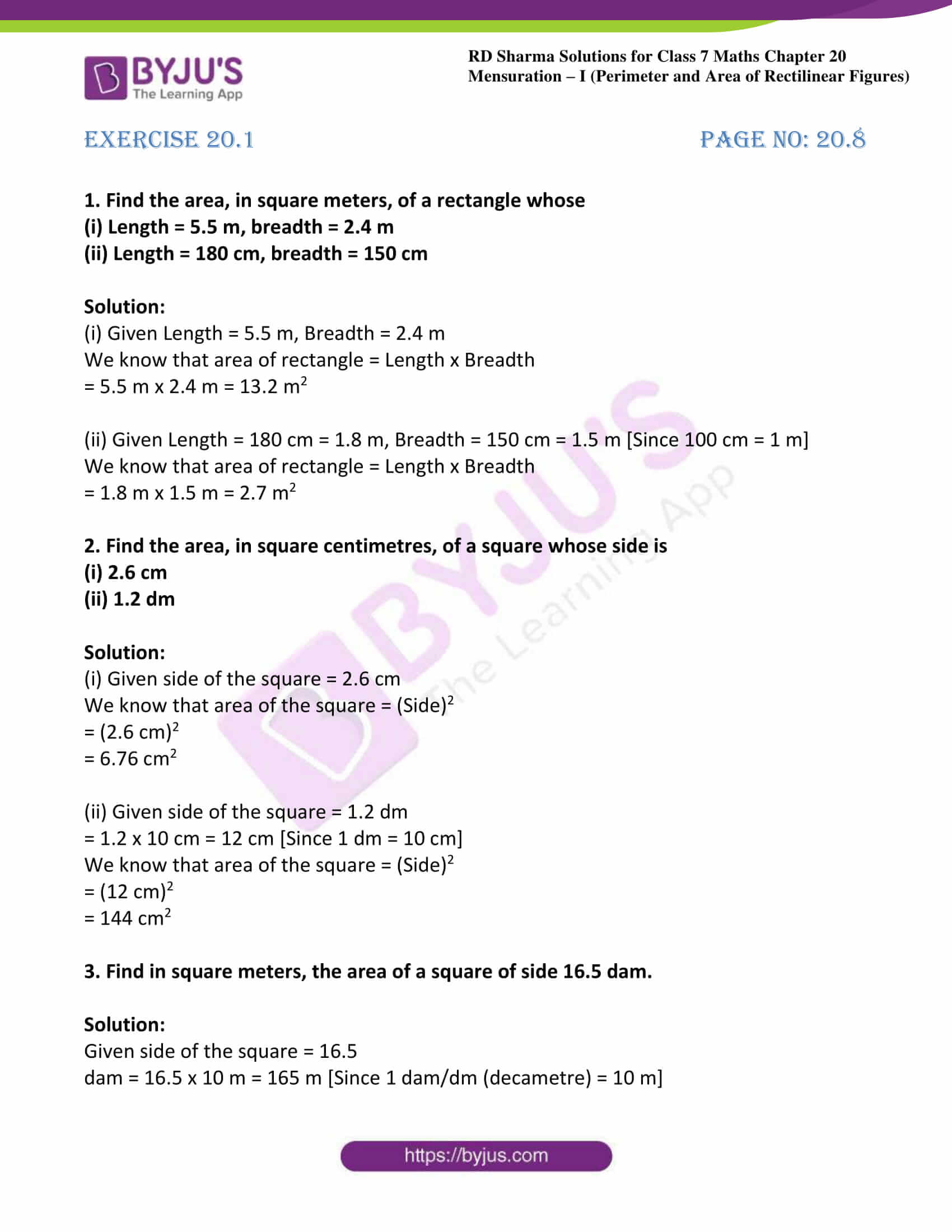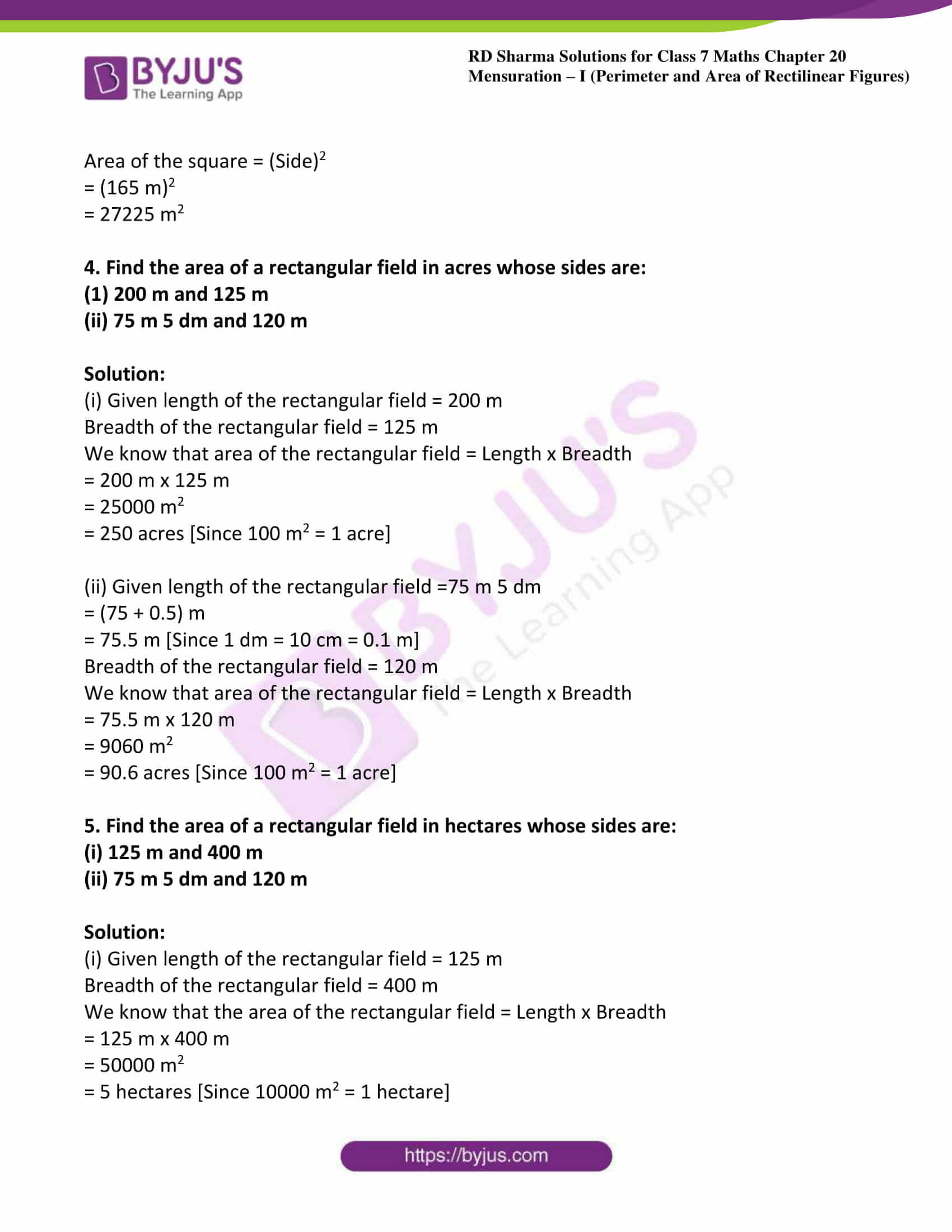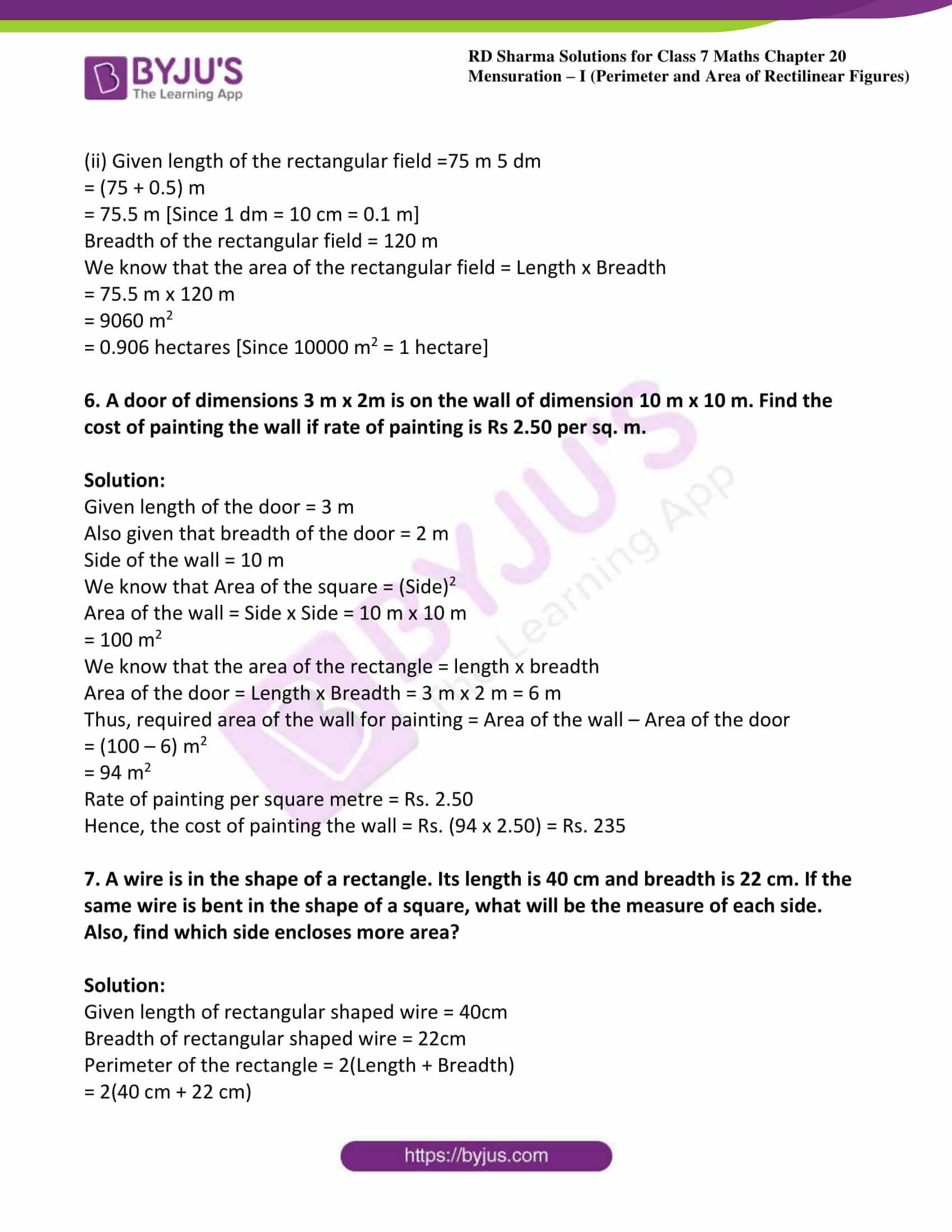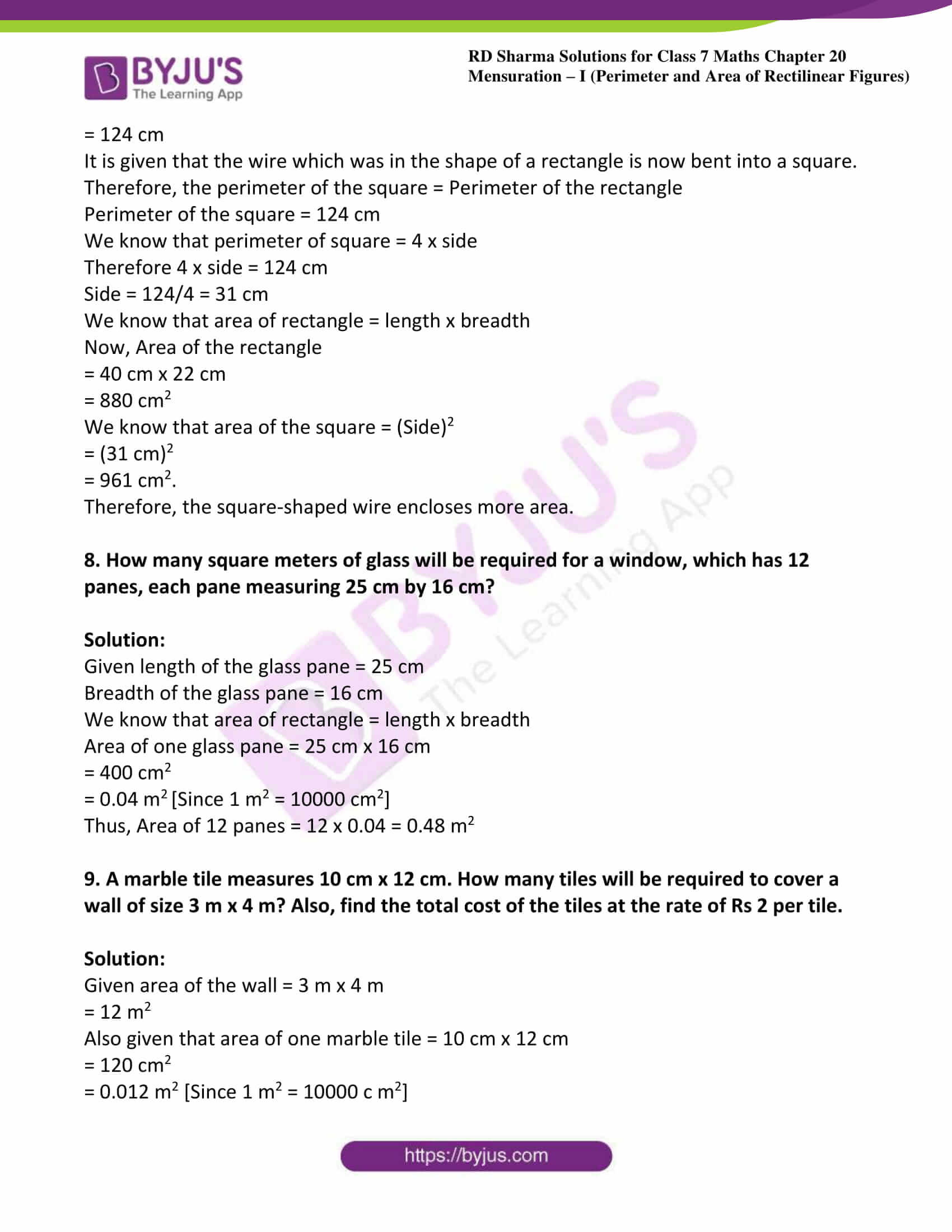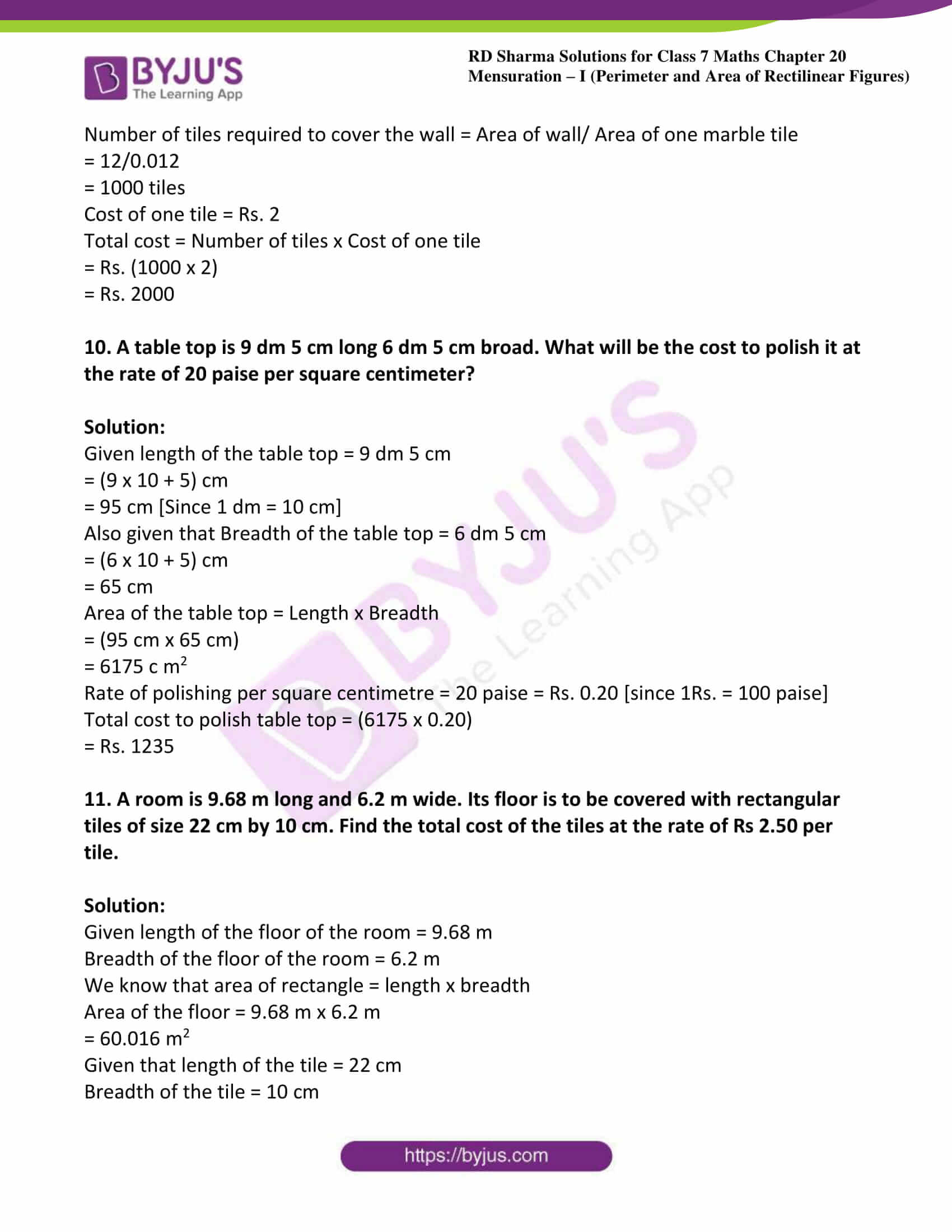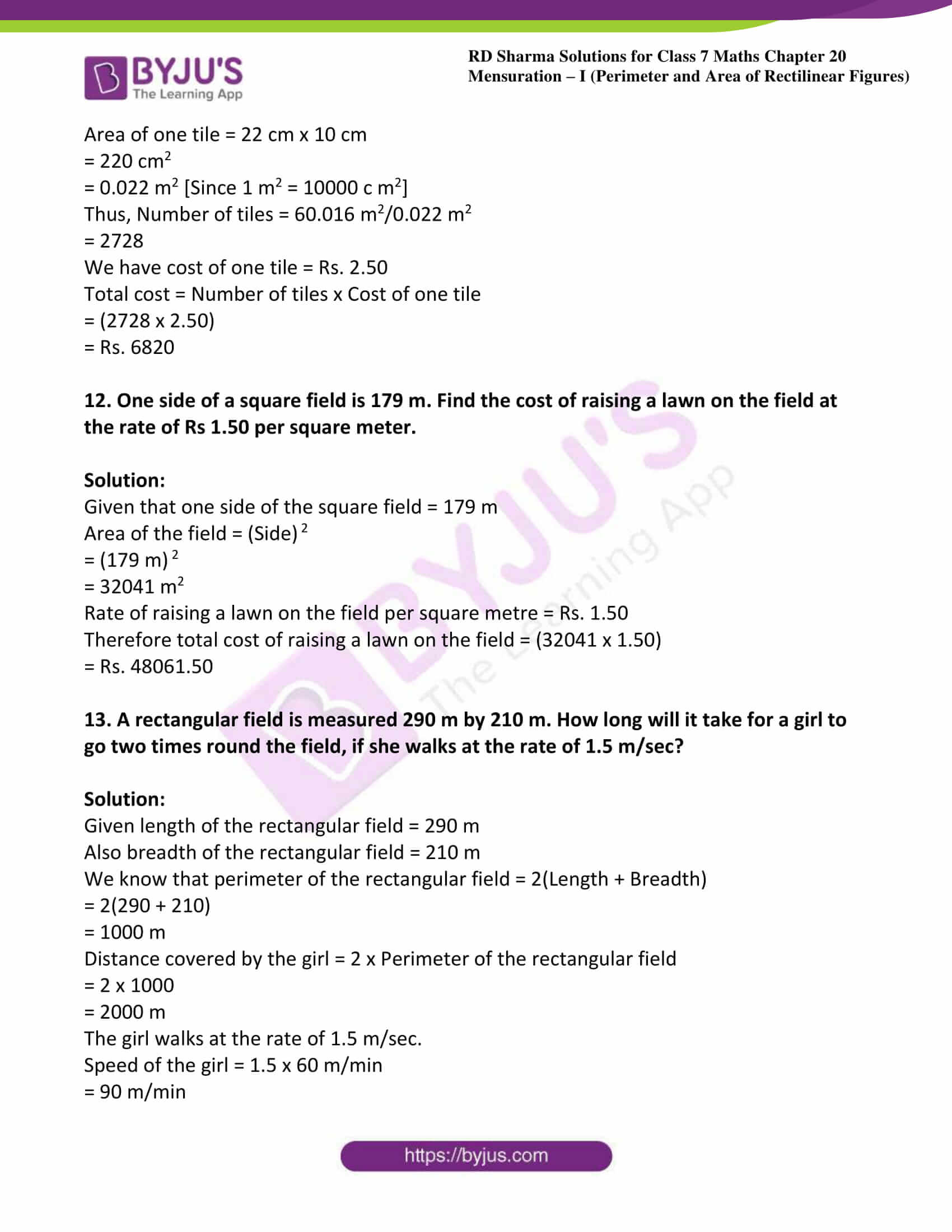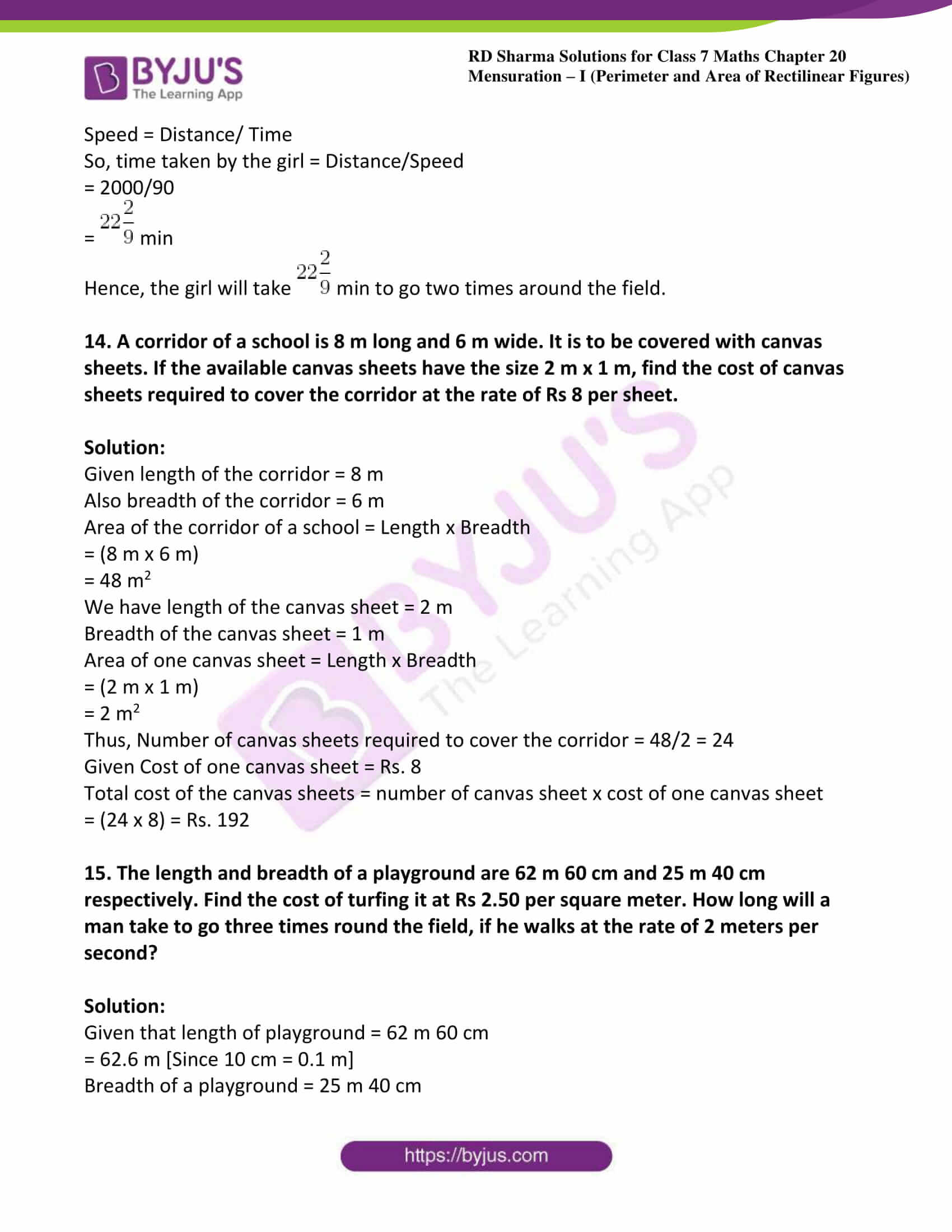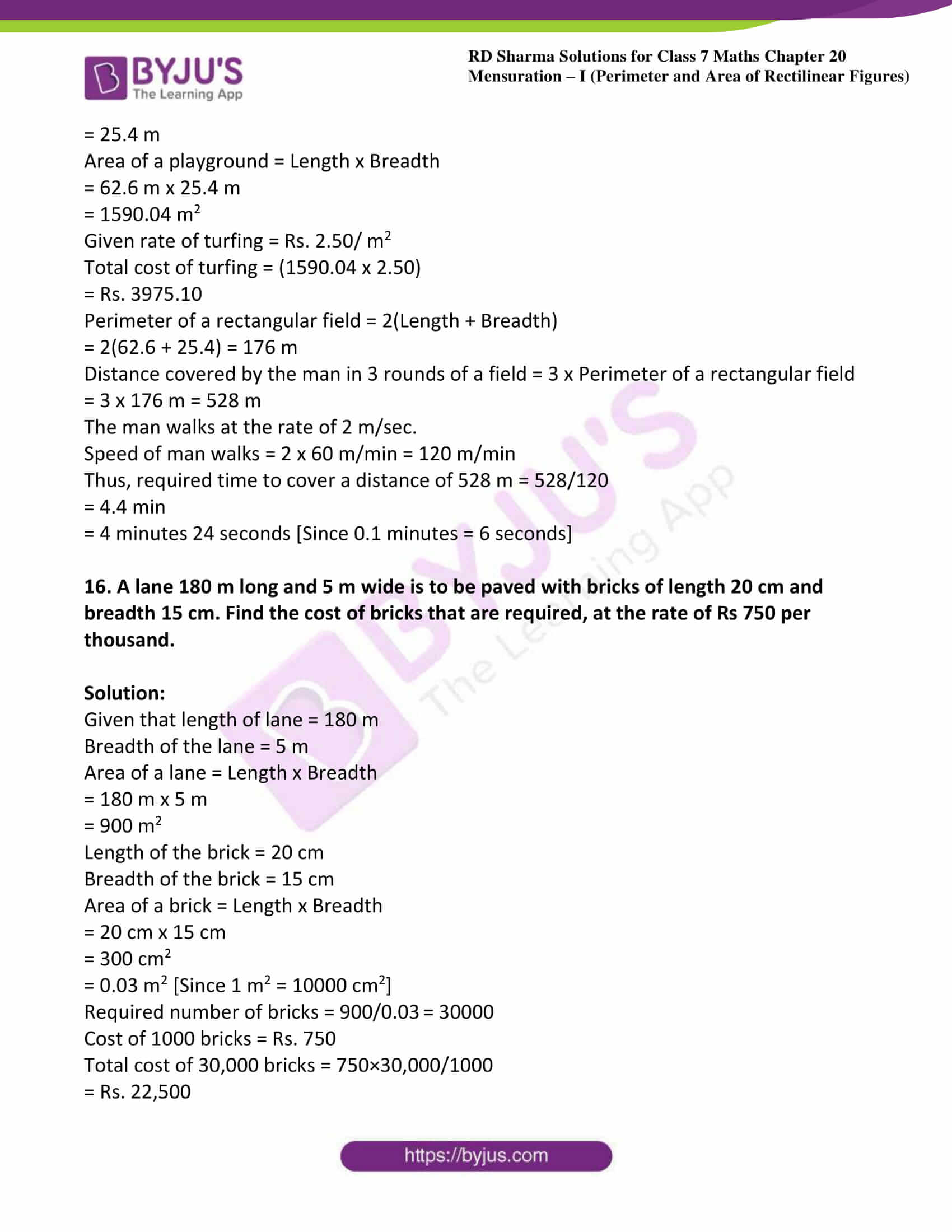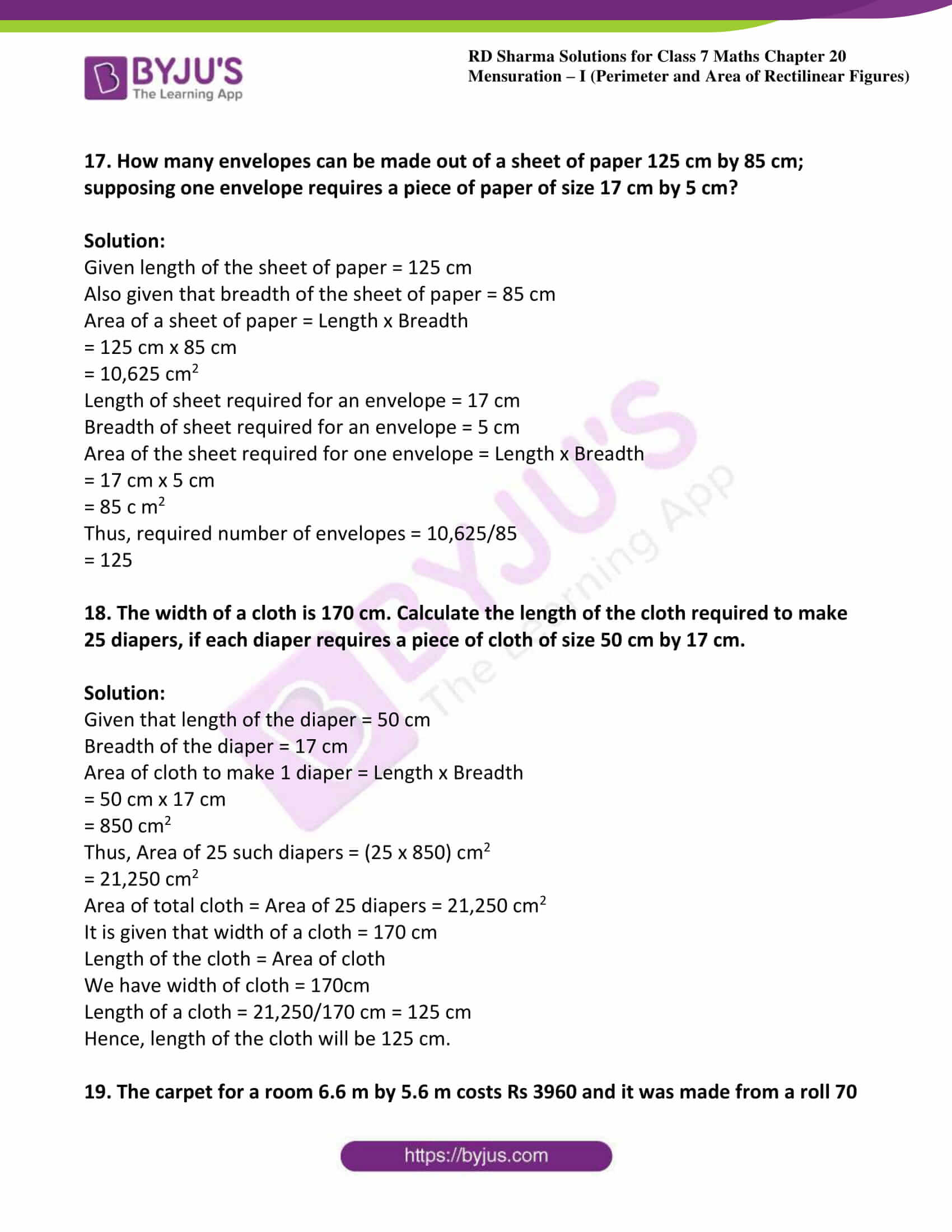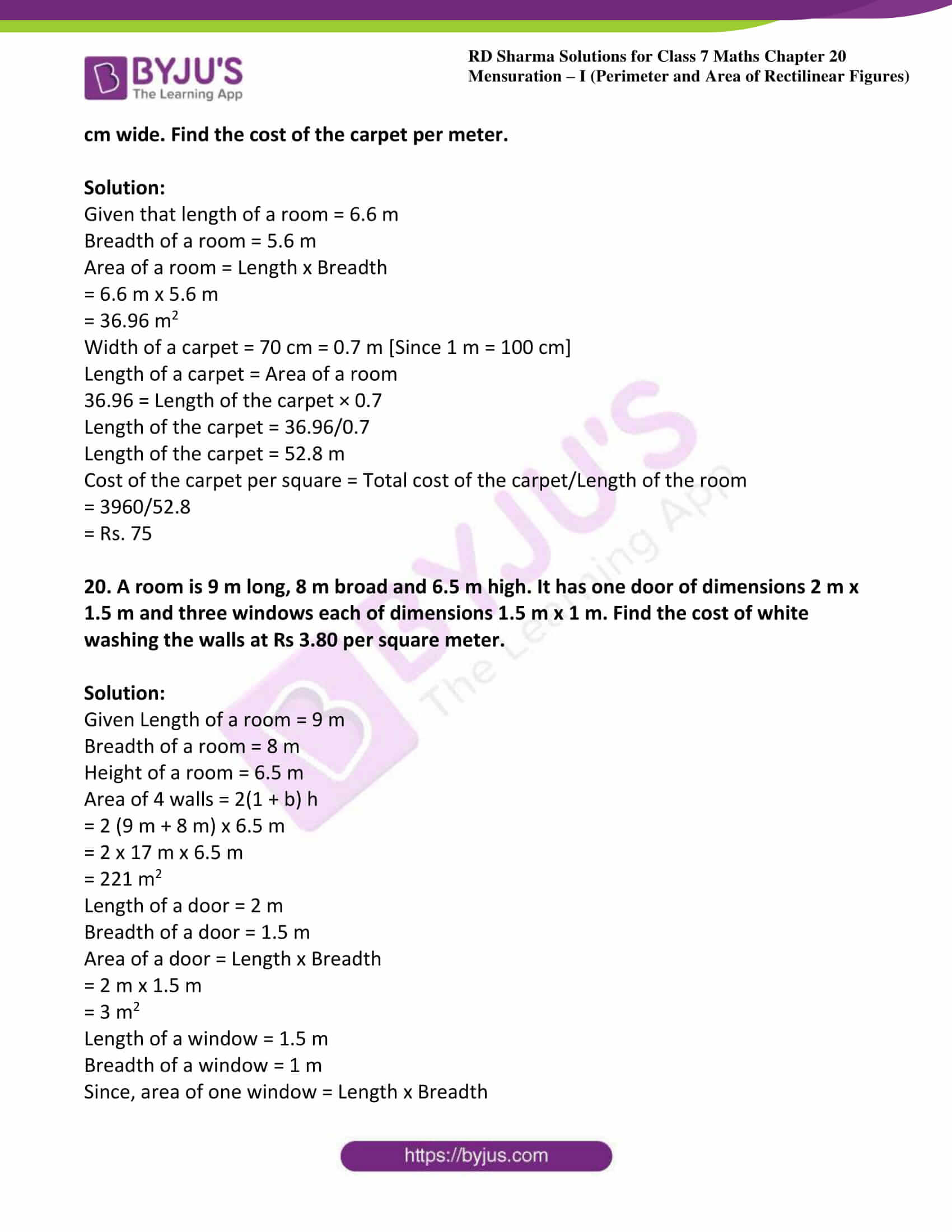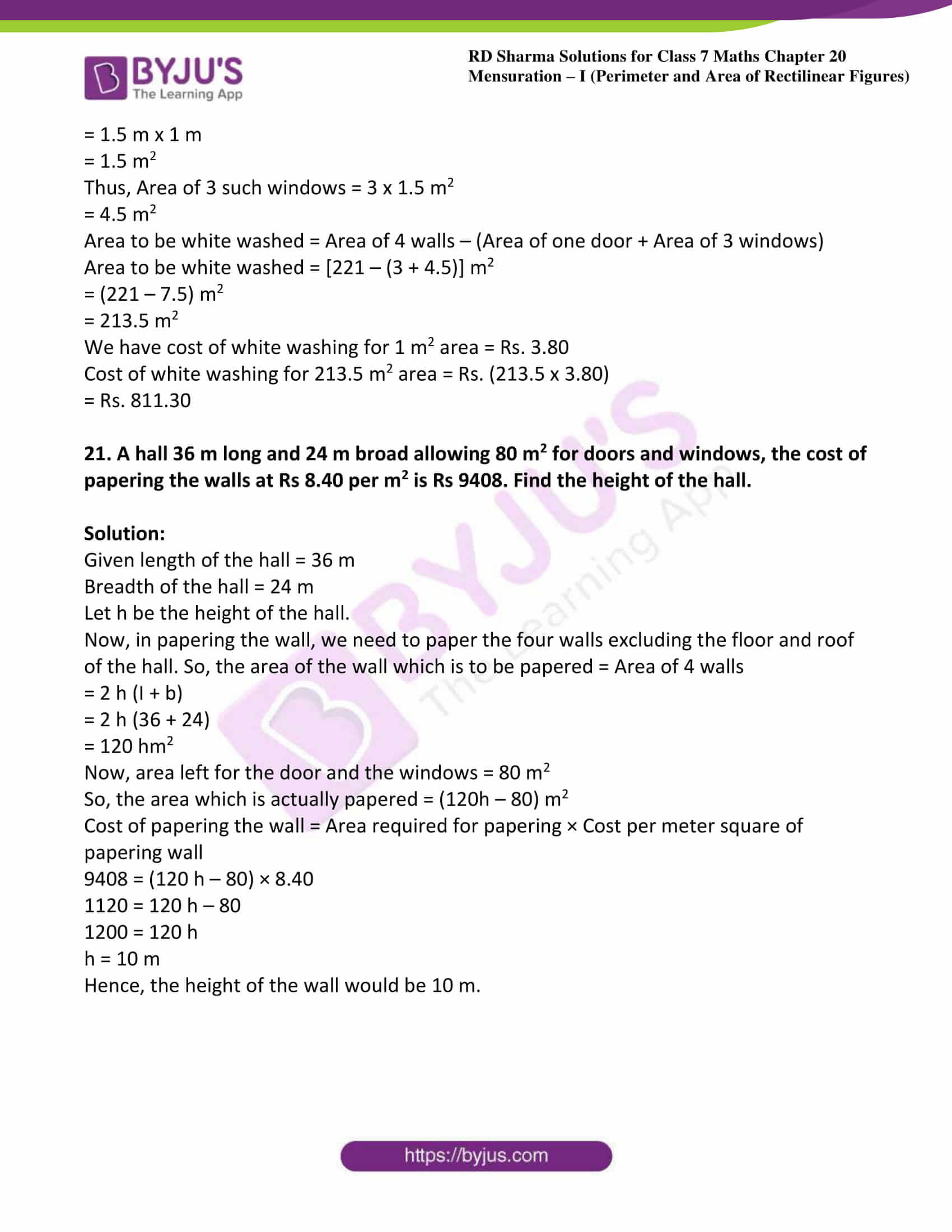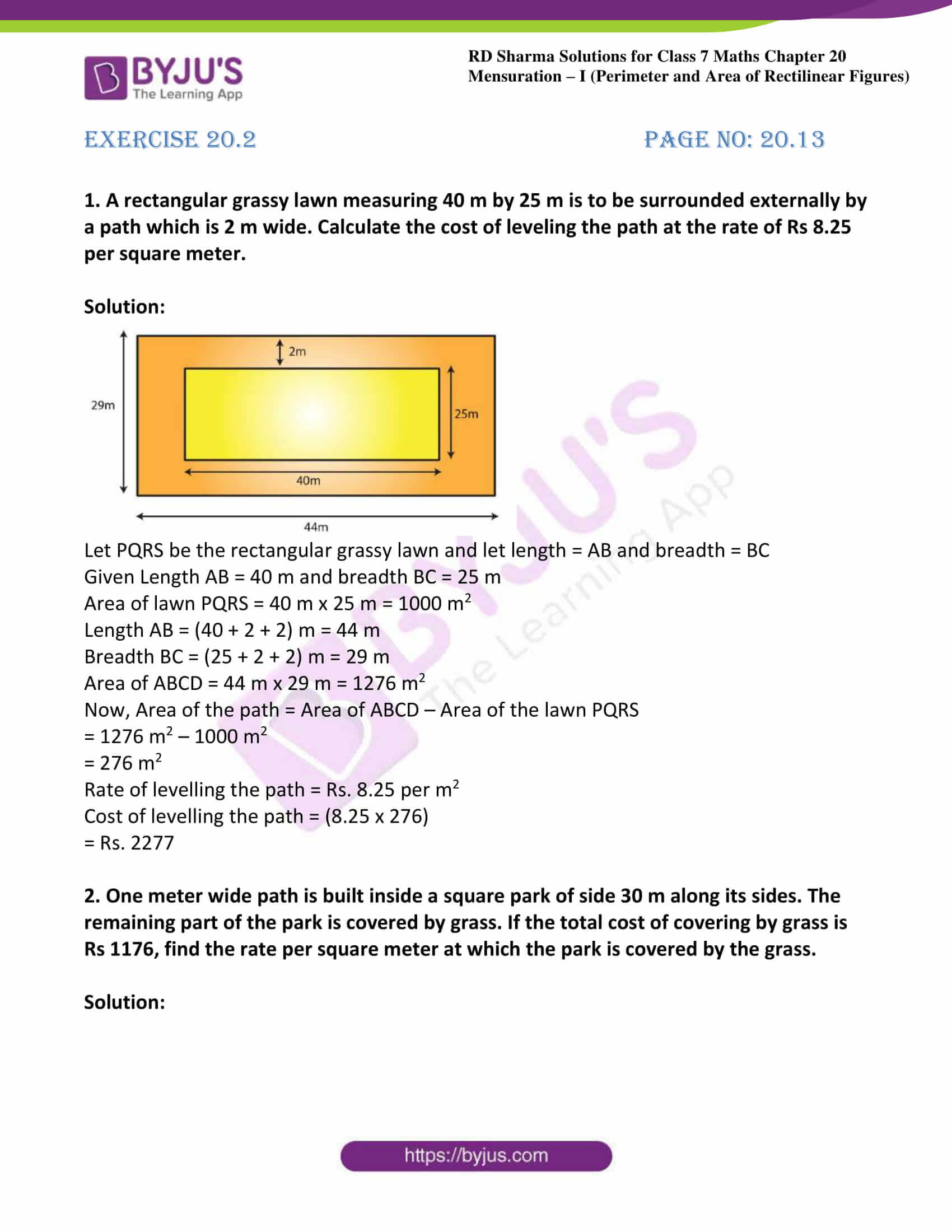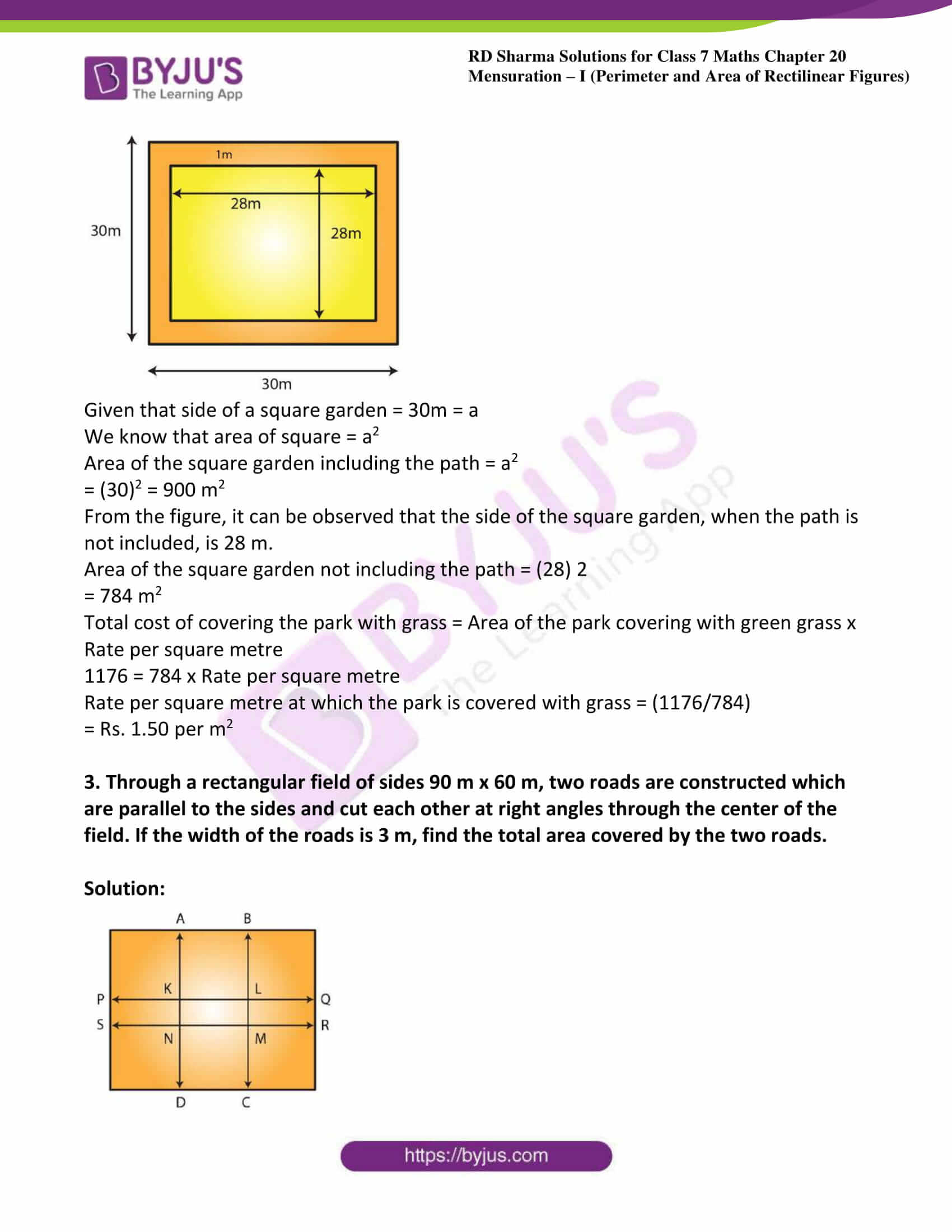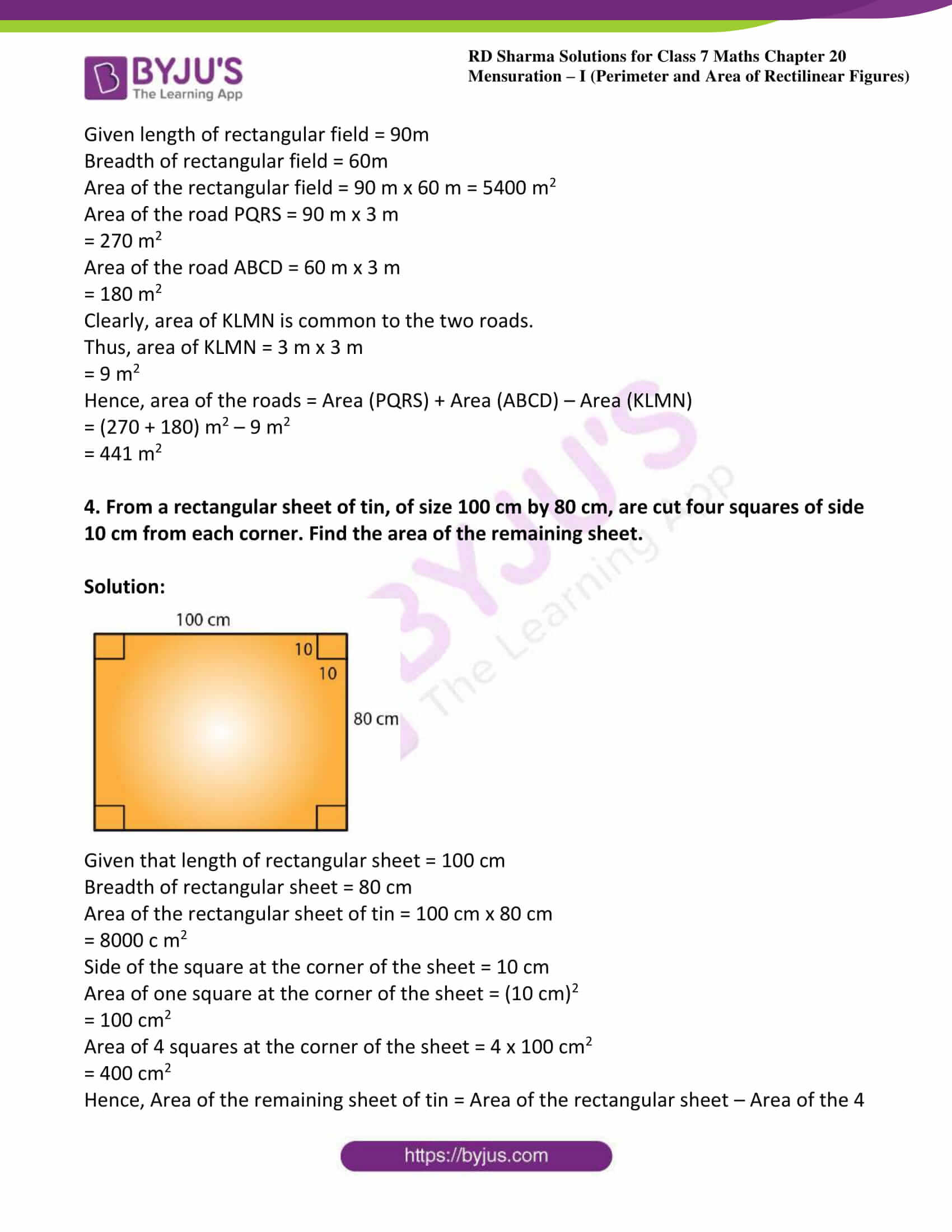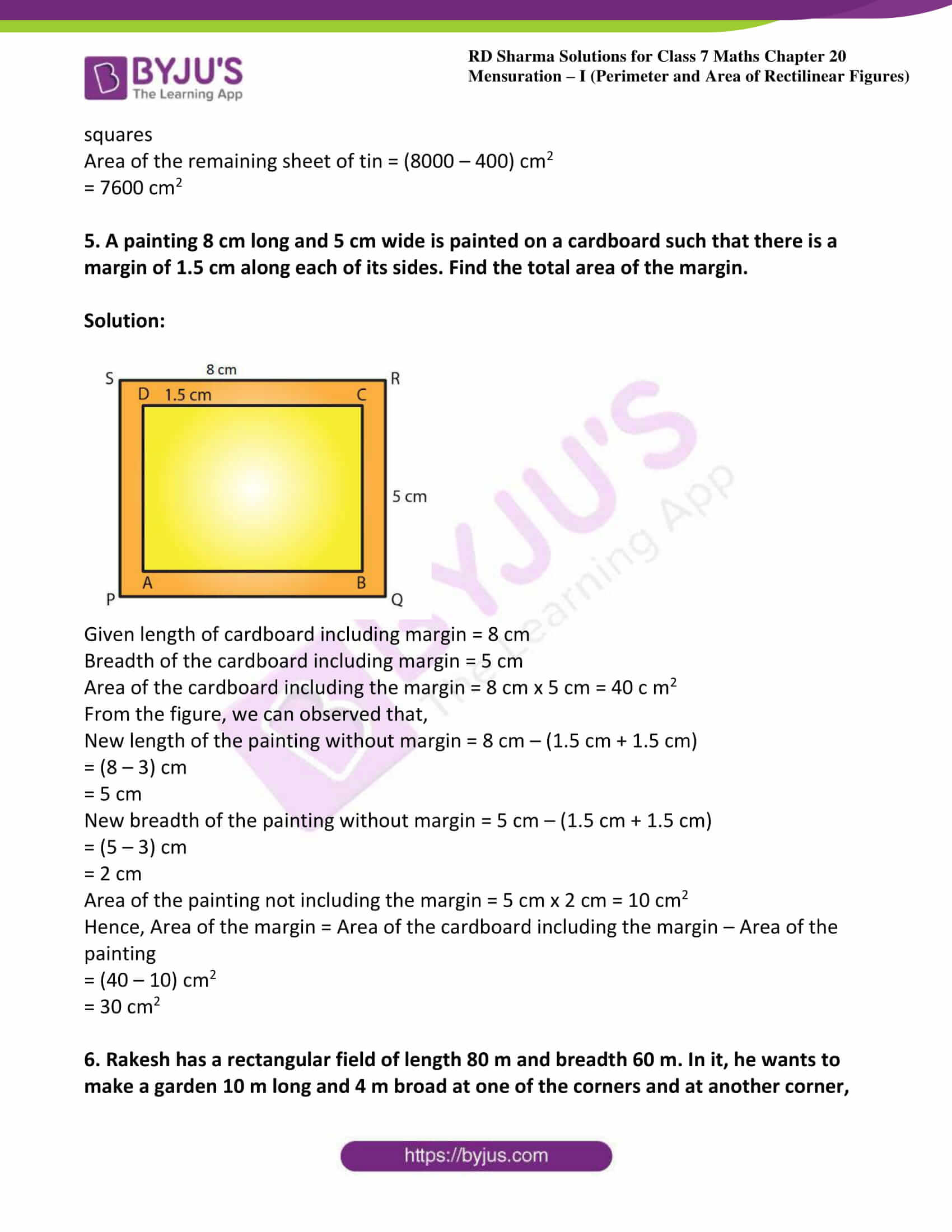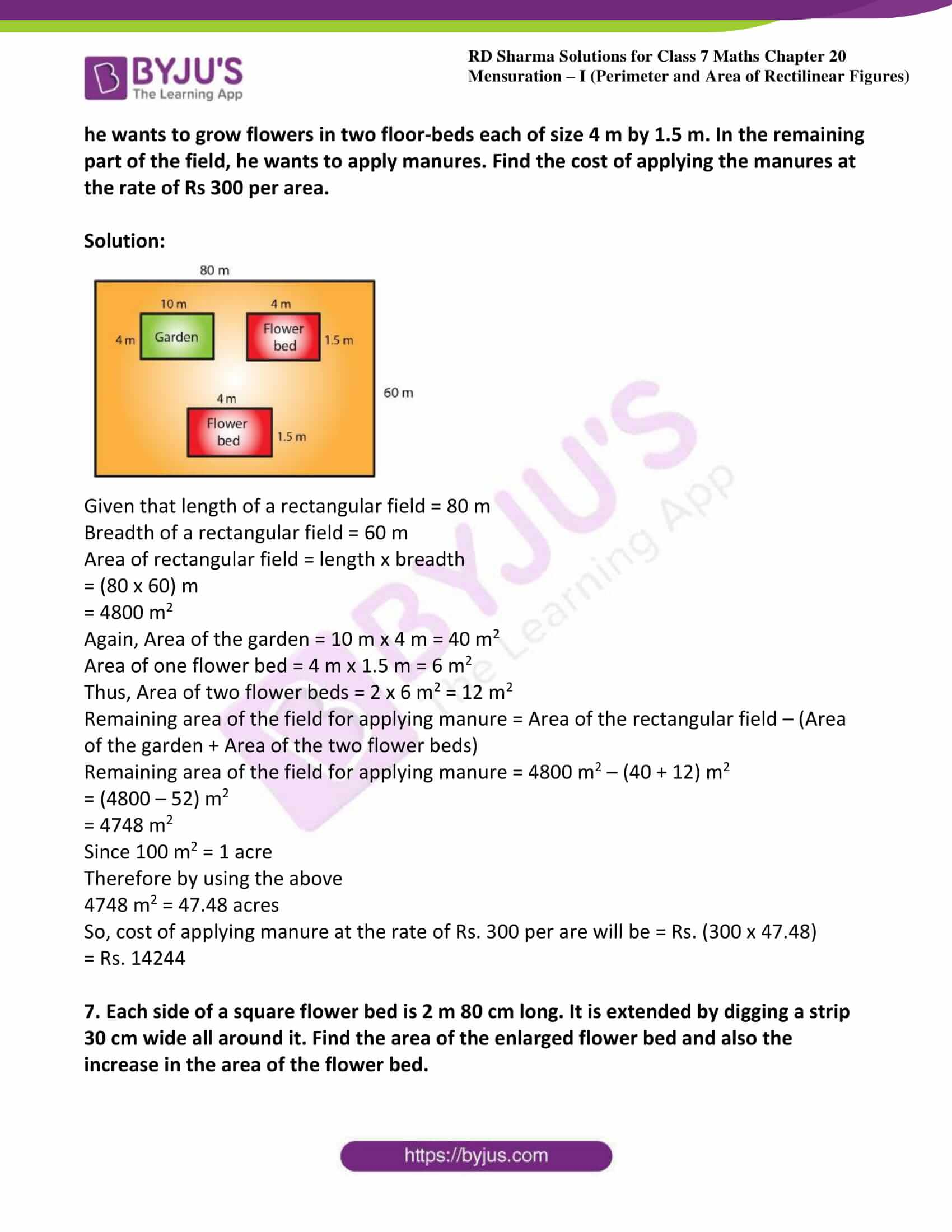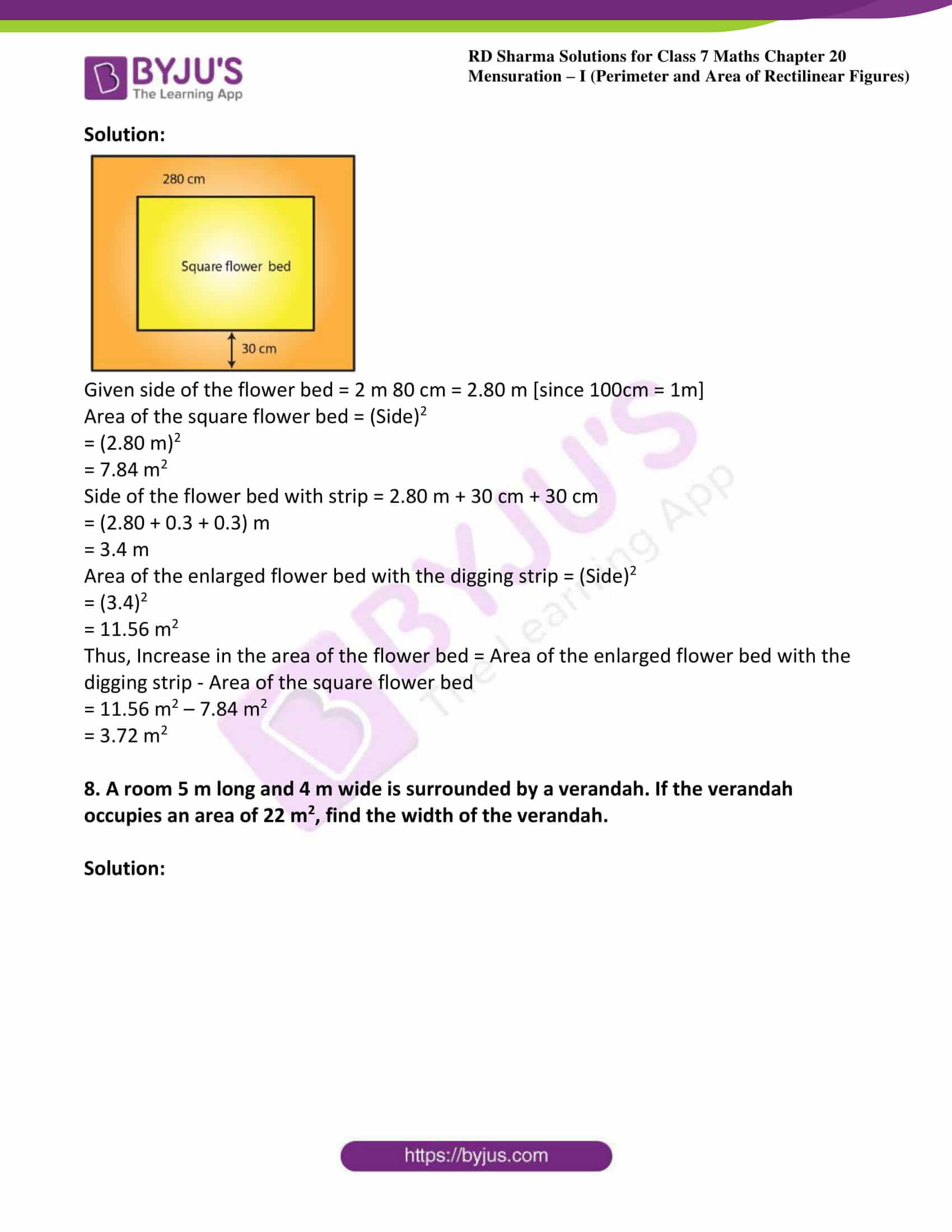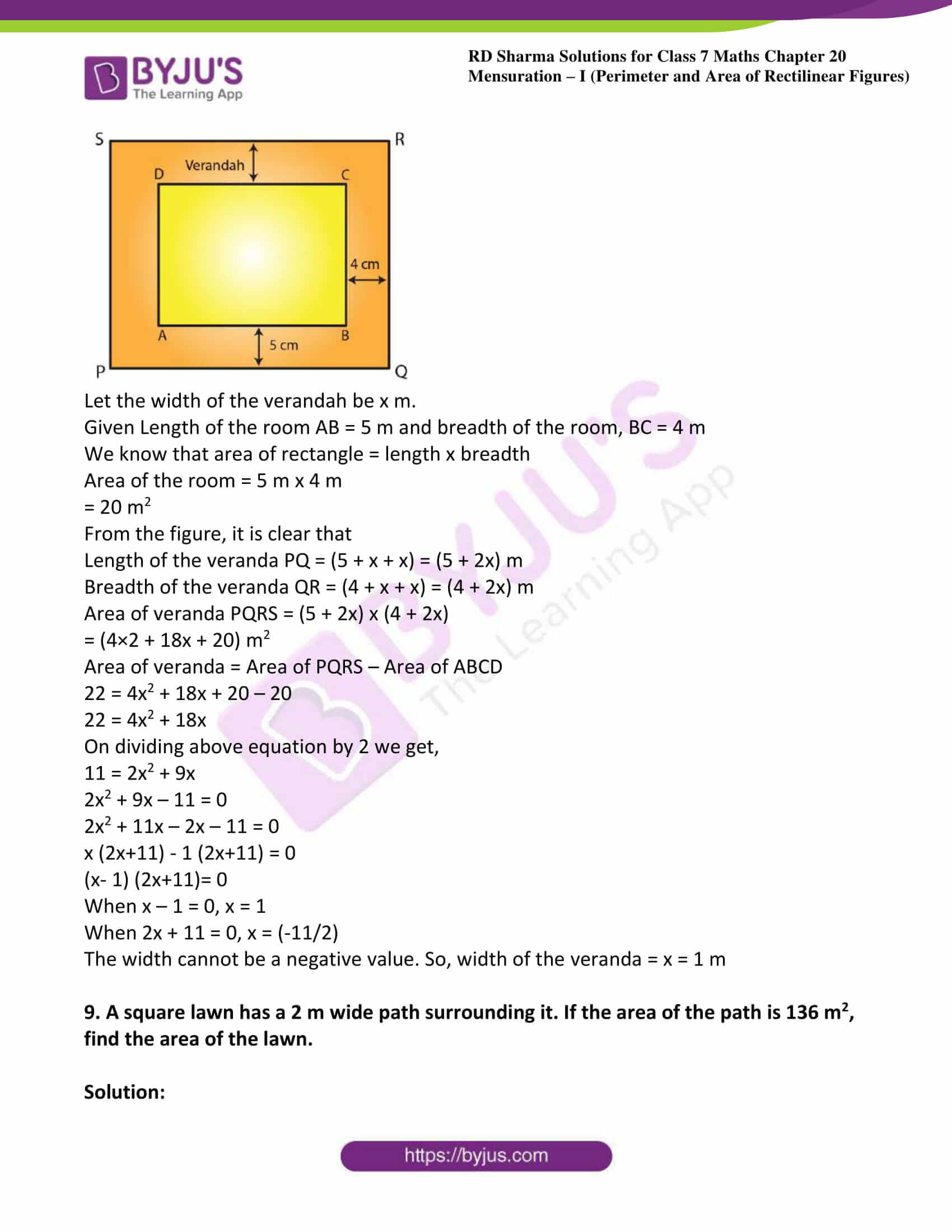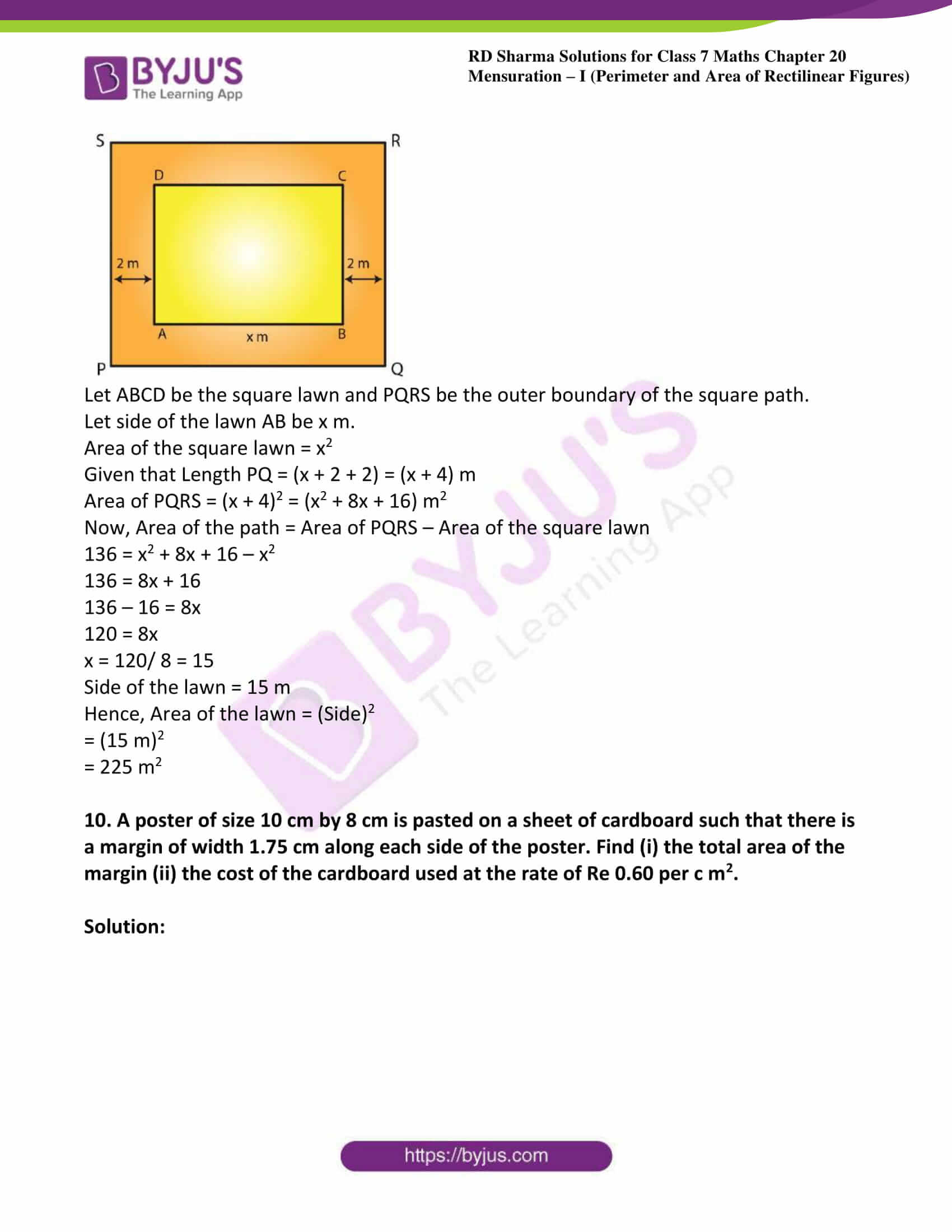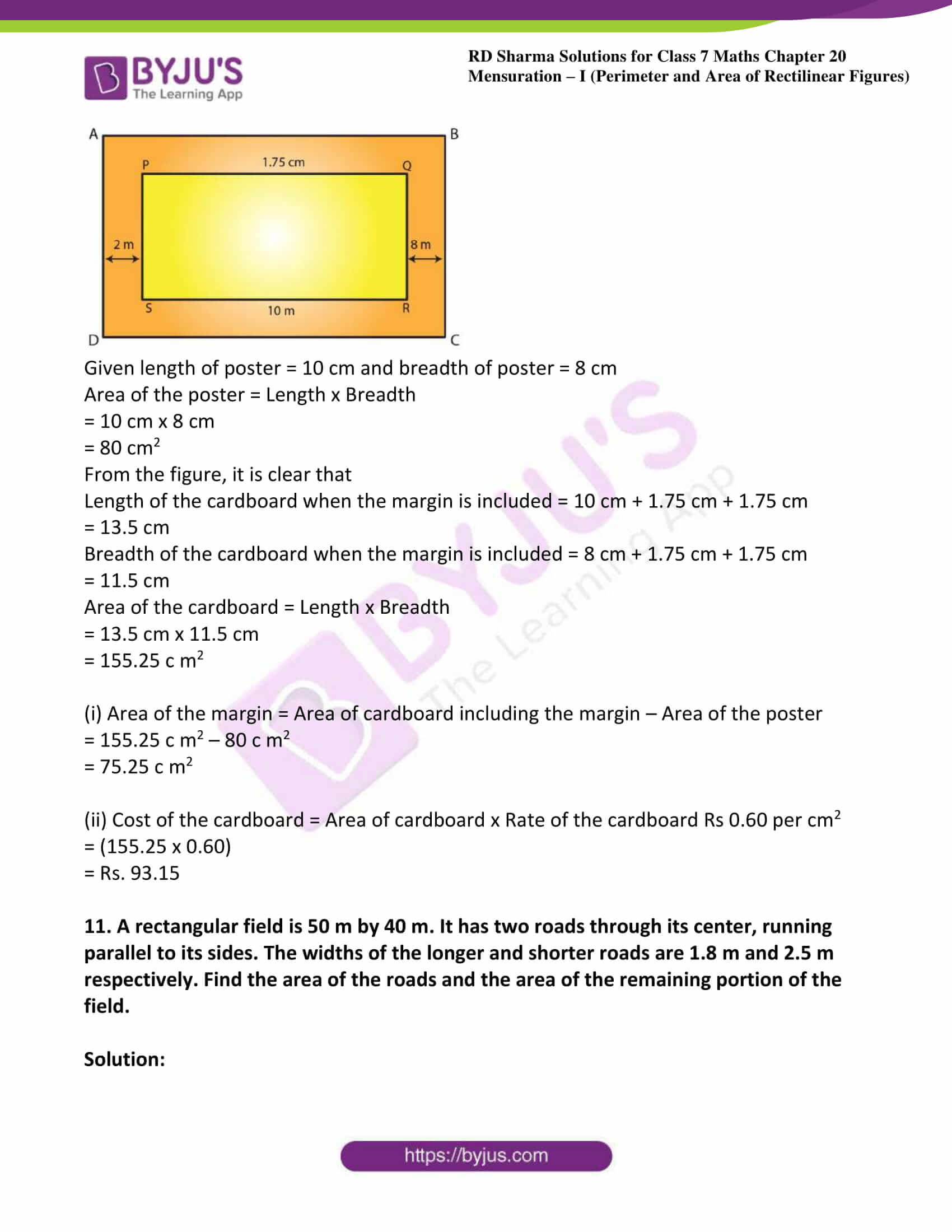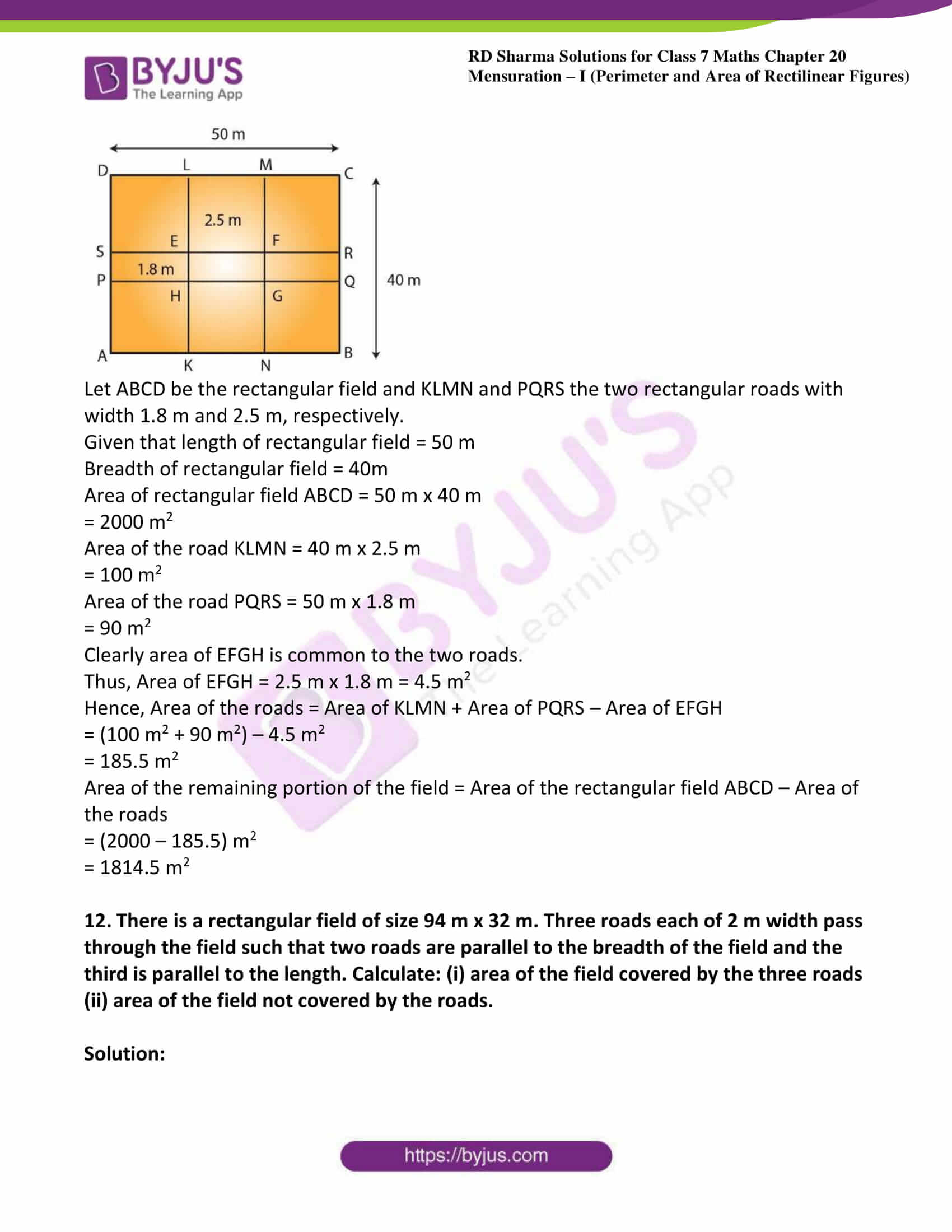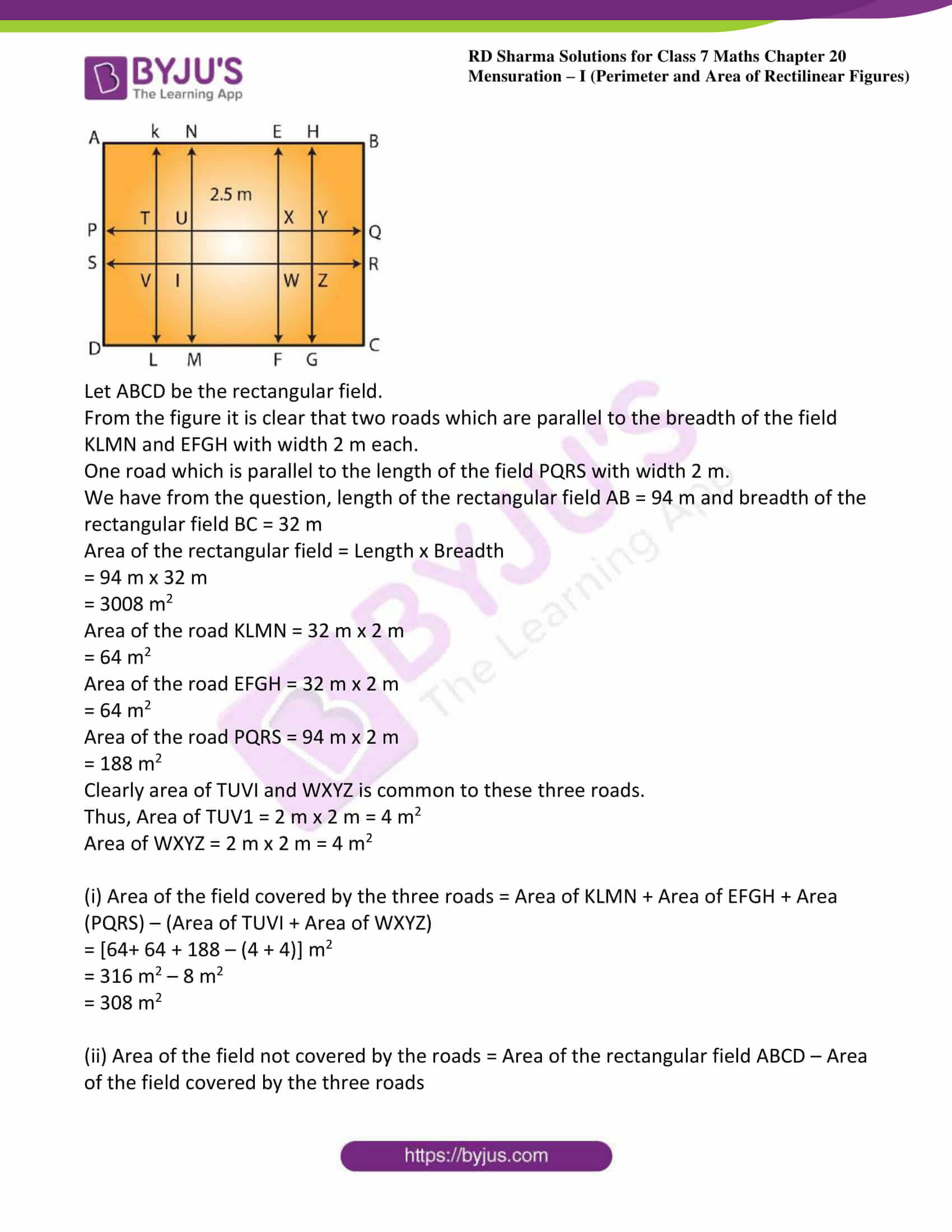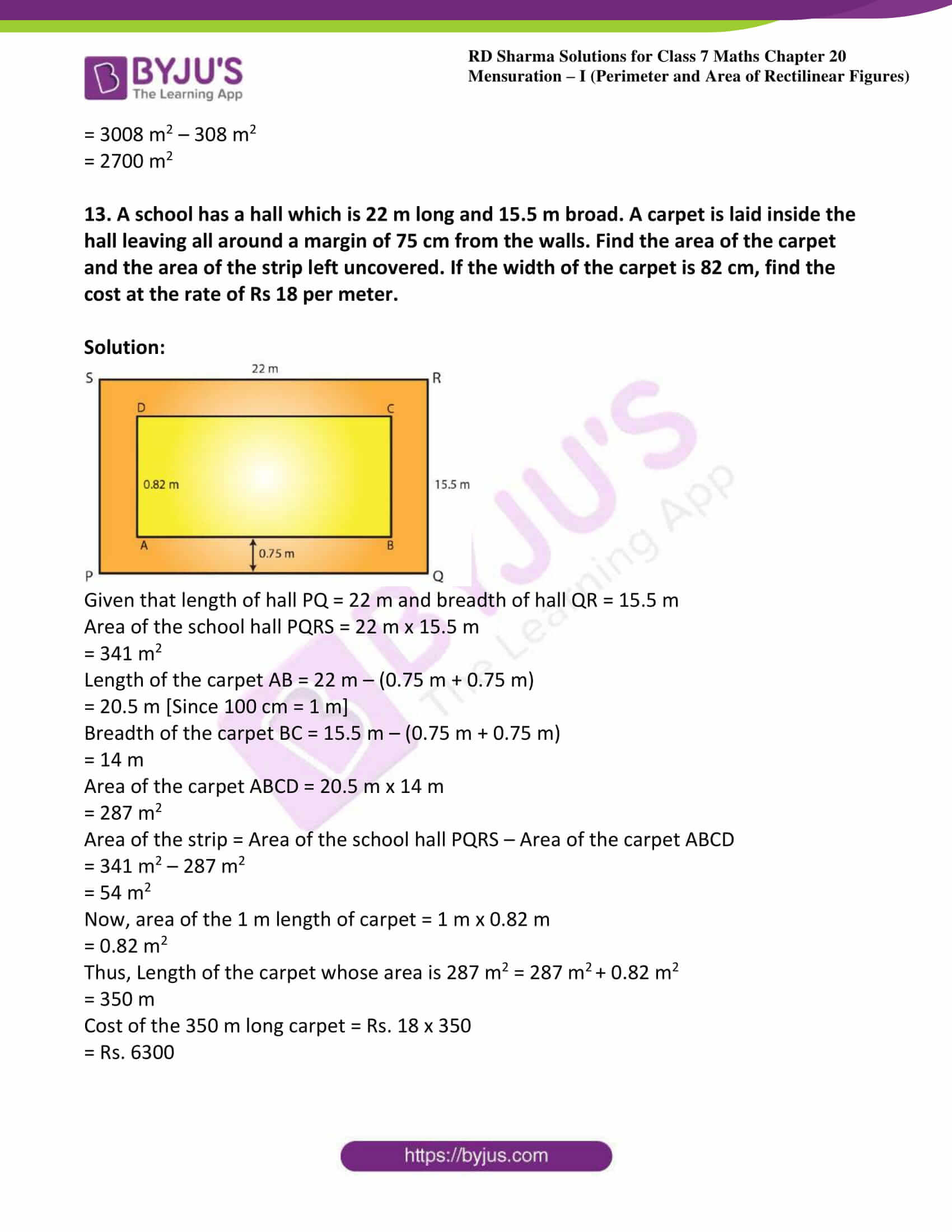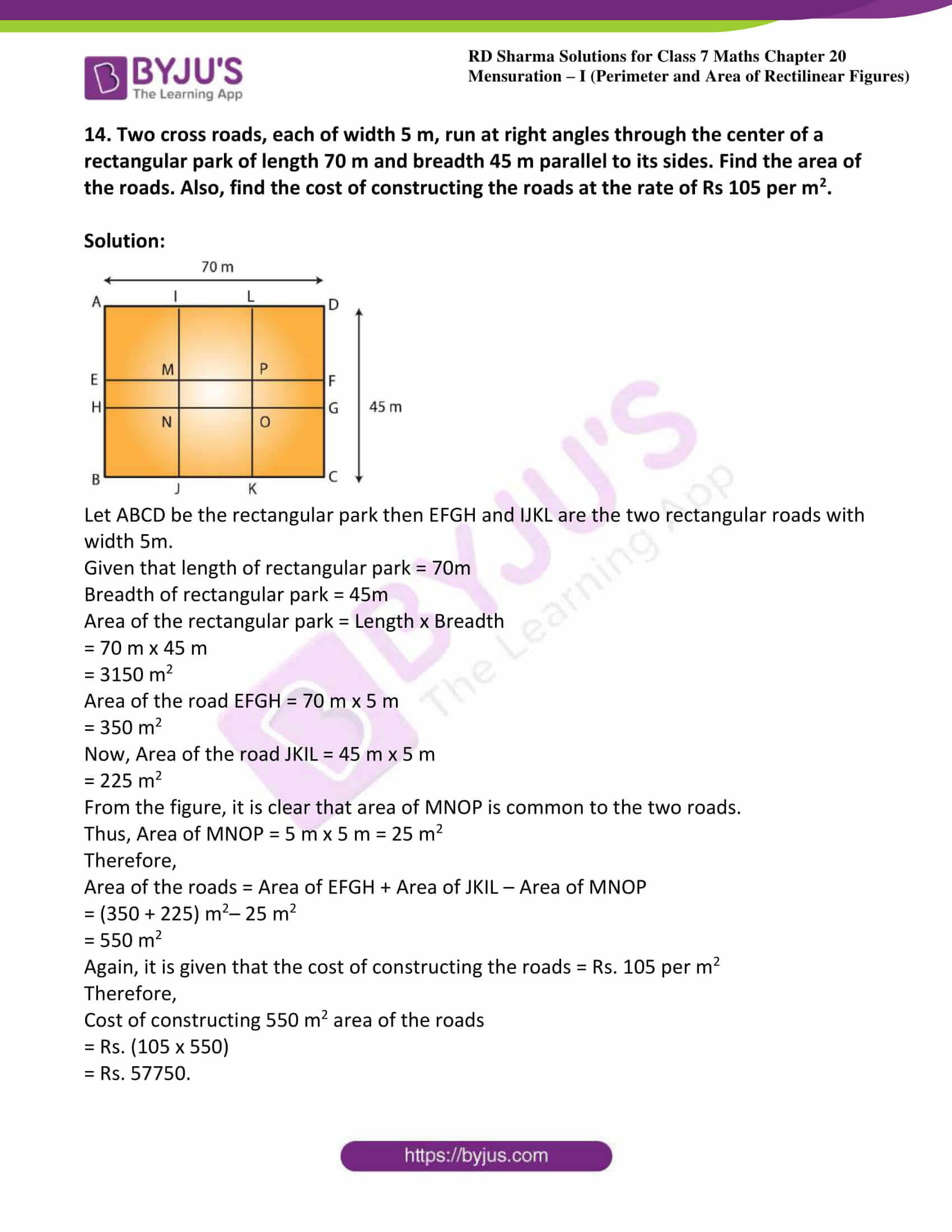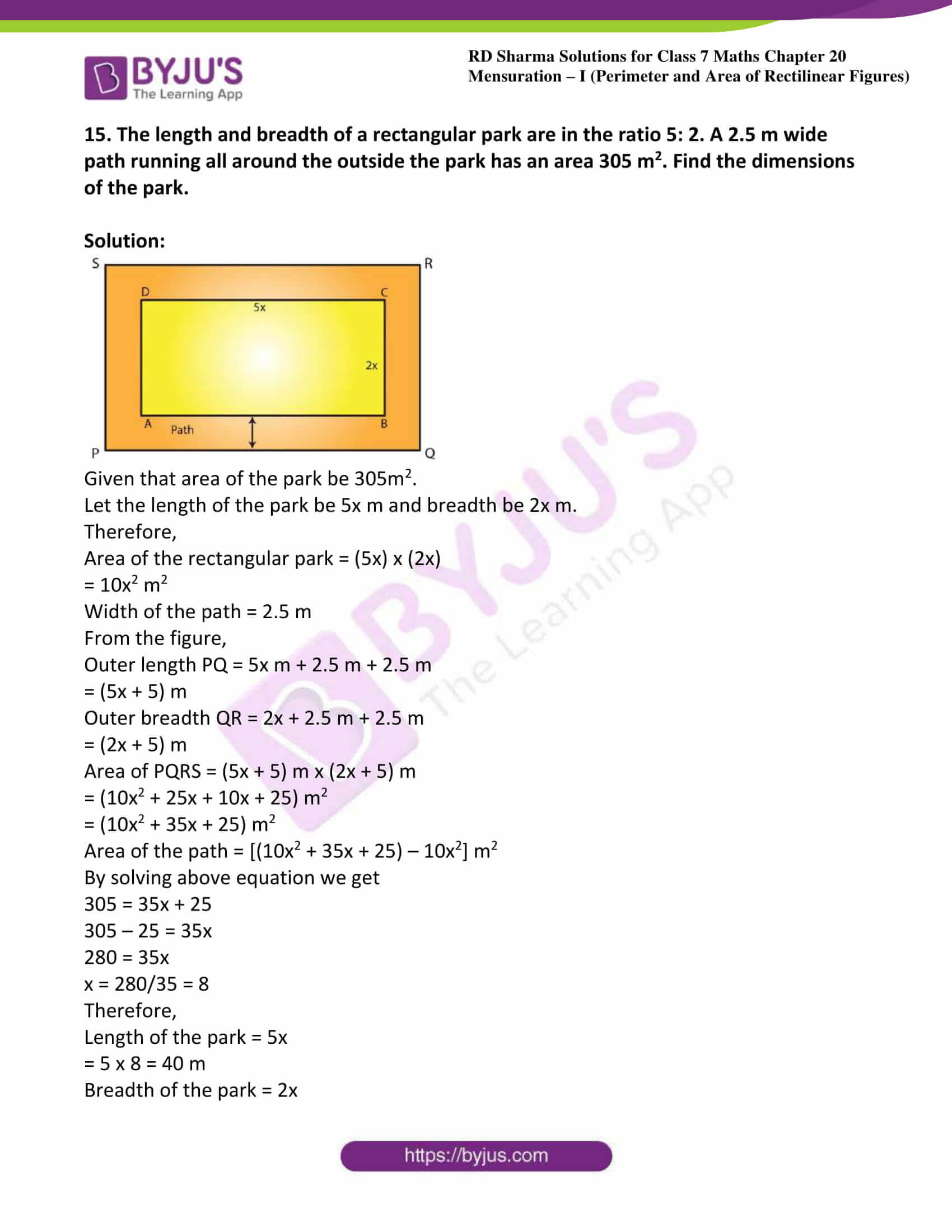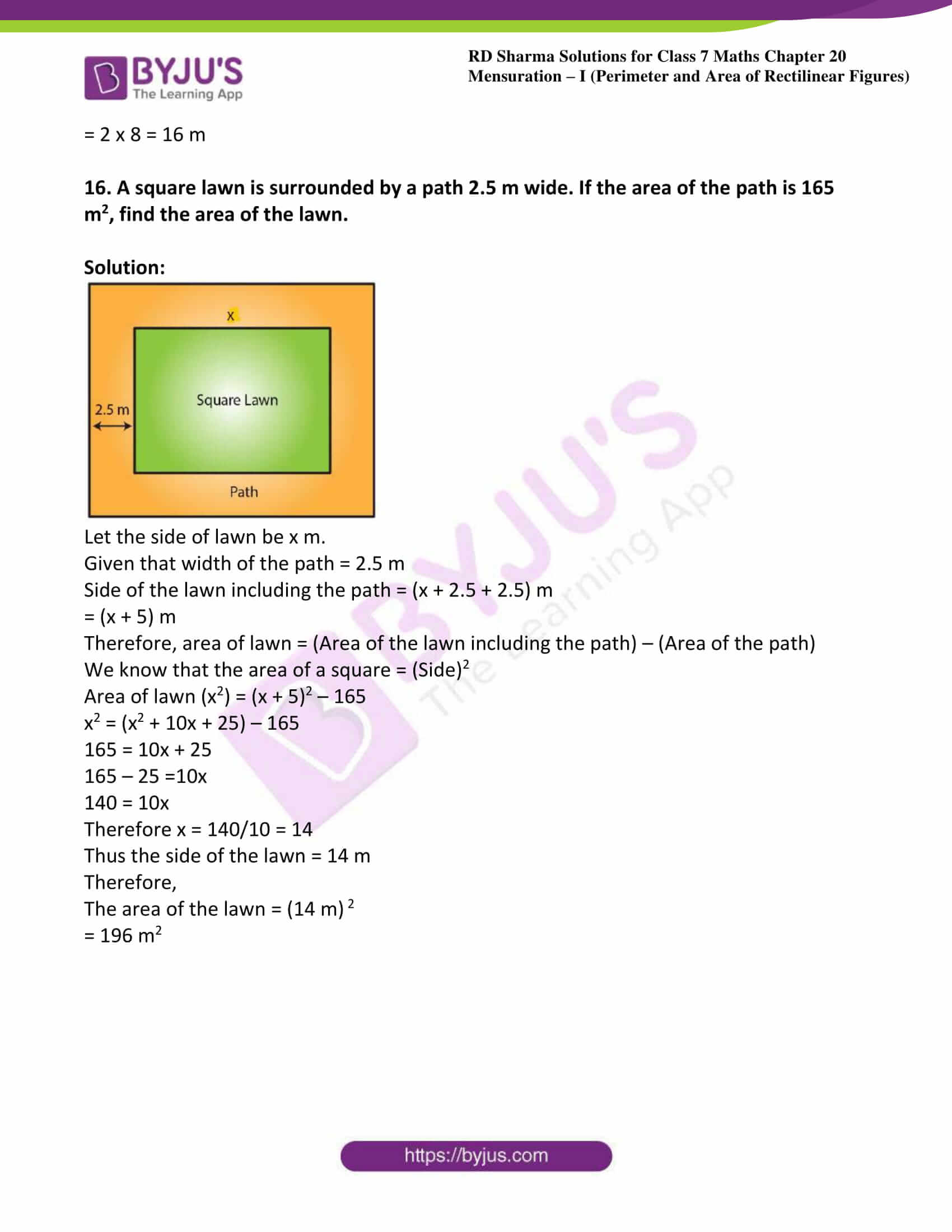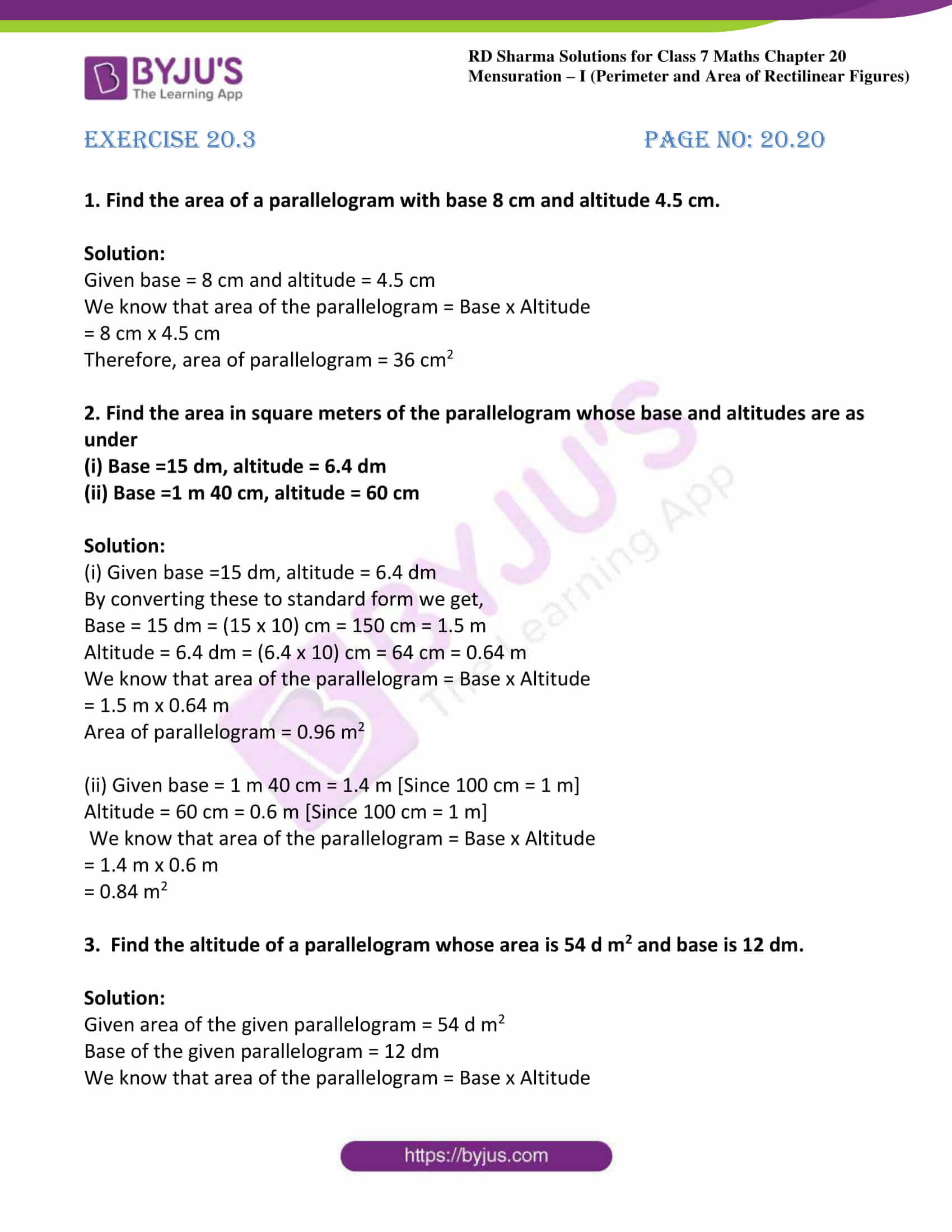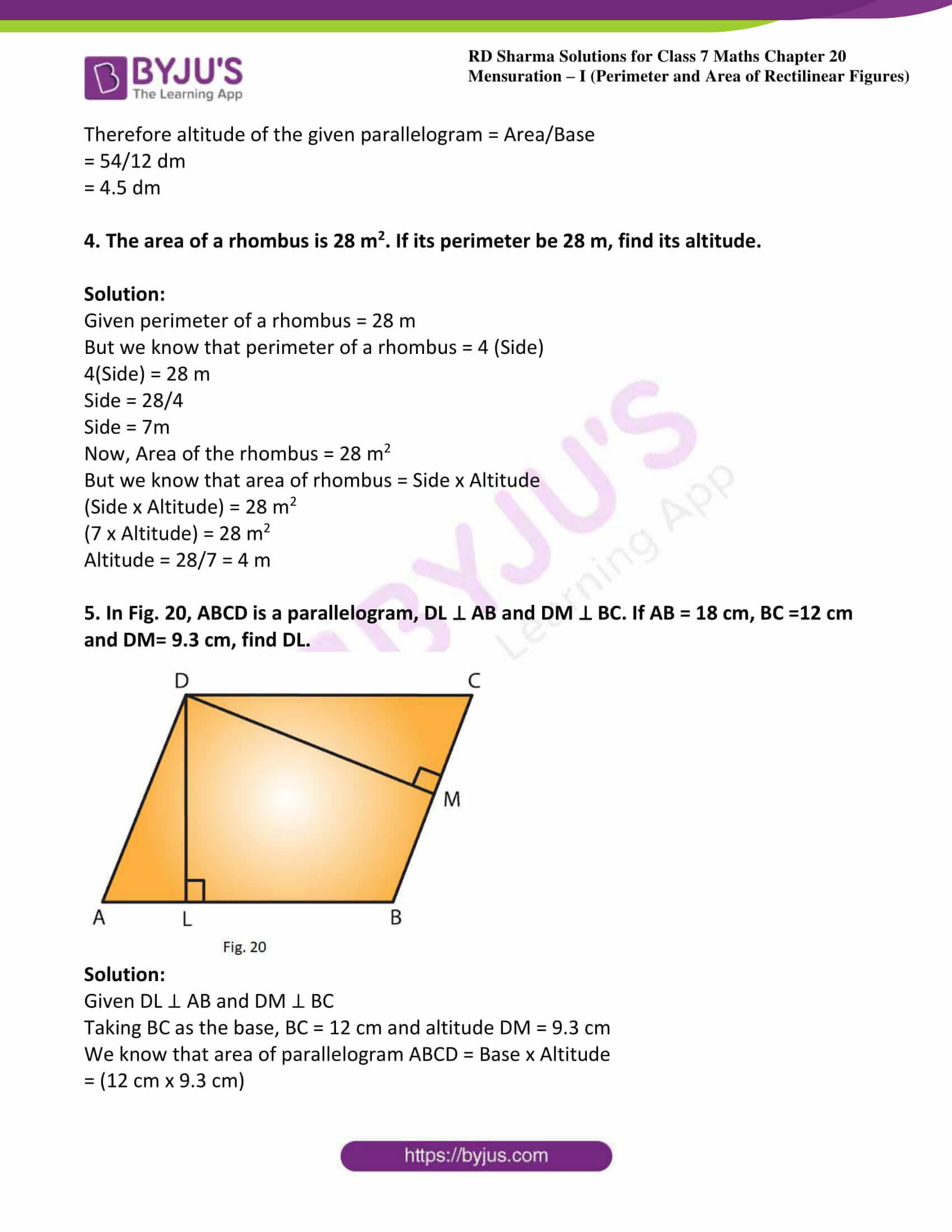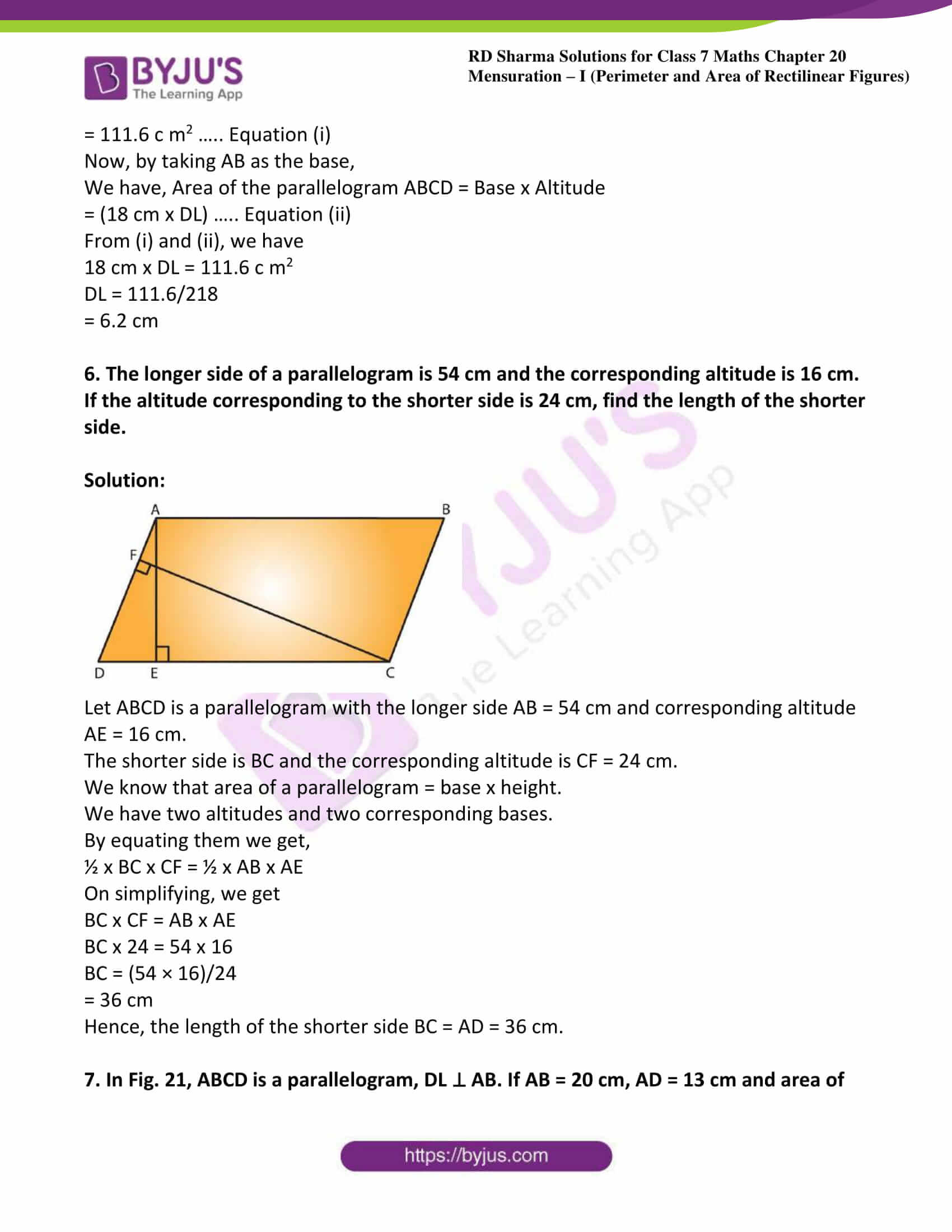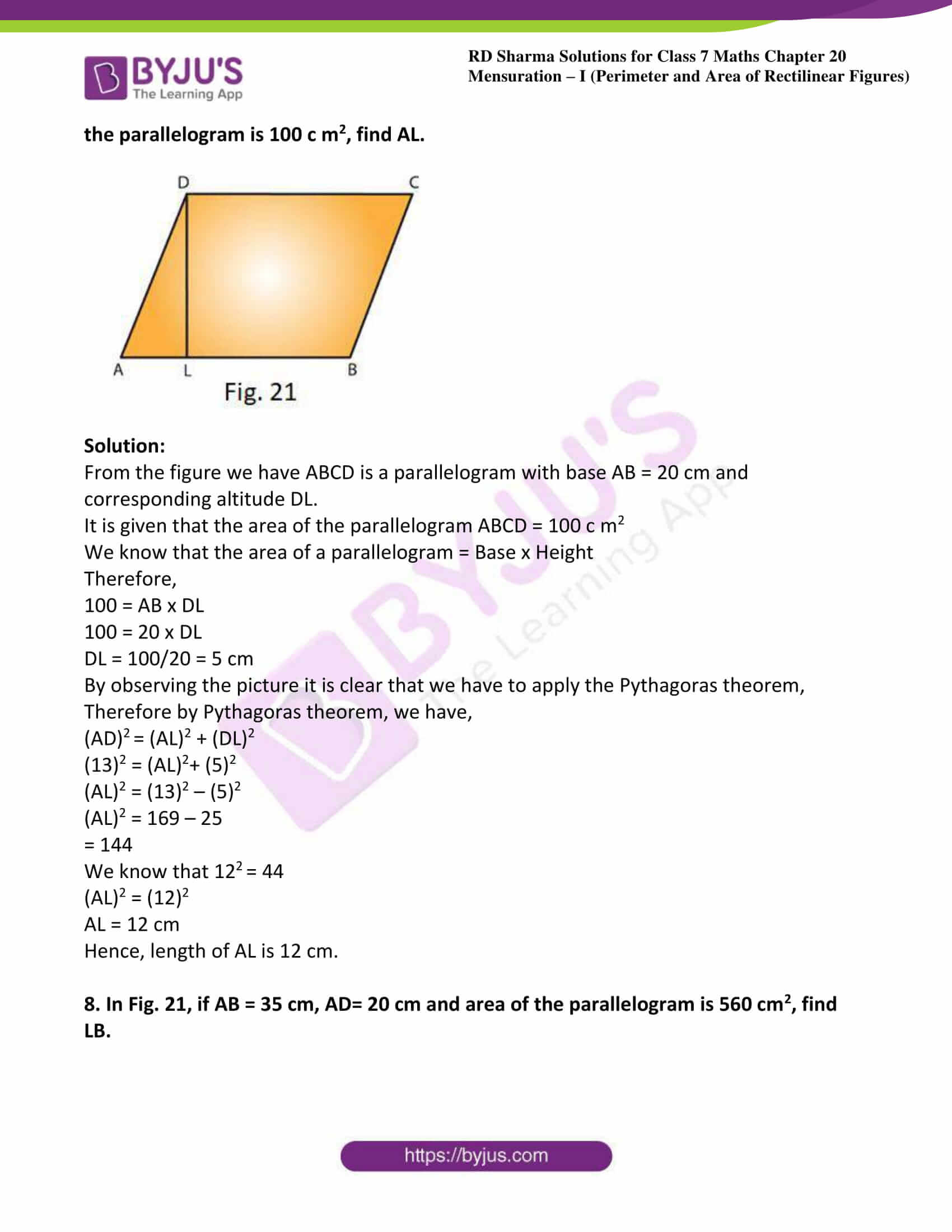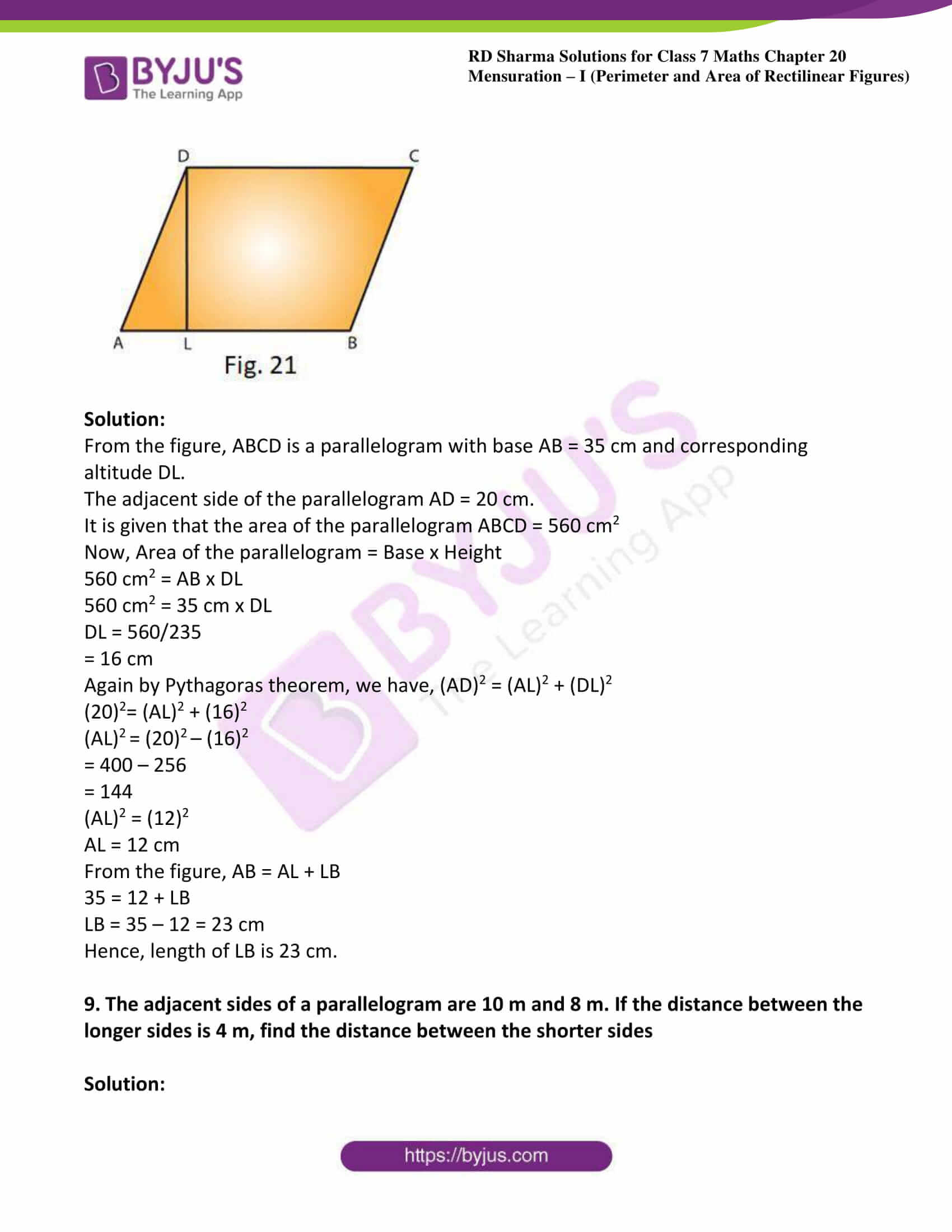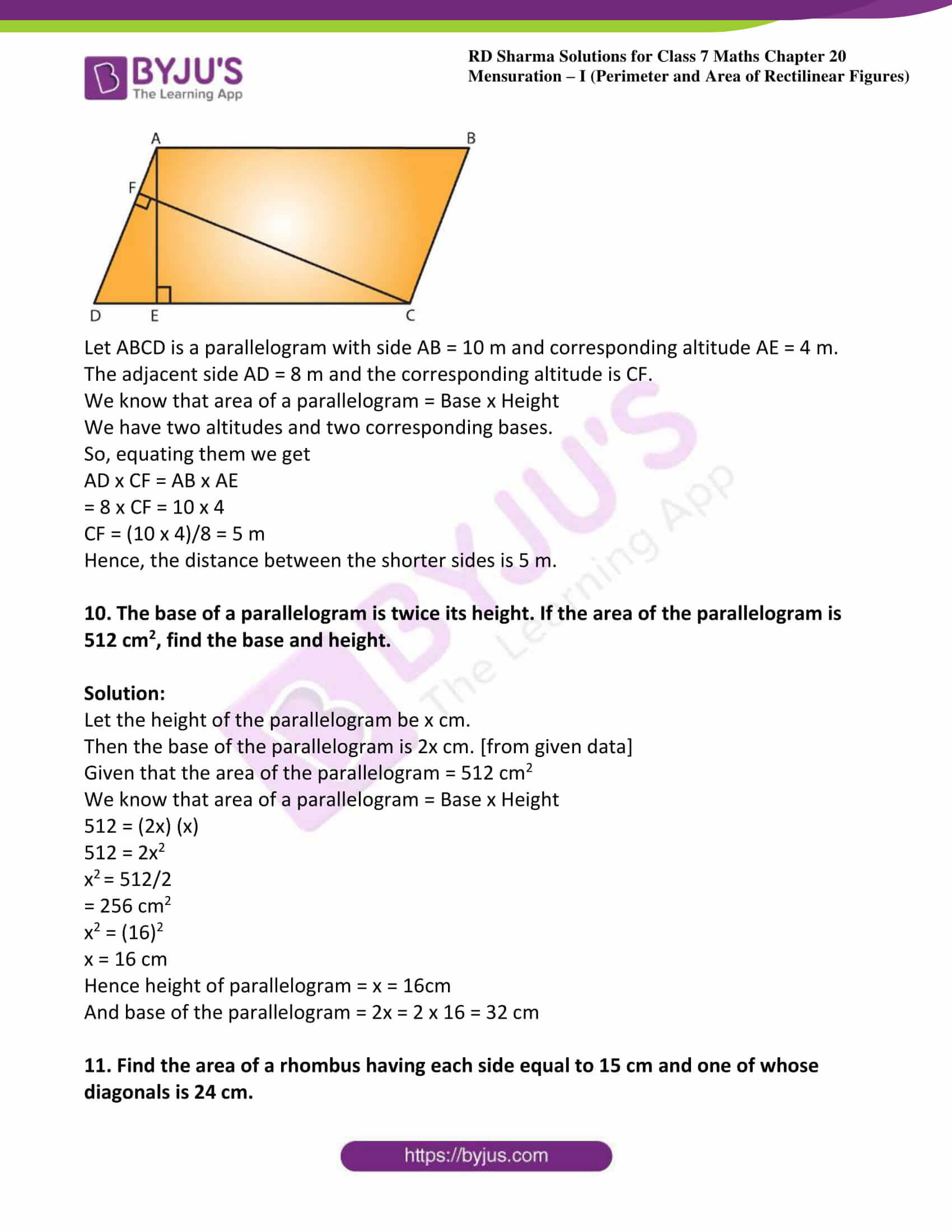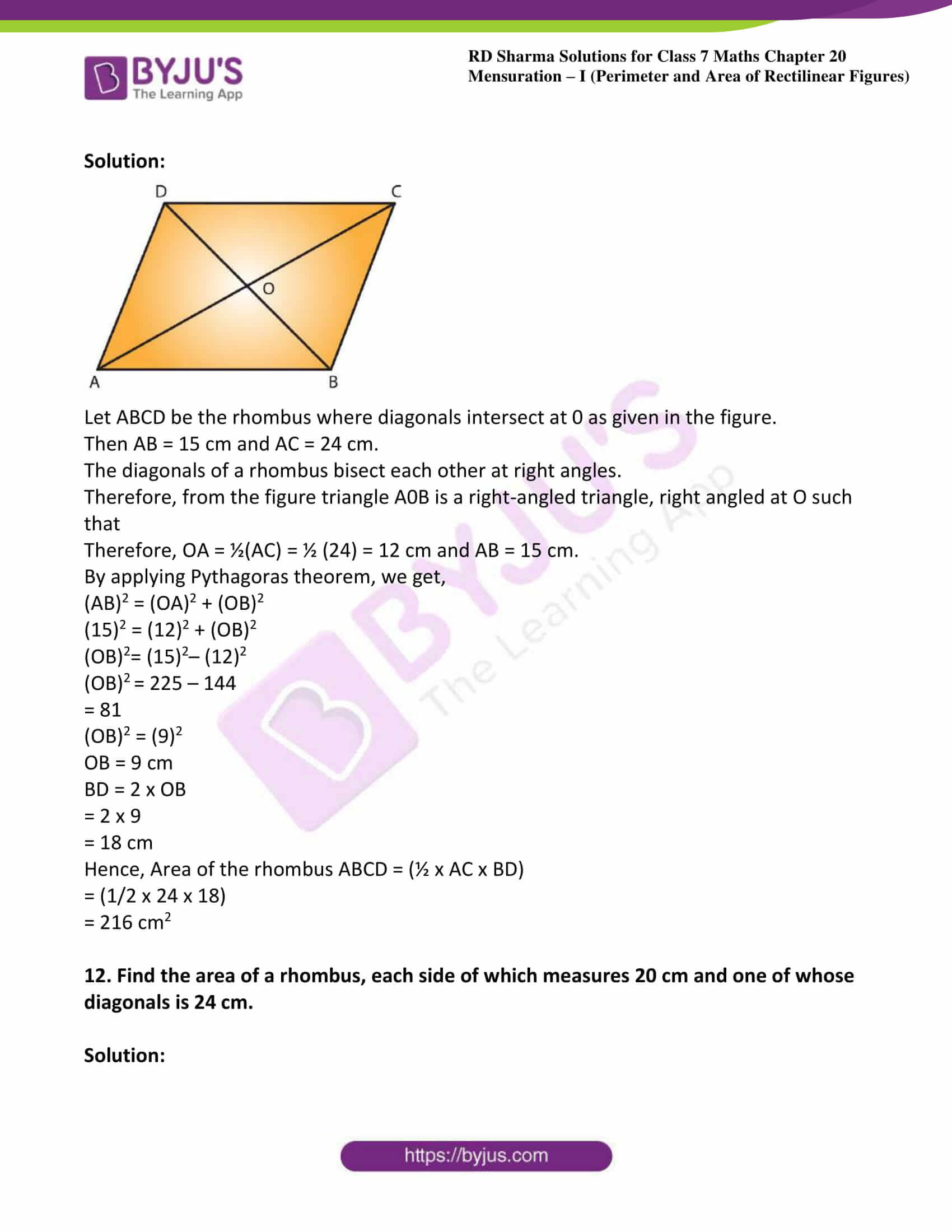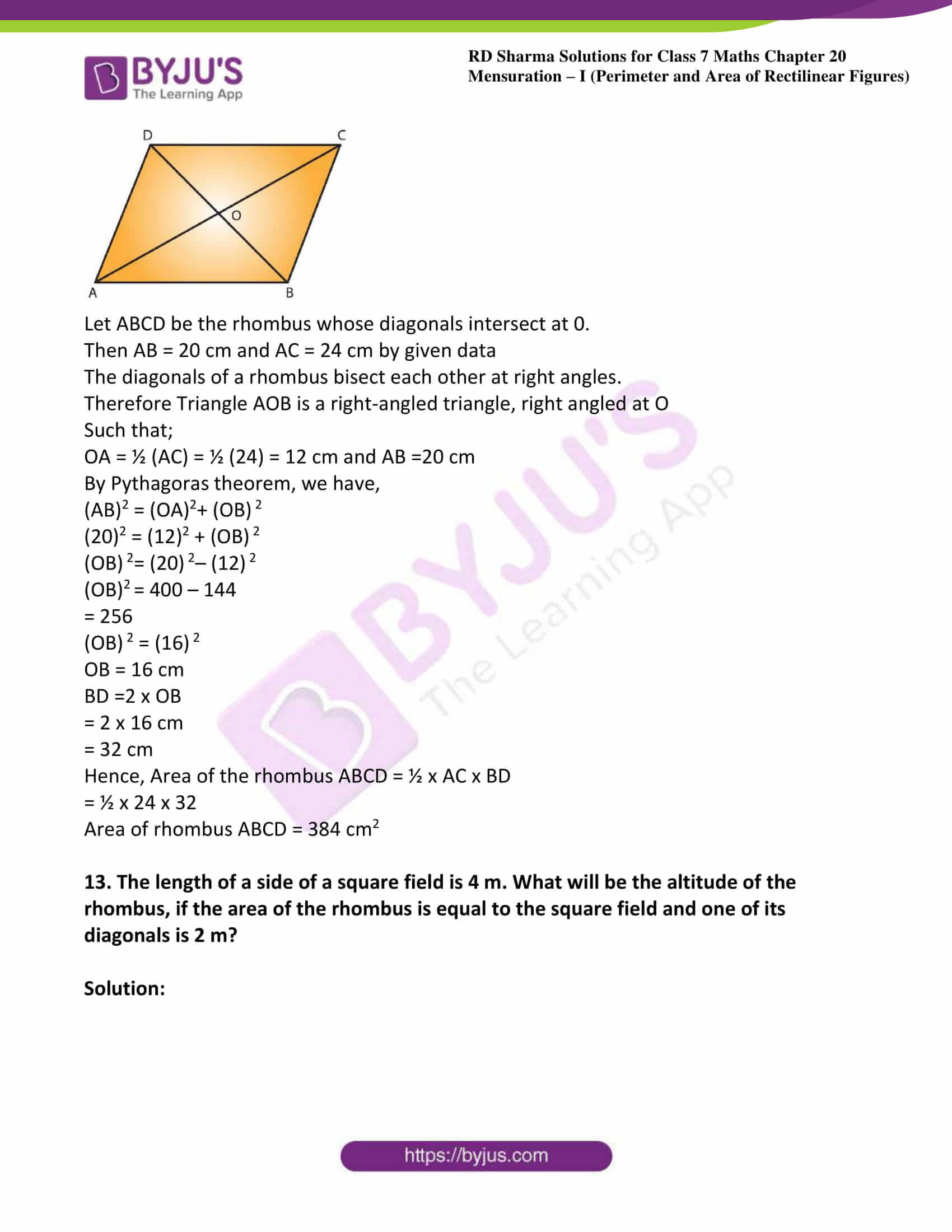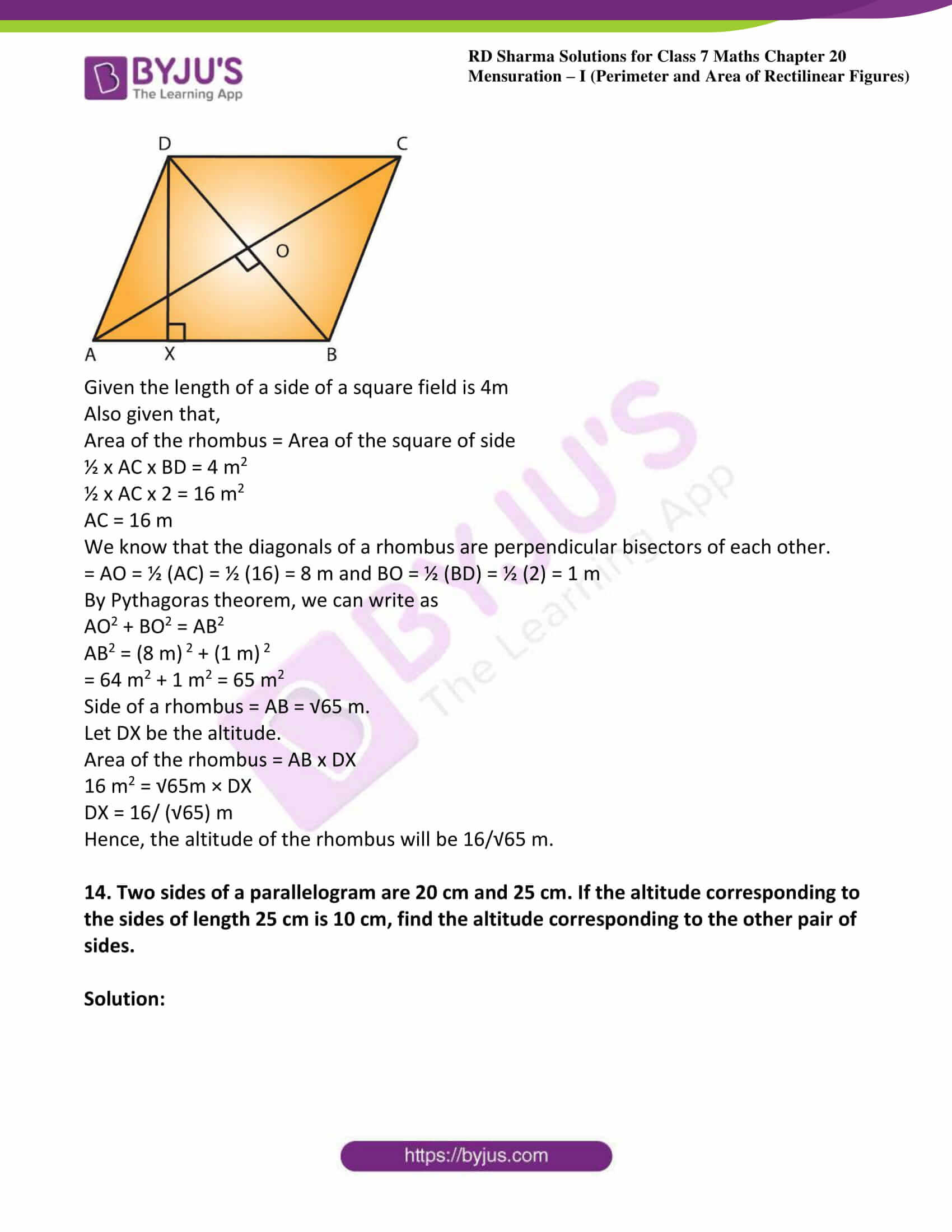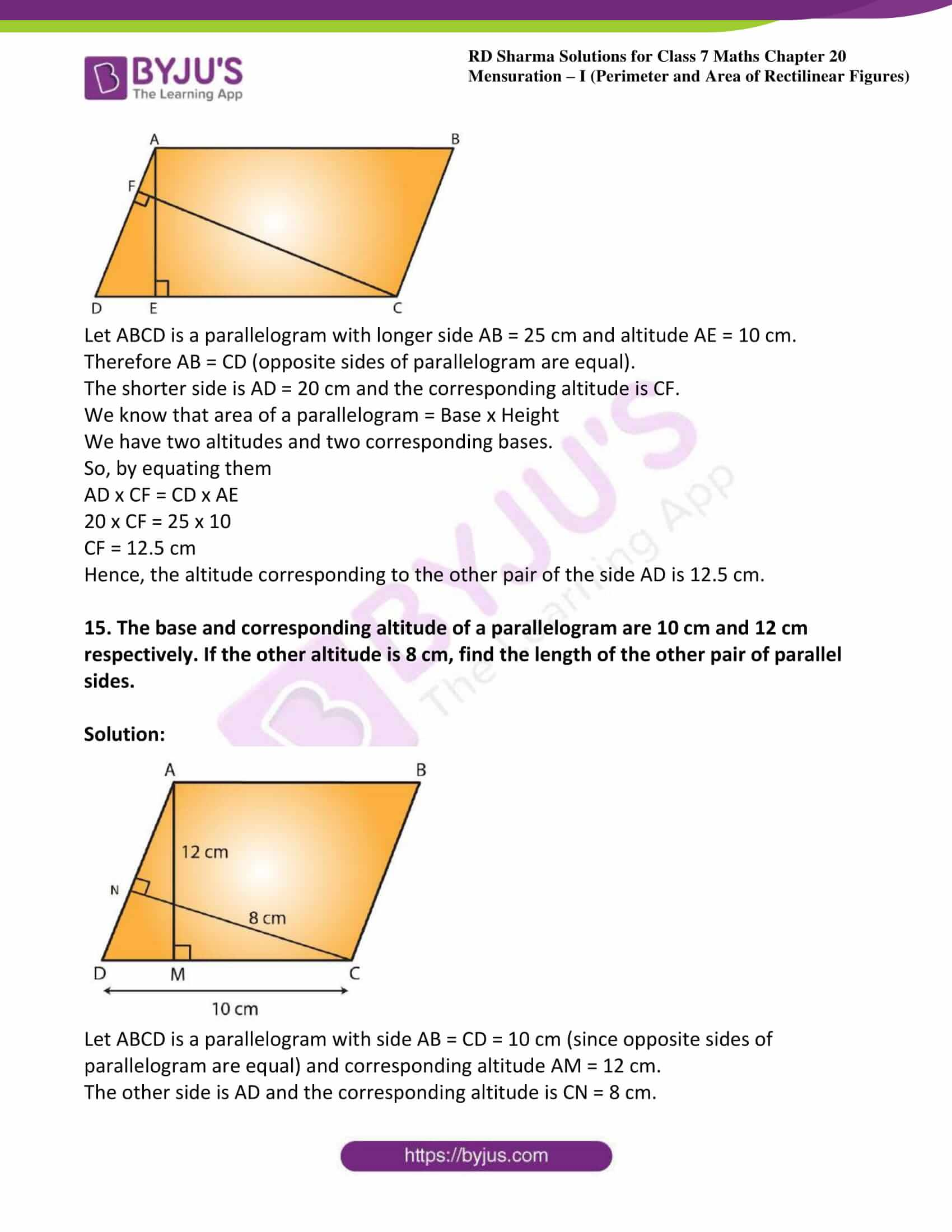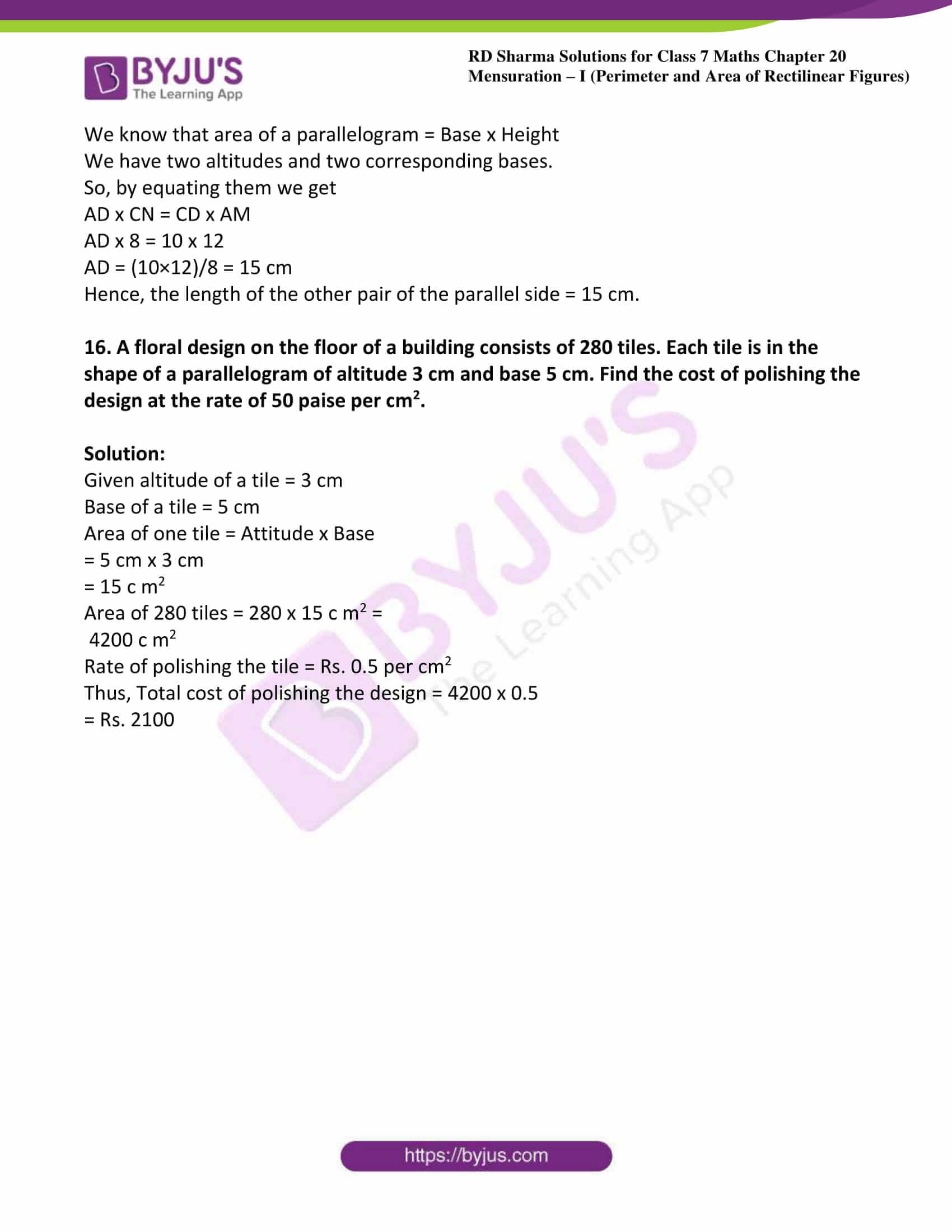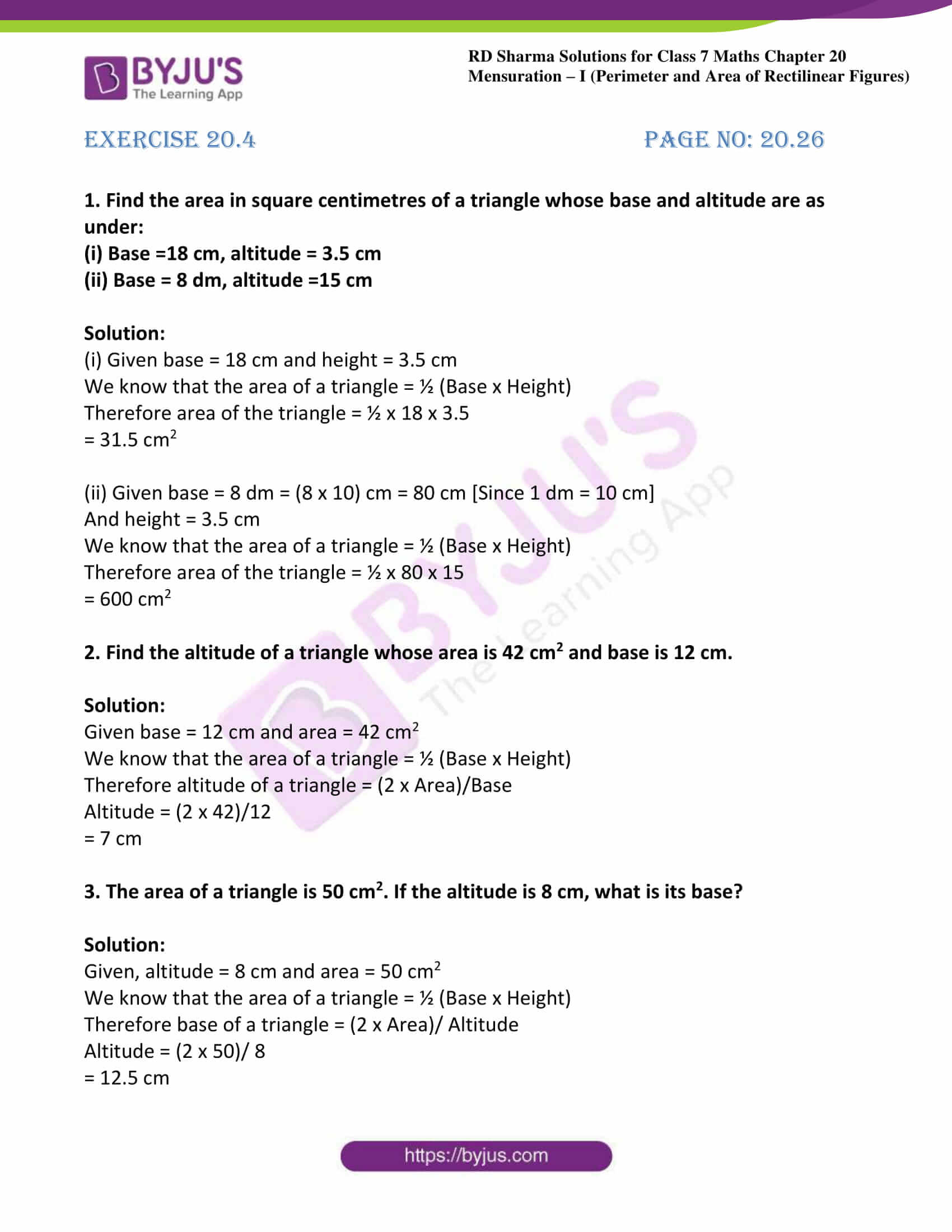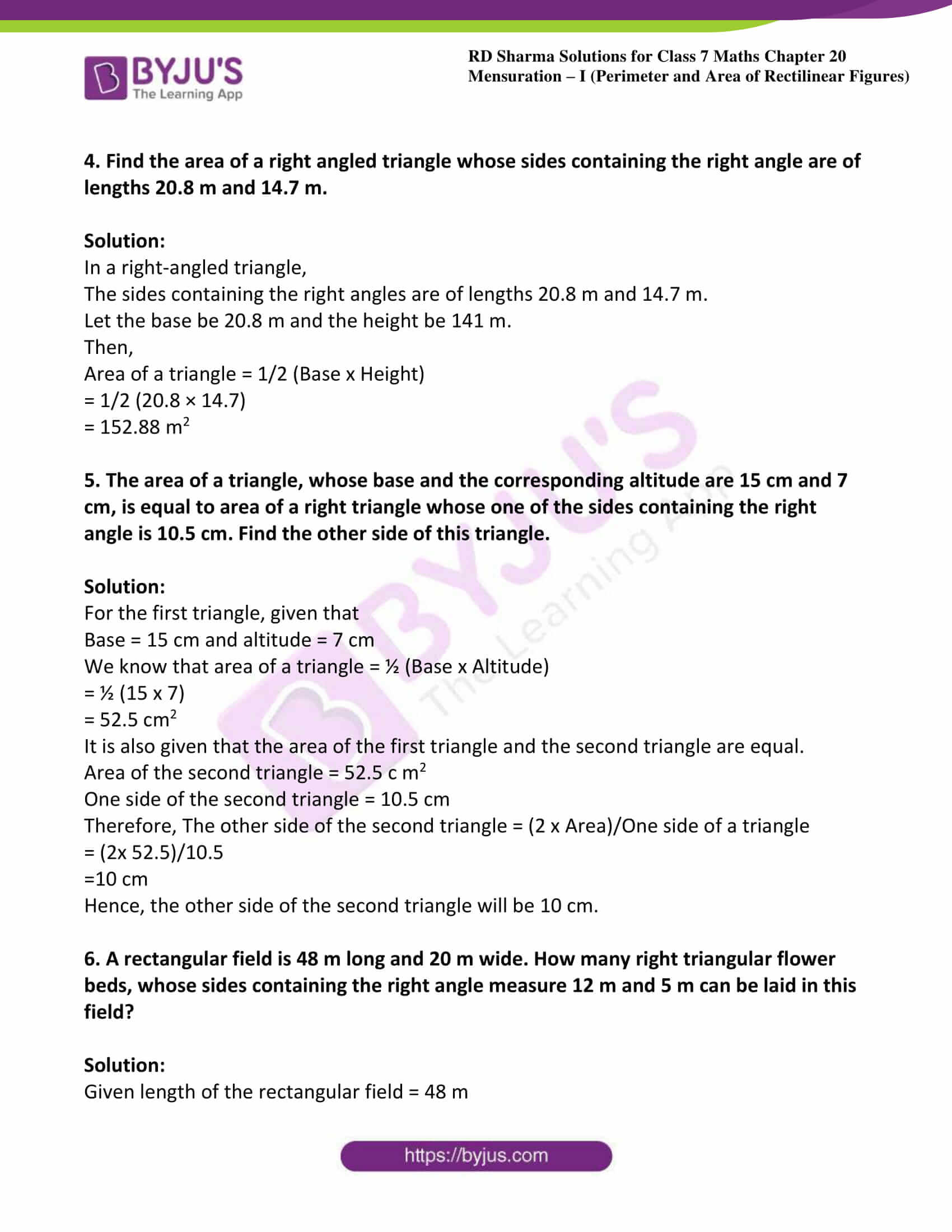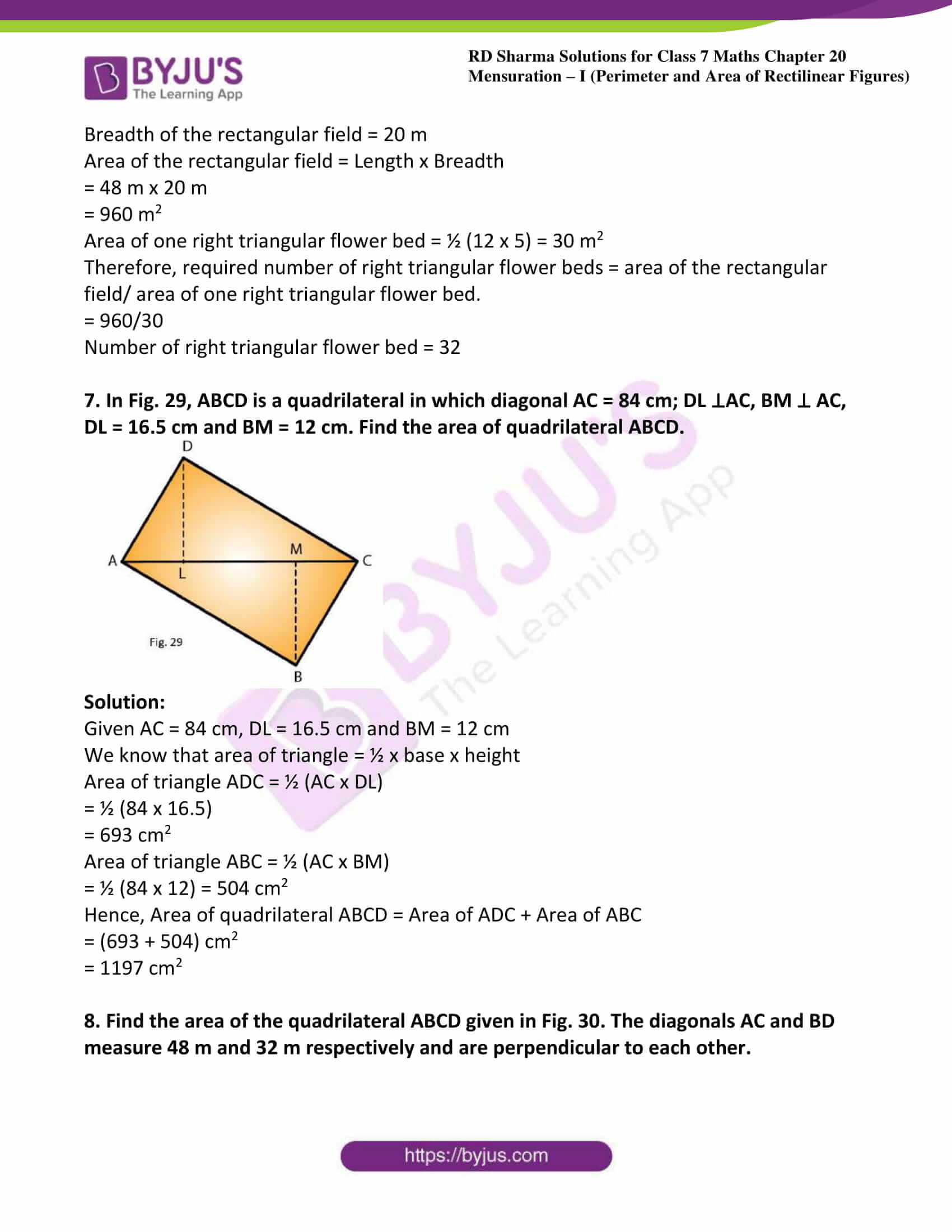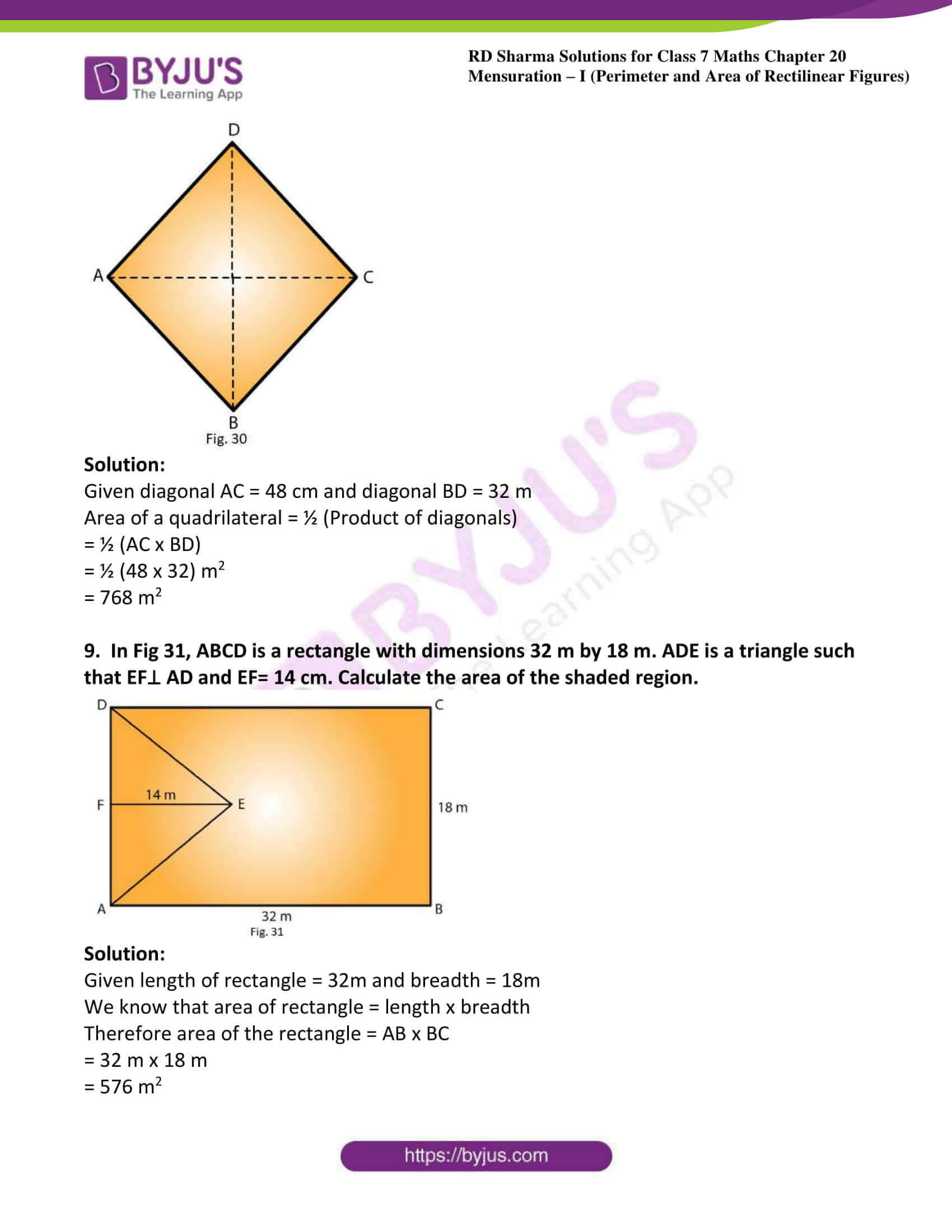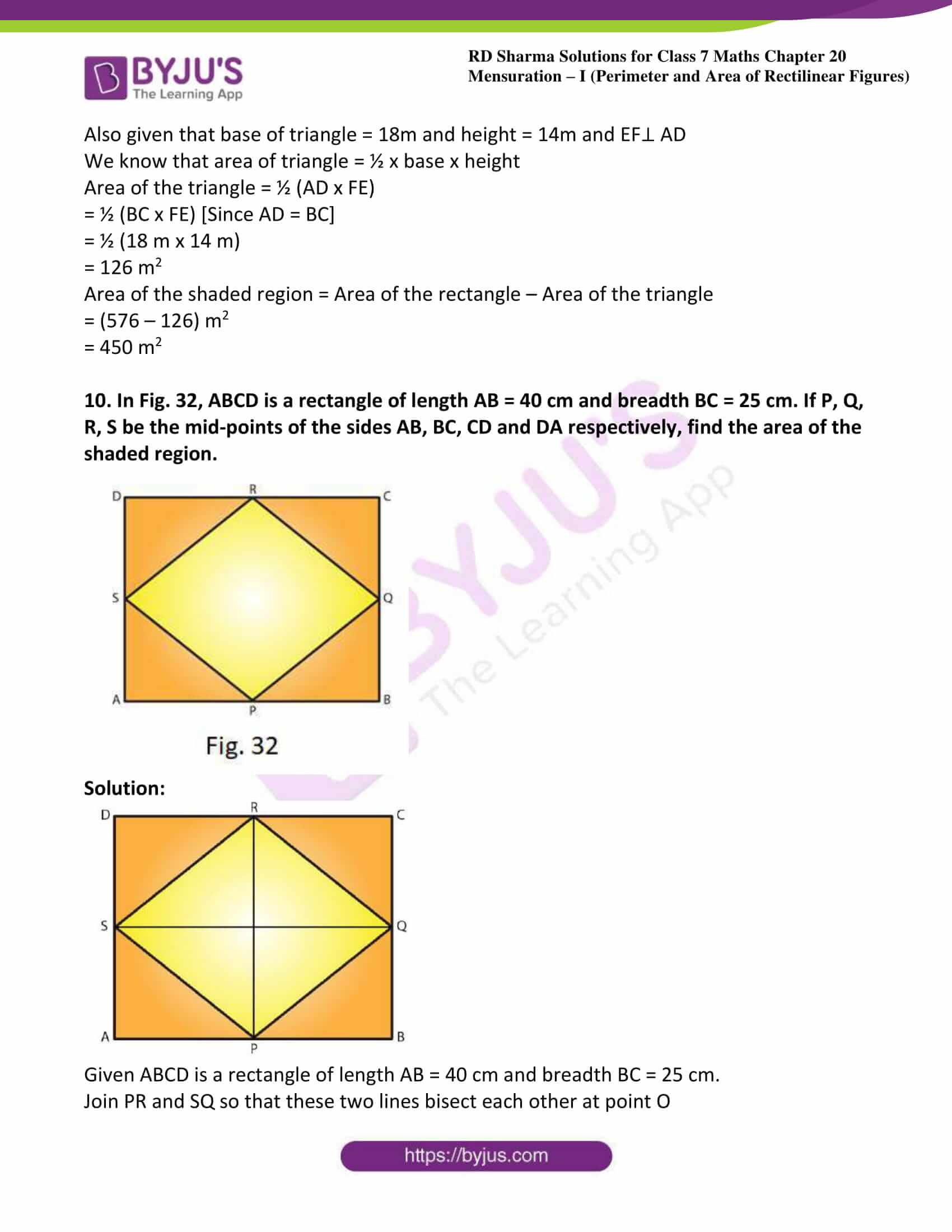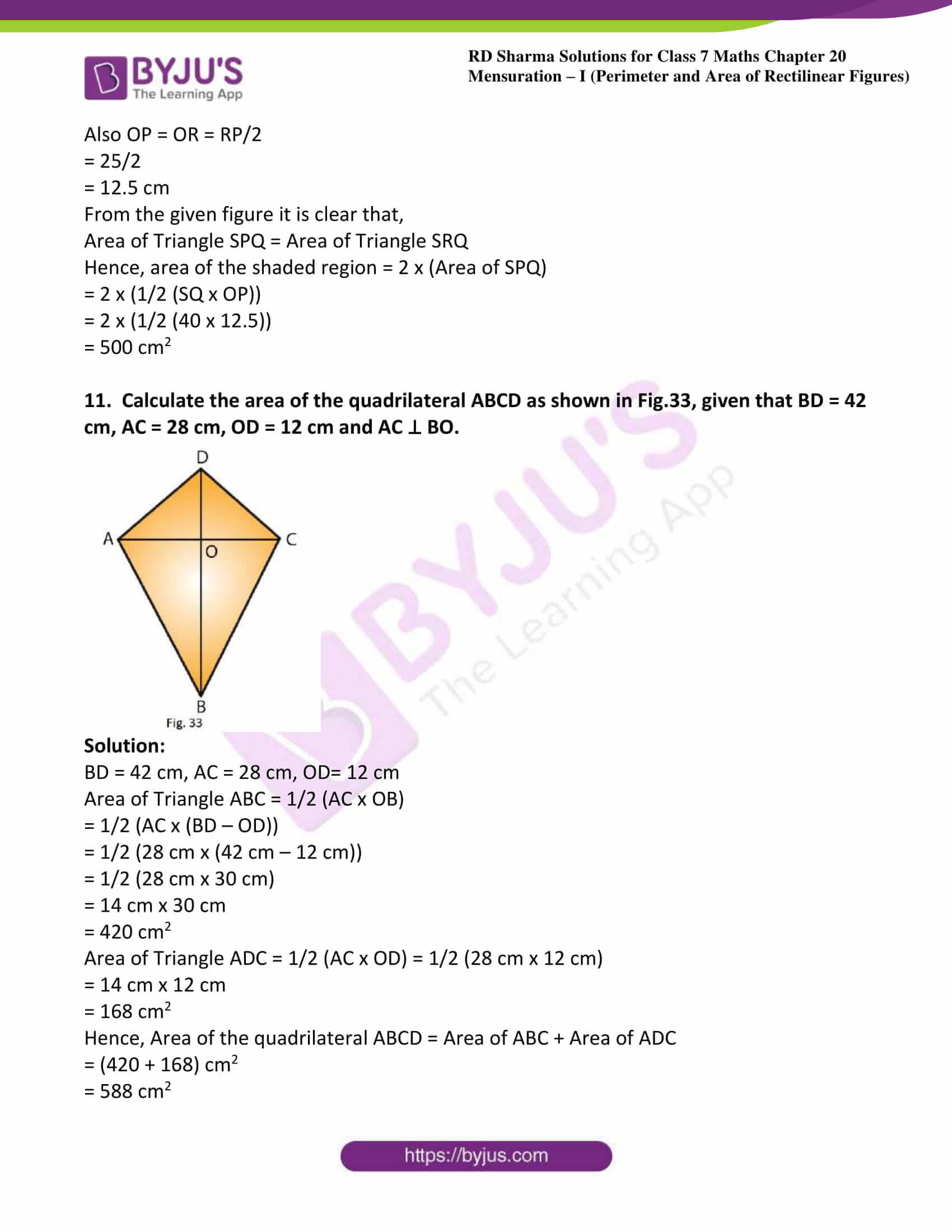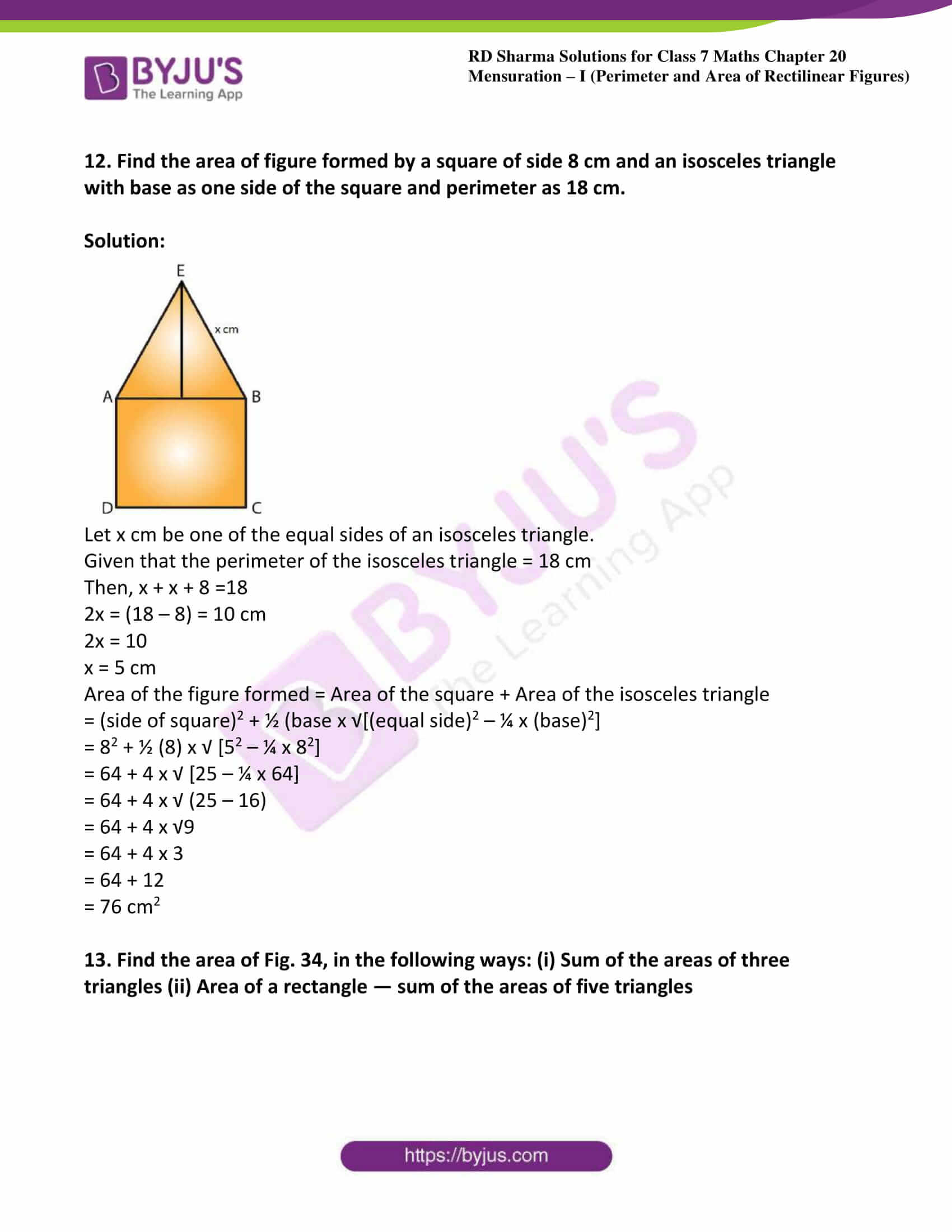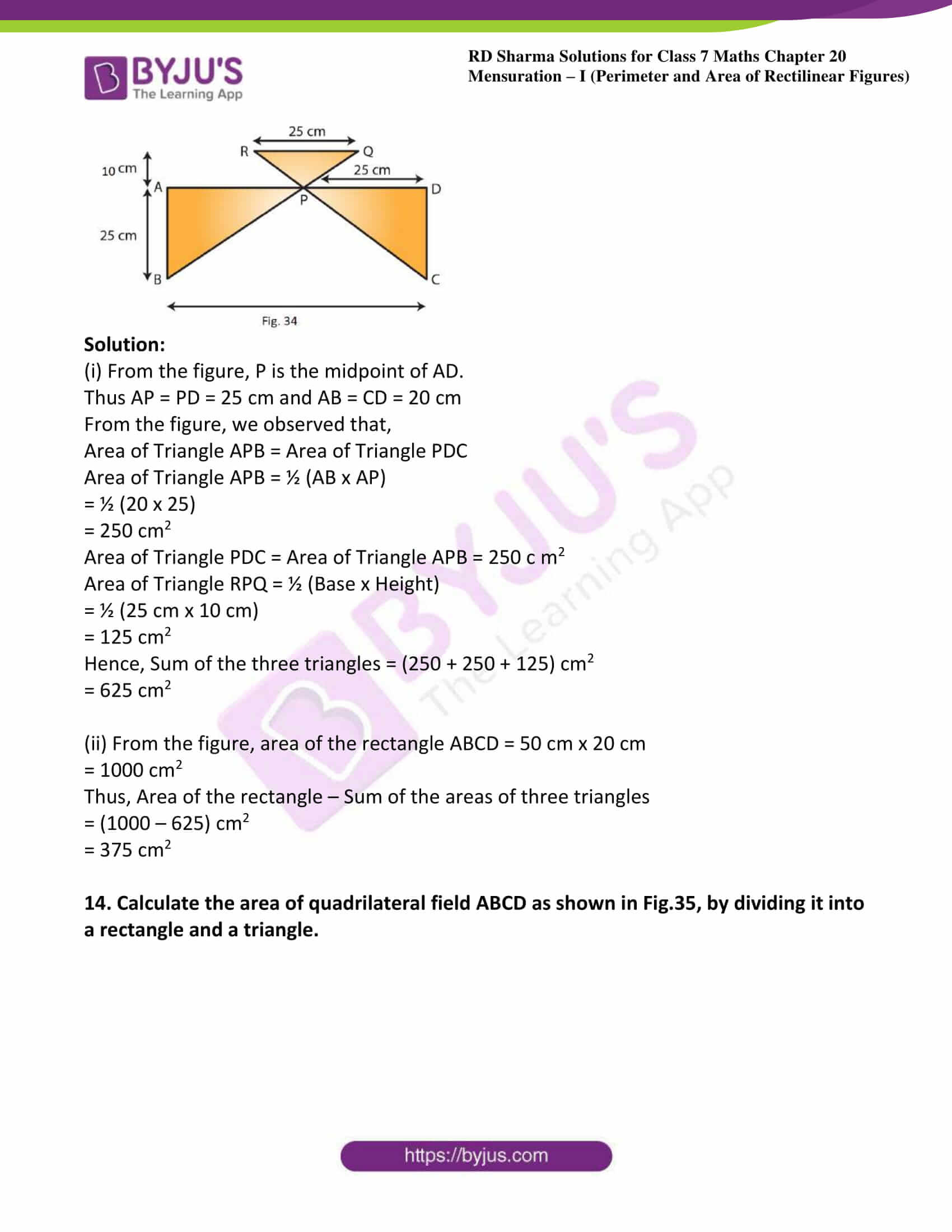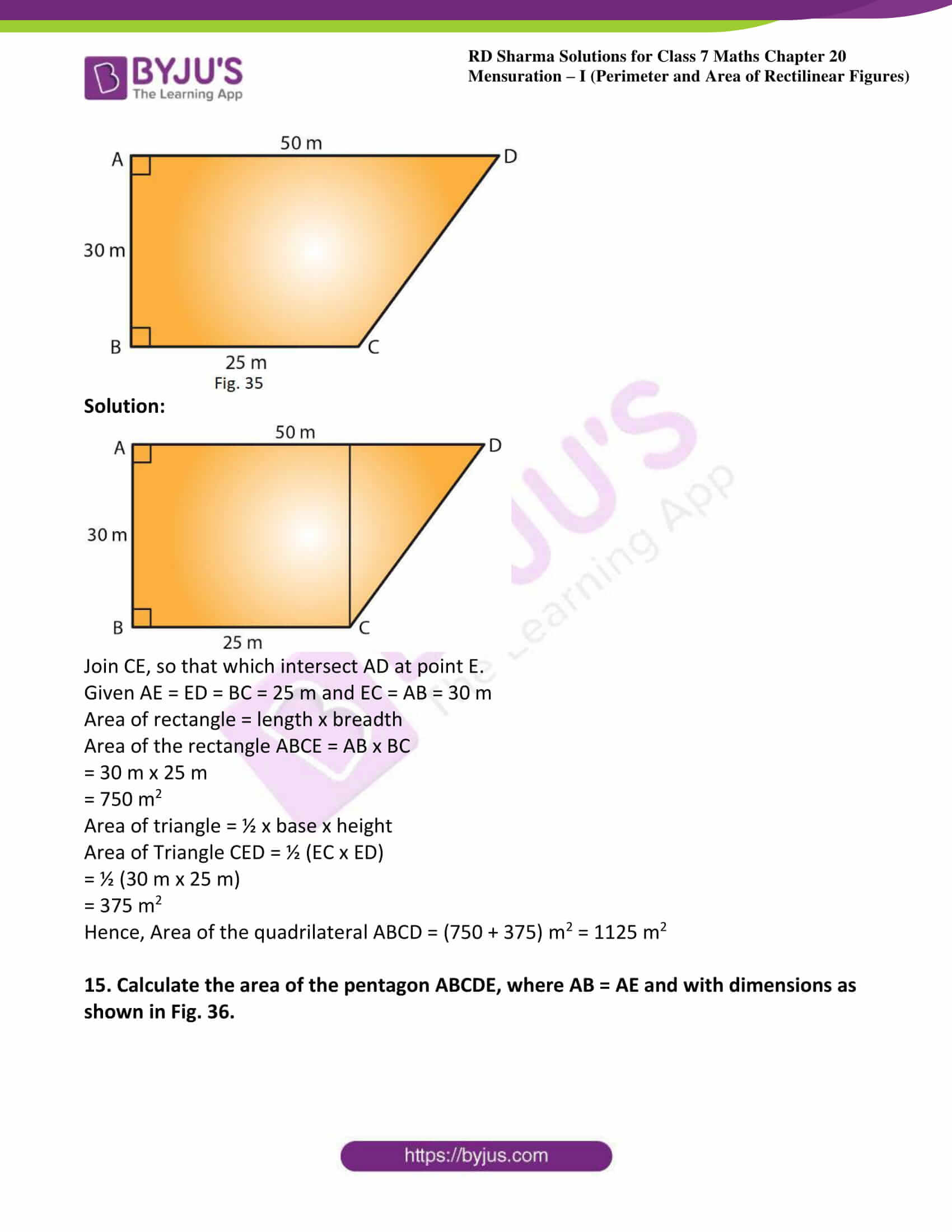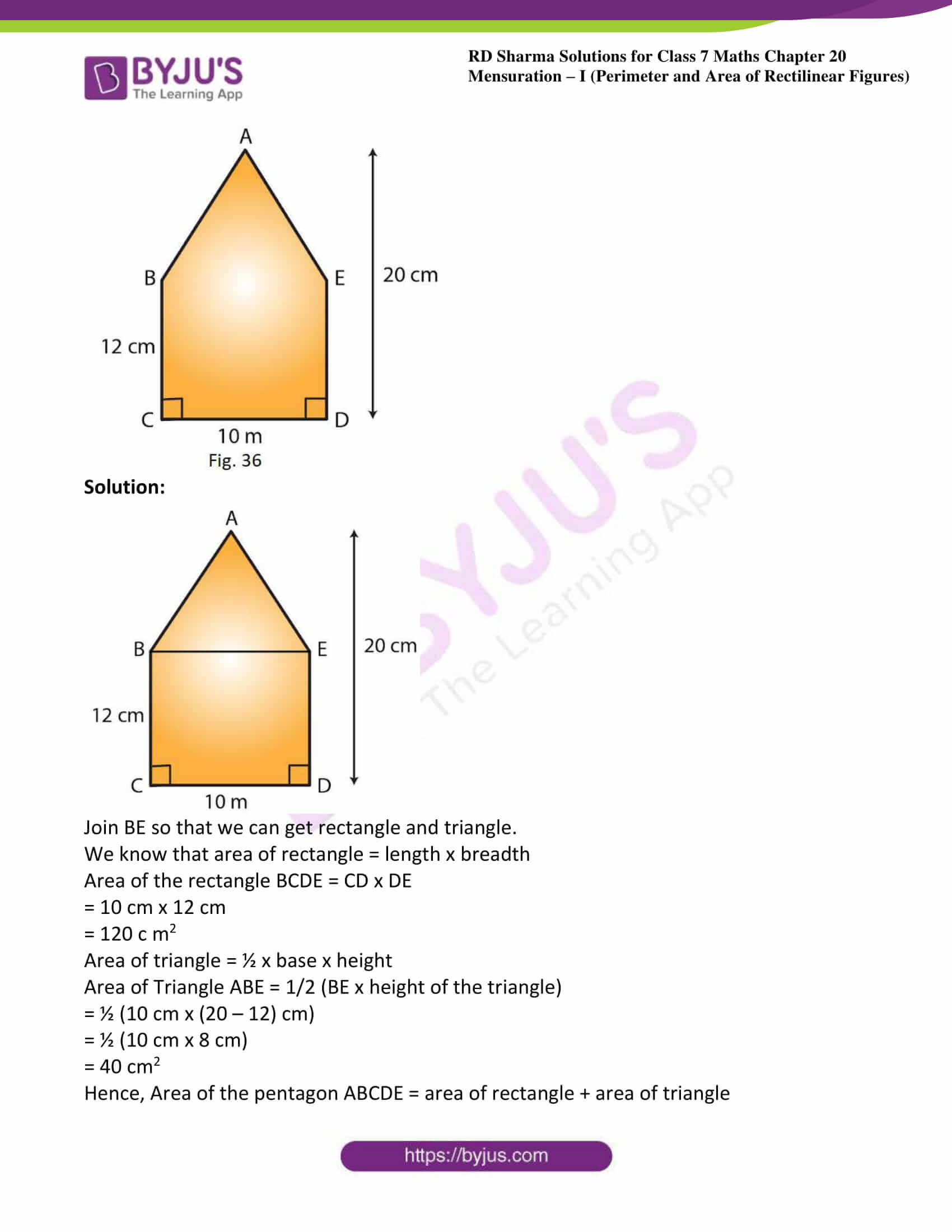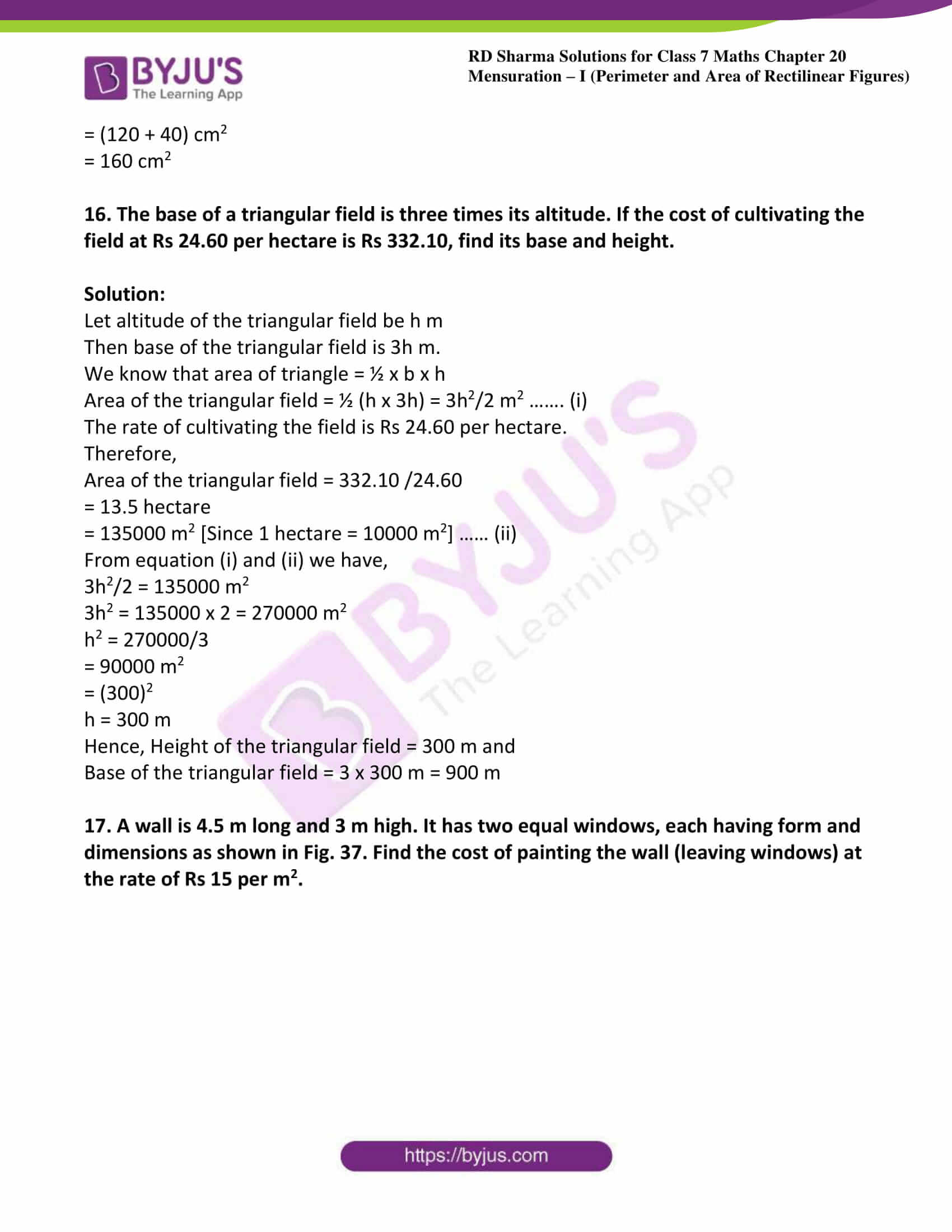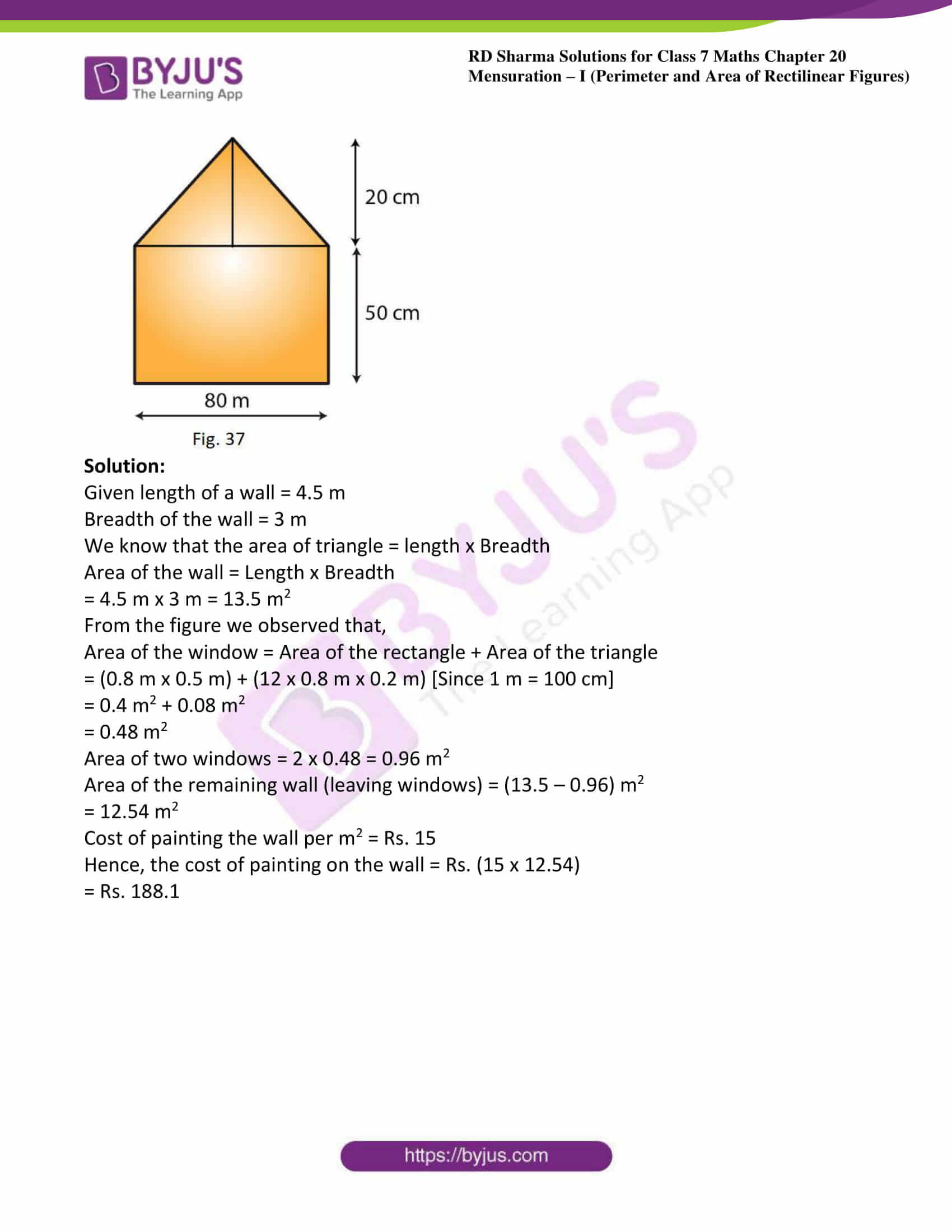### Access answers to Maths RD Sharma Solutions For Class 7 Chapter 20 – Mensuration – I (Perimeter and area of rectilinear figures)

Exercise 20.1 Page No: 20.8

1. Find the area, in square meters, of a rectangle whose

(i) Length = 5.5 m, breadth = 2.4 m

(ii) Length = 180 cm, breadth = 150 cm

Solution:

(i) Given Length = 5.5 m, Breadth = 2.4 m

We know that area of rectangle = Length x Breadth

= 5.5 m x 2.4 m = 13.2 m2

(ii) Given Length = 180 cm = 1.8 m, Breadth = 150 cm = 1.5 m [Since 100 cm = 1 m]

We know that area of rectangle = Length x Breadth

= 1.8 m x 1.5 m = 2.7 m2

2. Find the area, in square centimetres, of a square whose side is

(i) 2.6 cm

(ii) 1.2 dm

Solution:

(i) Given side of the square = 2.6 cm

We know that area of the square = (Side)2

= (2.6 cm)2

= 6.76 cm2

(ii) Given side of the square = 1.2 dm

= 1.2 x 10 cm = 12 cm [Since 1 dm = 10 cm]

We know that area of the square = (Side)2

= (12 cm)2

= 144 cm2

3. Find in square meters, the area of a square of side 16.5 dam.

Solution:

Given side of the square = 16.5

dam = 16.5 x 10 m = 165 m [Since 1 dam/dm (decametre) = 10 m]

Area of the square = (Side)2

= (165 m)2

= 27225 m2

4. Find the area of a rectangular field in acres whose sides are:

(1) 200 m and 125 m

(ii) 75 m 5 dm and 120 m

Solution:

(i) Given length of the rectangular field = 200 m

Breadth of the rectangular field = 125 m

We know that area of the rectangular field = Length x Breadth

= 200 m x 125 m

= 25000 m2

= 250 acres [Since 100 m2 = 1 acre]

(ii) Given length of the rectangular field =75 m 5 dm

= (75 + 0.5) m

= 75.5 m [Since 1 dm = 10 cm = 0.1 m]

Breadth of the rectangular field = 120 m

We know that area of the rectangular field = Length x Breadth

= 75.5 m x 120 m

= 9060 m2

= 90.6 acres [Since 100 m2 = 1 acre]

5. Find the area of a rectangular field in hectares whose sides are:

(i) 125 m and 400 m

(ii) 75 m 5 dm and 120 m

Solution:

(i) Given length of the rectangular field = 125 m

Breadth of the rectangular field = 400 m

We know that the area of the rectangular field = Length x Breadth

= 125 m x 400 m

= 50000 m2

= 5 hectares [Since 10000 m2 = 1 hectare]

(ii) Given length of the rectangular field =75 m 5 dm

= (75 + 0.5) m

= 75.5 m [Since 1 dm = 10 cm = 0.1 m]

Breadth of the rectangular field = 120 m

We know that the area of the rectangular field = Length x Breadth

= 75.5 m x 120 m

= 9060 m2

= 0.906 hectares [Since 10000 m2 = 1 hectare]

6. A door of dimensions 3 m x 2m is on the wall of dimension 10 m x 10 m. Find the cost of painting the wall if rate of painting is Rs 2.50 per sq. m.

Solution:

Given length of the door = 3 m

Also given that breadth of the door = 2 m

Side of the wall = 10 m

We know that Area of the square = (Side)2

Area of the wall = Side x Side = 10 m x 10 m

= 100 m2

We know that the area of the rectangle = length x breadth

Area of the door = Length x Breadth = 3 m x 2 m = 6 m

Thus, required area of the wall for painting = Area of the wall – Area of the door

= (100 – 6) m2

= 94 m2

Rate of painting per square metre = Rs. 2.50

Hence, the cost of painting the wall = Rs. (94 x 2.50) = Rs. 235

7. A wire is in the shape of a rectangle. Its length is 40 cm and breadth is 22 cm. If the same wire is bent in the shape of a square, what will be the measure of each side. Also, find which side encloses more area?

Solution:

Given length of rectangular shaped wire = 40cm

Breadth of rectangular shaped wire = 22cm

Perimeter of the rectangle = 2(Length + Breadth)

= 2(40 cm + 22 cm)

= 124 cm

It is given that the wire which was in the shape of a rectangle is now bent into a square.

Therefore, the perimeter of the square = Perimeter of the rectangle

Perimeter of the square = 124 cm

We know that perimeter of square = 4 x side

Therefore 4 x side = 124 cm

Side = 124/4 = 31 cm

We know that area of rectangle = length x breadth

Now, Area of the rectangle

= 40 cm x 22 cm

= 880 cm2

We know that area of the square = (Side)2

= (31 cm)2

= 961 cm2.

Therefore, the square-shaped wire encloses more area.

8. How many square meters of glass will be required for a window, which has 12 panes, each pane measuring 25 cm by 16 cm?

Solution:

Given length of the glass pane = 25 cm

Breadth of the glass pane = 16 cm

We know that area of rectangle = length x breadth

Area of one glass pane = 25 cm x 16 cm

= 400 cm2

= 0.04 m2 [Since 1 m2 = 10000 cm2]

Thus, Area of 12 panes = 12 x 0.04 = 0.48 m2

9. A marble tile measures 10 cm x 12 cm. How many tiles will be required to cover a wall of size 3 m x 4 m? Also, find the total cost of the tiles at the rate of Rs 2 per tile.

Solution:

Given area of the wall = 3 m x 4 m

= 12 m2

Also given that area of one marble tile = 10 cm x 12 cm

= 120 cm2

= 0.012 m2 [Since 1 m2 = 10000 c m2]

Number of tiles required to cover the wall = Area of wall/ Area of one marble tile

= 12/0.012

= 1000 tiles

Cost of one tile = Rs. 2

Total cost = Number of tiles x Cost of one tile

= Rs. (1000 x 2)

= Rs. 2000

10. A table top is 9 dm 5 cm long 6 dm 5 cm broad. What will be the cost to polish it at the rate of 20 paise per square centimeter?

Solution:

Given length of the table top = 9 dm 5 cm

= (9 x 10 + 5) cm

= 95 cm [Since 1 dm = 10 cm]

Also given that Breadth of the table top = 6 dm 5 cm

= (6 x 10 + 5) cm

= 65 cm

Area of the table top = Length x Breadth

= (95 cm x 65 cm)

= 6175 c m2

Rate of polishing per square centimetre = 20 paise = Rs. 0.20 [since 1Rs. = 100 paise]

Total cost to polish table top = (6175 x 0.20)

= Rs. 1235

11. A room is 9.68 m long and 6.2 m wide. Its floor is to be covered with rectangular tiles of size 22 cm by 10 cm. Find the total cost of the tiles at the rate of Rs 2.50 per tile.

Solution:

Given length of the floor of the room = 9.68 m

Breadth of the floor of the room = 6.2 m

We know that area of rectangle = length x breadth

Area of the floor = 9.68 m x 6.2 m

= 60.016 m2

Given that length of the tile = 22 cm

Breadth of the tile = 10 cm

Area of one tile = 22 cm x 10 cm

= 220 cm2

= 0.022 m2 [Since 1 m2 = 10000 c m2]

Thus, Number of tiles = 60.016 m2/0.022 m2

= 2728

We have cost of one tile = Rs. 2.50

Total cost = Number of tiles x Cost of one tile

= (2728 x 2.50)

= Rs. 6820

12. One side of a square field is 179 m. Find the cost of raising a lawn on the field at the rate of Rs 1.50 per square meter.

Solution:

Given that one side of the square field = 179 m

Area of the field = (Side) 2

= (179 m) 2

= 32041 m2

Rate of raising a lawn on the field per square metre = Rs. 1.50

Therefore total cost of raising a lawn on the field = (32041 x 1.50)

= Rs. 48061.50

13. A rectangular field is measured 290 m by 210 m. How long will it take for a girl to go two times round the field, if she walks at the rate of 1.5 m/sec?

Solution:

Given length of the rectangular field = 290 m

Also breadth of the rectangular field = 210 m

We know that perimeter of the rectangular field = 2(Length + Breadth)

= 2(290 + 210)

= 1000 m

Distance covered by the girl = 2 x Perimeter of the rectangular field

= 2 x 1000

= 2000 m

The girl walks at the rate of 1.5 m/sec.

Speed of the girl = 1.5 x 60 m/min

= 90 m/min

Speed = Distance/ Time

So, time taken by the girl = Distance/Speed

= 2000/90

=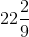min

Hence, the girl will takemin to go two times around the field.

14. A corridor of a school is 8 m long and 6 m wide. It is to be covered with canvas sheets. If the available canvas sheets have the size 2 m x 1 m, find the cost of canvas sheets required to cover the corridor at the rate of Rs 8 per sheet.

Solution:

Given length of the corridor = 8 m

Also breadth of the corridor = 6 m

Area of the corridor of a school = Length x Breadth

= (8 m x 6 m)

= 48 m2

We have length of the canvas sheet = 2 m

Breadth of the canvas sheet = 1 m

Area of one canvas sheet = Length x Breadth

= (2 m x 1 m)

= 2 m2

Thus, Number of canvas sheets required to cover the corridor = 48/2 = 24

Given Cost of one canvas sheet = Rs. 8

Total cost of the canvas sheets = number of canvas sheet x cost of one canvas sheet

= (24 x 8) = Rs. 192

15. The length and breadth of a playground are 62 m 60 cm and 25 m 40 cm respectively. Find the cost of turfing it at Rs 2.50 per square meter. How long will a man take to go three times round the field, if he walks at the rate of 2 meters per second?

Solution:

Given that length of playground = 62 m 60 cm

= 62.6 m [Since 10 cm = 0.1 m]

Breadth of a playground = 25 m 40 cm

= 25.4 m

Area of a playground = Length x Breadth

= 62.6 m x 25.4 m

= 1590.04 m2

Given rate of turfing = Rs. 2.50/ m2

Total cost of turfing = (1590.04 x 2.50)

= Rs. 3975.10

Perimeter of a rectangular field = 2(Length + Breadth)

= 2(62.6 + 25.4) = 176 m

Distance covered by the man in 3 rounds of a field = 3 x Perimeter of a rectangular field

= 3 x 176 m = 528 m

The man walks at the rate of 2 m/sec.

Speed of man walks = 2 x 60 m/min = 120 m/min

Thus, required time to cover a distance of 528 m = 528/120

= 4.4 min

= 4 minutes 24 seconds [Since 0.1 minutes = 6 seconds]

16. A lane 180 m long and 5 m wide is to be paved with bricks of length 20 cm and breadth 15 cm. Find the cost of bricks that are required, at the rate of Rs 750 per thousand.

Solution:

Given that length of lane = 180 m

Breadth of the lane = 5 m

Area of a lane = Length x Breadth

= 180 m x 5 m

= 900 m2

Length of the brick = 20 cm

Breadth of the brick = 15 cm

Area of a brick = Length x Breadth

= 20 cm x 15 cm

= 300 cm2

= 0.03 m2 [Since 1 m2 = 10000 cm2]

Required number of bricks = 900/0.03 = 30000

Cost of 1000 bricks = Rs. 750

Total cost of 30,000 bricks = 750×30,000/1000

= Rs. 22,500

17. How many envelopes can be made out of a sheet of paper 125 cm by 85 cm; supposing one envelope requires a piece of paper of size 17 cm by 5 cm?

Solution:

Given length of the sheet of paper = 125 cm

Also given that breadth of the sheet of paper = 85 cm

Area of a sheet of paper = Length x Breadth

= 125 cm x 85 cm

= 10,625 cm2

Length of sheet required for an envelope = 17 cm

Breadth of sheet required for an envelope = 5 cm

Area of the sheet required for one envelope = Length x Breadth

= 17 cm x 5 cm

= 85 c m2

Thus, required number of envelopes = 10,625/85

= 125

18. The width of a cloth is 170 cm. Calculate the length of the cloth required to make 25 diapers, if each diaper requires a piece of cloth of size 50 cm by 17 cm.

Solution:

Given that length of the diaper = 50 cm

Breadth of the diaper = 17 cm

Area of cloth to make 1 diaper = Length x Breadth

= 50 cm x 17 cm

= 850 cm2

Thus, Area of 25 such diapers = (25 x 850) cm2

= 21,250 cm2

Area of total cloth = Area of 25 diapers = 21,250 cm2

It is given that width of a cloth = 170 cm

Length of the cloth = Area of cloth

We have width of cloth = 170cm

Length of a cloth = 21,250/170 cm = 125 cm

Hence, length of the cloth will be 125 cm.

19. The carpet for a room 6.6 m by 5.6 m costs Rs 3960 and it was made from a roll 70 cm wide. Find the cost of the carpet per meter.

Solution:

Given that length of a room = 6.6 m

Breadth of a room = 5.6 m

Area of a room = Length x Breadth

= 6.6 m x 5.6 m

= 36.96 m2

Width of a carpet = 70 cm = 0.7 m [Since 1 m = 100 cm]

Length of a carpet = Area of a room

36.96 = Length of the carpet × 0.7

Length of the carpet = 36.96/0.7

Length of the carpet = 52.8 m

Cost of the carpet per square = Total cost of the carpet/Length of the room

= 3960/52.8

= Rs. 75

20. A room is 9 m long, 8 m broad and 6.5 m high. It has one door of dimensions 2 m x 1.5 m and three windows each of dimensions 1.5 m x 1 m. Find the cost of white washing the walls at Rs 3.80 per square meter.

Solution:

Given Length of a room = 9 m

Breadth of a room = 8 m

Height of a room = 6.5 m

Area of 4 walls = 2(1 + b) h

= 2 (9 m + 8 m) x 6.5 m

= 2 x 17 m x 6.5 m

= 221 m2

Length of a door = 2 m

Breadth of a door = 1.5 m

Area of a door = Length x Breadth

= 2 m x 1.5 m

= 3 m2

Length of a window = 1.5 m

Breadth of a window = 1 m

Since, area of one window = Length x Breadth

= 1.5 m x 1 m

= 1.5 m2

Thus, Area of 3 such windows = 3 x 1.5 m2

= 4.5 m2

Area to be white washed = Area of 4 walls – (Area of one door + Area of 3 windows)

Area to be white washed = [221 – (3 + 4.5)] m2

= (221 – 7.5) m2

= 213.5 m2

We have cost of white washing for 1 m2 area = Rs. 3.80

Cost of white washing for 213.5 m2 area = Rs. (213.5 x 3.80)

= Rs. 811.30

21. A hall 36 m long and 24 m broad allowing 80 m2 for doors and windows, the cost of papering the walls at Rs 8.40 per m2 is Rs 9408. Find the height of the hall.

Solution:

Given length of the hall = 36 m

Breadth of the hall = 24 m

Let h be the height of the hall.

Now, in papering the wall, we need to paper the four walls excluding the floor and roof of the hall. So, the area of the wall which is to be papered = Area of 4 walls

= 2 h (I + b)

= 2 h (36 + 24)

= 120 hm2

Now, area left for the door and the windows = 80 m2

So, the area which is actually papered = (120h – 80) m2

Cost of papering the wall = Area required for papering × Cost per meter square of papering wall

9408 = (120 h – 80) × 8.40

1120 = 120 h – 80

1200 = 120 h

h = 10 m

Hence, the height of the wall would be 10 m.

Exercise 20.2 Page No: 20.13

1. A rectangular grassy lawn measuring 40 m by 25 m is to be surrounded externally by a path which is 2 m wide. Calculate the cost of leveling the path at the rate of Rs 8.25 per square meter.

Solution: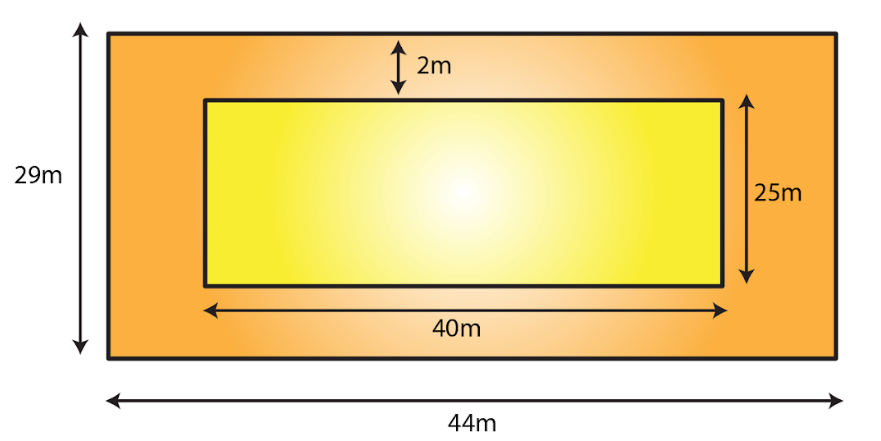Let PQRS be the rectangular grassy lawn and let length = AB and breadth = BC

Given Length AB = 40 m and breadth BC = 25 m

Area of lawn PQRS = 40 m x 25 m = 1000 m2

Length AB = (40 + 2 + 2) m = 44 m

Breadth BC = (25 + 2 + 2) m = 29 m

Area of ABCD = 44 m x 29 m = 1276 m2

Now, Area of the path = Area of ABCD – Area of the lawn PQRS

= 1276 m2 – 1000 m2

= 276 m2

Rate of levelling the path = Rs. 8.25 per m2

Cost of levelling the path = (8.25 x 276)

= Rs. 2277

2. One meter wide path is built inside a square park of side 30 m along its sides. The remaining part of the park is covered by grass. If the total cost of covering by grass is Rs 1176, find the rate per square meter at which the park is covered by the grass.

Solution: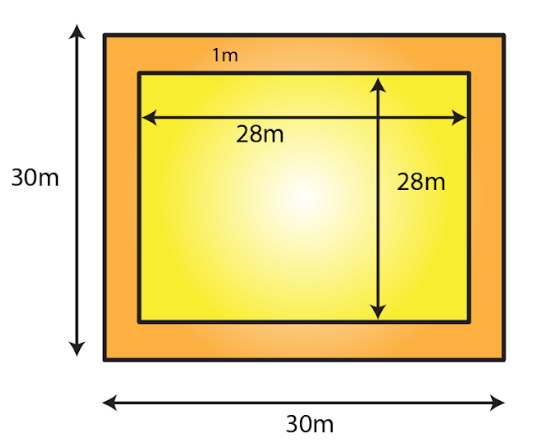Given that side of a square garden = 30m = a

We know that area of square = a2

Area of the square garden including the path = a2

= (30)2 = 900 m2

From the figure, it can be observed that the side of the square garden, when the path is not included, is 28 m.

Area of the square garden not including the path = (28) 2

= 784 m2

Total cost of covering the park with grass = Area of the park covering with green grass x Rate per square metre

1176 = 784 x Rate per square metre

Rate per square metre at which the park is covered with grass = (1176/784)

= Rs. 1.50 per m2

3. Through a rectangular field of sides 90 m x 60 m, two roads are constructed which are parallel to the sides and cut each other at right angles through the center of the field. If the width of the roads is 3 m, find the total area covered by the two roads.

Solution: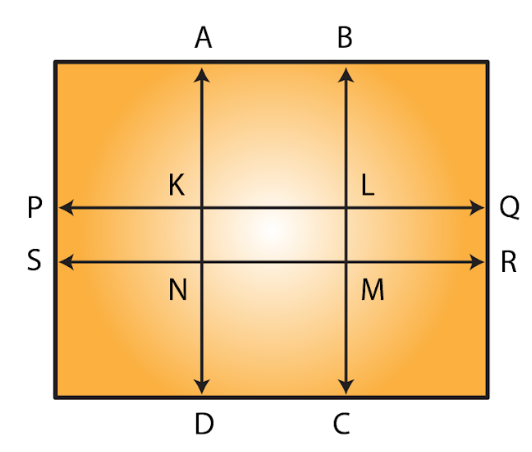Given length of rectangular field = 90m

Breadth of rectangular field = 60m

Area of the rectangular field = 90 m x 60 m = 5400 m2

Area of the road PQRS = 90 m x 3 m

= 270 m2

Area of the road ABCD = 60 m x 3 m

= 180 m2

Clearly, area of KLMN is common to the two roads.

Thus, area of KLMN = 3 m x 3 m

= 9 m2

Hence, area of the roads = Area (PQRS) + Area (ABCD) – Area (KLMN)

= (270 + 180) m2 – 9 m2

= 441 m2

4. From a rectangular sheet of tin, of size 100 cm by 80 cm, are cut four squares of side 10 cm from each corner. Find the area of the remaining sheet.

Solution: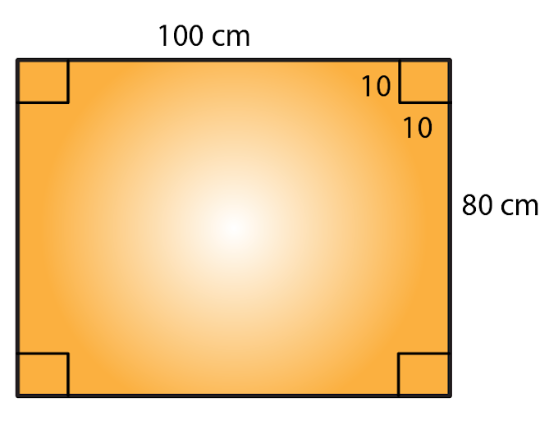Given that length of rectangular sheet = 100 cm

Breadth of rectangular sheet = 80 cm

Area of the rectangular sheet of tin = 100 cm x 80 cm

= 8000 c m2

Side of the square at the corner of the sheet = 10 cm

Area of one square at the corner of the sheet = (10 cm)2

= 100 cm2

Area of 4 squares at the corner of the sheet = 4 x 100 cm2

= 400 cm2

Hence, Area of the remaining sheet of tin = Area of the rectangular sheet – Area of the 4 squares

Area of the remaining sheet of tin = (8000 – 400) cm2

= 7600 cm2

5. A painting 8 cm long and 5 cm wide is painted on a cardboard such that there is a margin of 1.5 cm along each of its sides. Find the total area of the margin.

Solution: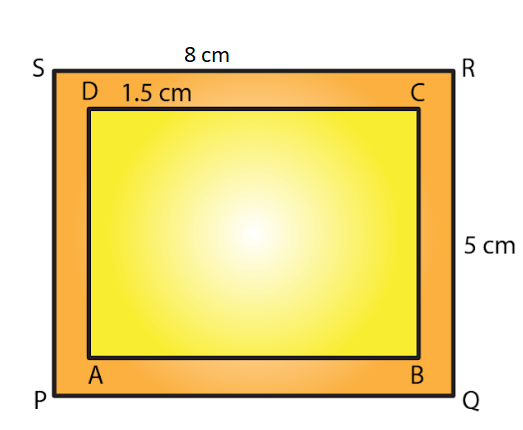Given length of cardboard including margin = 8 cm

Breadth of the cardboard including margin = 5 cm

Area of the cardboard including the margin = 8 cm x 5 cm = 40 c m2

From the figure, we can observed that,

New length of the painting without margin = 8 cm – (1.5 cm + 1.5 cm)

= (8 – 3) cm

= 5 cm

New breadth of the painting without margin = 5 cm – (1.5 cm + 1.5 cm)

= (5 – 3) cm

= 2 cm

Area of the painting not including the margin = 5 cm x 2 cm = 10 cm2

Hence, Area of the margin = Area of the cardboard including the margin – Area of the painting

= (40 – 10) cm2

= 30 cm2

6. Rakesh has a rectangular field of length 80 m and breadth 60 m. In it, he wants to make a garden 10 m long and 4 m broad at one of the corners and at another corner, he wants to grow flowers in two floor-beds each of size 4 m by 1.5 m. In the remaining part of the field, he wants to apply manures. Find the cost of applying the manures at the rate of Rs 300 per area.

Solution: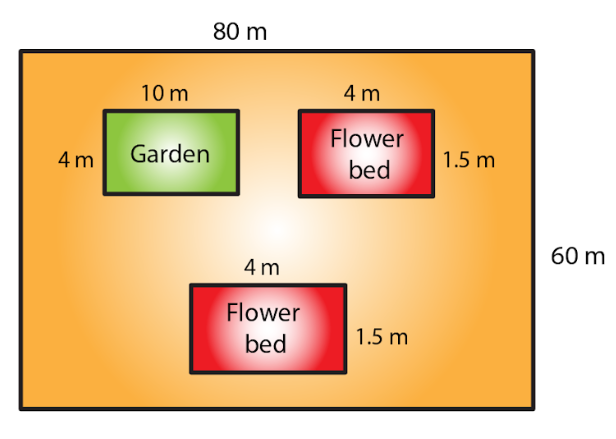Given that length of a rectangular field = 80 m

Breadth of a rectangular field = 60 m

Area of rectangular field = length x breadth

= (80 x 60) m

= 4800 m2

Again, Area of the garden = 10 m x 4 m = 40 m2

Area of one flower bed = 4 m x 1.5 m = 6 m2

Thus, Area of two flower beds = 2 x 6 m2 = 12 m2

Remaining area of the field for applying manure = Area of the rectangular field – (Area of the garden + Area of the two flower beds)

Remaining area of the field for applying manure = 4800 m2 – (40 + 12) m2

= (4800 – 52) m2

= 4748 m2

Since 100 m2 = 1 acre

Therefore by using the above

4748 m2 = 47.48 acres

So, cost of applying manure at the rate of Rs. 300 per are will be = Rs. (300 x 47.48)

= Rs. 14244

7. Each side of a square flower bed is 2 m 80 cm long. It is extended by digging a strip 30 cm wide all around it. Find the area of the enlarged flower bed and also the increase in the area of the flower bed.

Solution: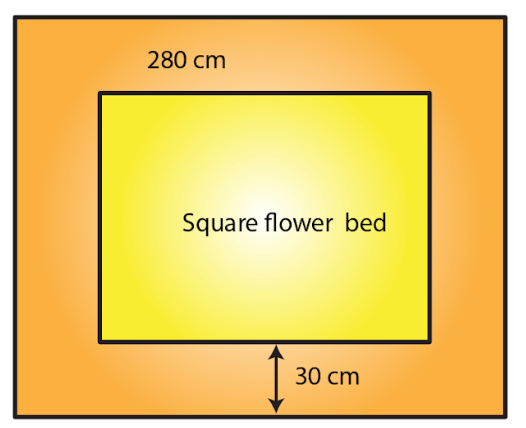Given side of the flower bed = 2 m 80 cm = 2.80 m [since 100cm = 1m]

Area of the square flower bed = (Side)2

= (2.80 m)2

= 7.84 m2

Side of the flower bed with strip = 2.80 m + 30 cm + 30 cm

= (2.80 + 0.3 + 0.3) m

= 3.4 m

Area of the enlarged flower bed with the digging strip = (Side)2

= (3.4)2

= 11.56 m2

Thus, Increase in the area of the flower bed = Area of the enlarged flower bed with the digging strip – Area of the square flower bed

= 11.56 m2 – 7.84 m2

= 3.72 m2

8. A room 5 m long and 4 m wide is surrounded by a verandah. If the verandah occupies an area of 22 m2, find the width of the verandah.

Solution: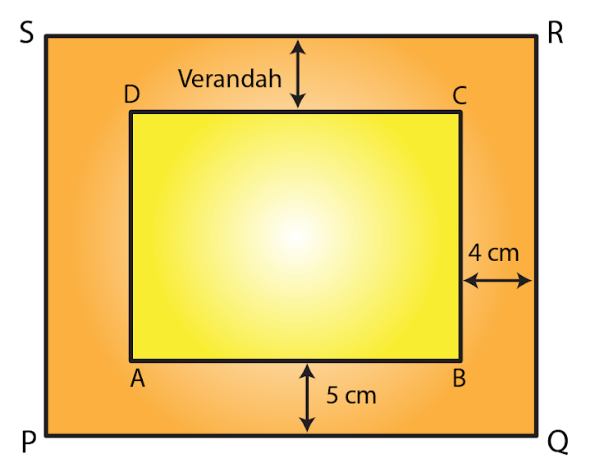Let the width of the verandah be x m.

Given Length of the room AB = 5 m and breadth of the room, BC = 4 m

We know that area of rectangle = length x breadth

Area of the room = 5 m x 4 m

= 20 m2

From the figure, it is clear that

Length of the veranda PQ = (5 + x + x) = (5 + 2x) m

Breadth of the veranda QR = (4 + x + x) = (4 + 2x) m

Area of veranda PQRS = (5 + 2x) x (4 + 2x)

= (4×2 + 18x + 20) m2

Area of veranda = Area of PQRS – Area of ABCD

22 = 4x2 + 18x + 20 – 20

22 = 4x2 + 18x

On dividing above equation by 2 we get,

11 = 2x2 + 9x

2x2 + 9x – 11 = 0

2x2 + 11x – 2x – 11 = 0

x (2x+11) – 1 (2x+11) = 0

(x- 1) (2x+11)= 0

When x – 1 = 0, x = 1

When 2x + 11 = 0, x = (-11/2)

The width cannot be a negative value. So, width of the veranda = x = 1 m

9. A square lawn has a 2 m wide path surrounding it. If the area of the path is 136 m2, find the area of the lawn.

Solution: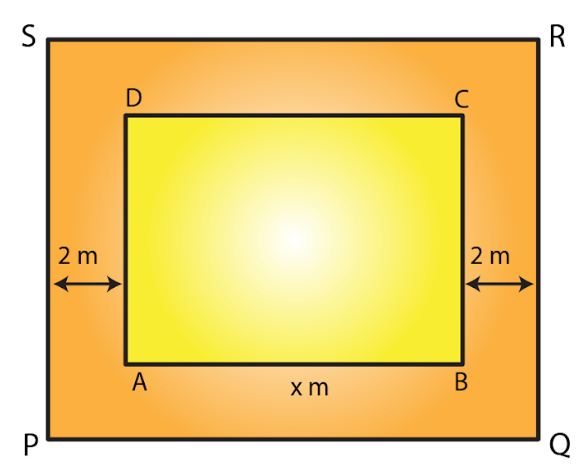Let ABCD be the square lawn and PQRS be the outer boundary of the square path.

Let side of the lawn AB be x m.

Area of the square lawn = x2

Given that Length PQ = (x + 2 + 2) = (x + 4) m

Area of PQRS = (x + 4)2 = (x2 + 8x + 16) m2

Now, Area of the path = Area of PQRS – Area of the square lawn

136 = x2 + 8x + 16 – x2

136 = 8x + 16

136 – 16 = 8x

120 = 8x

x = 120/ 8 = 15

Side of the lawn = 15 m

Hence, Area of the lawn = (Side)2

= (15 m)2

= 225 m2

10. A poster of size 10 cm by 8 cm is pasted on a sheet of cardboard such that there is a margin of width 1.75 cm along each side of the poster. Find (i) the total area of the margin (ii) the cost of the cardboard used at the rate of Re 0.60 per c m2.

Solution: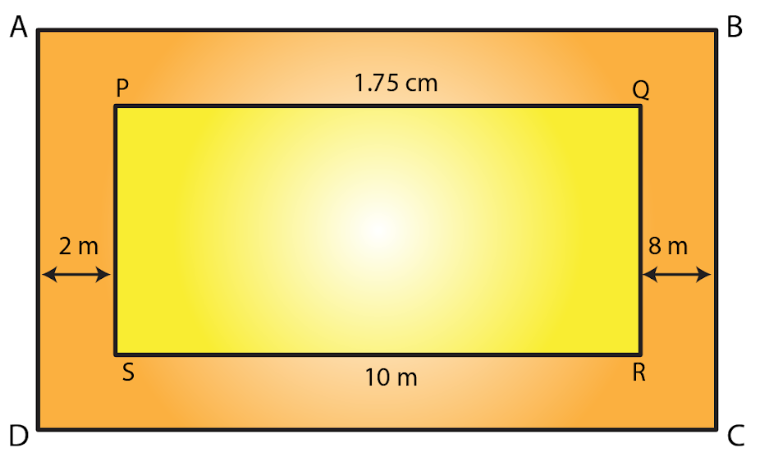Given length of poster = 10 cm and breadth of poster = 8 cm

Area of the poster = Length x Breadth

= 10 cm x 8 cm

= 80 cm2

From the figure, it is clear that

Length of the cardboard when the margin is included = 10 cm + 1.75 cm + 1.75 cm

= 13.5 cm

Breadth of the cardboard when the margin is included = 8 cm + 1.75 cm + 1.75 cm

= 11.5 cm

Area of the cardboard = Length x Breadth

= 13.5 cm x 11.5 cm

= 155.25 c m2

(i) Area of the margin = Area of cardboard including the margin – Area of the poster

= 155.25 c m2 – 80 c m2

= 75.25 c m2

(ii) Cost of the cardboard = Area of cardboard x Rate of the cardboard Rs 0.60 per cm2

= (155.25 x 0.60)

= Rs. 93.15

11. A rectangular field is 50 m by 40 m. It has two roads through its center, running parallel to its sides. The widths of the longer and shorter roads are 1.8 m and 2.5 m respectively. Find the area of the roads and the area of the remaining portion of the field.

Solution: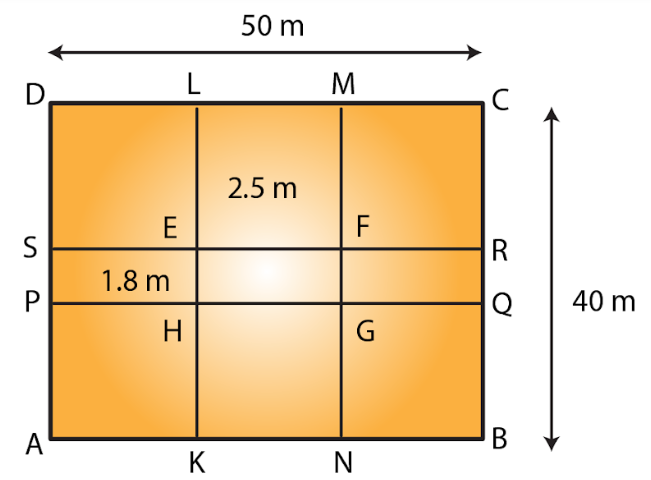Let ABCD be the rectangular field and KLMN and PQRS the two rectangular roads with width 1.8 m and 2.5 m, respectively.

Given that length of rectangular field = 50 m

Breadth of rectangular field = 40m

Area of rectangular field ABCD = 50 m x 40 m

= 2000 m2

Area of the road KLMN = 40 m x 2.5 m

= 100 m2

Area of the road PQRS = 50 m x 1.8 m

= 90 m2

Clearly area of EFGH is common to the two roads.

Thus, Area of EFGH = 2.5 m x 1.8 m = 4.5 m2

Hence, Area of the roads = Area of KLMN + Area of PQRS – Area of EFGH

= (100 m2 + 90 m2) – 4.5 m2

= 185.5 m2

Area of the remaining portion of the field = Area of the rectangular field ABCD – Area of the roads

= (2000 – 185.5) m2

= 1814.5 m2

12. There is a rectangular field of size 94 m x 32 m. Three roads each of 2 m width pass through the field such that two roads are parallel to the breadth of the field and the third is parallel to the length. Calculate: (i) area of the field covered by the three roads (ii) area of the field not covered by the roads.

Solution: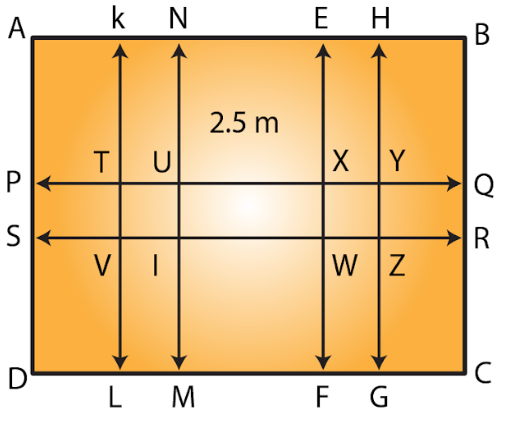Let ABCD be the rectangular field.

From the figure it is clear that two roads which are parallel to the breadth of the field KLMN and EFGH with width 2 m each.

One road which is parallel to the length of the field PQRS with width 2 m.

We have from the question, length of the rectangular field AB = 94 m and breadth of the rectangular field BC = 32 m

Area of the rectangular field = Length x Breadth

= 94 m x 32 m

= 3008 m2

Area of the road KLMN = 32 m x 2 m

= 64 m2

Area of the road EFGH = 32 m x 2 m

= 64 m2

Area of the road PQRS = 94 m x 2 m

= 188 m2

Clearly area of TUVI and WXYZ is common to these three roads.

Thus, Area of TUV1 = 2 m x 2 m = 4 m2

Area of WXYZ = 2 m x 2 m = 4 m2

(i) Area of the field covered by the three roads = Area of KLMN + Area of EFGH + Area (PQRS) – (Area of TUVI + Area of WXYZ)

= [64+ 64 + 188 – (4 + 4)] m2

= 316 m2 – 8 m2

= 308 m2

(ii) Area of the field not covered by the roads = Area of the rectangular field ABCD – Area of the field covered by the three roads

= 3008 m2 – 308 m2

= 2700 m2

13. A school has a hall which is 22 m long and 15.5 m broad. A carpet is laid inside the hall leaving all around a margin of 75 cm from the walls. Find the area of the carpet and the area of the strip left uncovered. If the width of the carpet is 82 cm, find the cost at the rate of Rs 18 per meter.

Solution: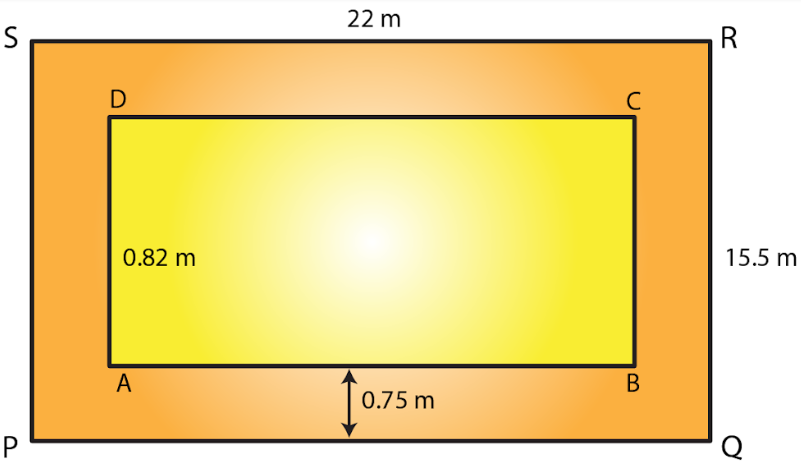Given that length of hall PQ = 22 m and breadth of hall QR = 15.5 m

Area of the school hall PQRS = 22 m x 15.5 m

= 341 m2

Length of the carpet AB = 22 m – (0.75 m + 0.75 m)

= 20.5 m [Since 100 cm = 1 m]

Breadth of the carpet BC = 15.5 m – (0.75 m + 0.75 m)

= 14 m

Area of the carpet ABCD = 20.5 m x 14 m

= 287 m2

Area of the strip = Area of the school hall PQRS – Area of the carpet ABCD

= 341 m2 – 287 m2

= 54 m2

Now, area of the 1 m length of carpet = 1 m x 0.82 m

= 0.82 m2

Thus, Length of the carpet whose area is 287 m2 = 287 m+ 0.82 m2

= 350 m

Cost of the 350 m long carpet = Rs. 18 x 350

= Rs. 6300

14. Two cross roads, each of width 5 m, run at right angles through the center of a rectangular park of length 70 m and breadth 45 m parallel to its sides. Find the area of the roads. Also, find the cost of constructing the roads at the rate of Rs 105 per m2.

Solution: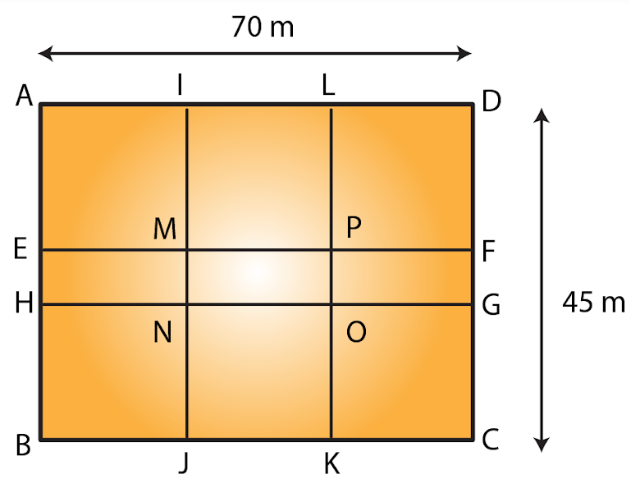Let ABCD be the rectangular park then EFGH and IJKL are the two rectangular roads with width 5m.

Given that length of rectangular park = 70m

Breadth of rectangular park = 45m

Area of the rectangular park = Length x Breadth

= 70 m x 45 m

= 3150 m2

Area of the road EFGH = 70 m x 5 m

= 350 m2

Now, Area of the road JKIL = 45 m x 5 m

= 225 m2

From the figure, it is clear that area of MNOP is common to the two roads.

Thus, Area of MNOP = 5 m x 5 m = 25 m2

Therefore,

Area of the roads = Area of EFGH + Area of JKIL – Area of MNOP

= (350 + 225) m2– 25 m2

= 550 m2

Again, it is given that the cost of constructing the roads = Rs. 105 per m2

Therefore,

Cost of constructing 550 m2 area of the roads

= Rs. (105 x 550)

= Rs. 57750.

15. The length and breadth of a rectangular park are in the ratio 5: 2. A 2.5 m wide path running all around the outside the park has an area 305 m2. Find the dimensions of the park.

Solution: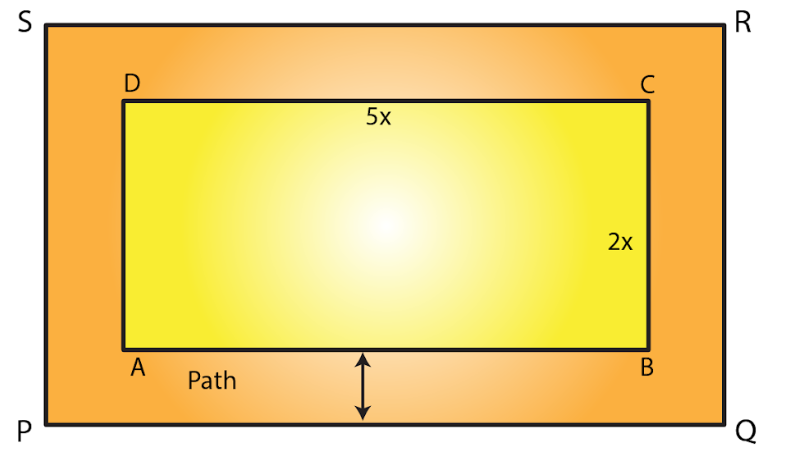Given that area of the park be 305m2.

Let the length of the park be 5x m and breadth be 2x m.

Therefore,

Area of the rectangular park = (5x) x (2x)

= 10x2 m2

Width of the path = 2.5 m

From the figure,

Outer length PQ = 5x m + 2.5 m + 2.5 m

= (5x + 5) m

Outer breadth QR = 2x + 2.5 m + 2.5 m

= (2x + 5) m

Area of PQRS = (5x + 5) m x (2x + 5) m

= (10x2 + 25x + 10x + 25) m2

= (10x2 + 35x + 25) m2

Area of the path = [(10x2 + 35x + 25) – 10x2] m2

By solving above equation we get

305 = 35x + 25

305 – 25 = 35x

280 = 35x

x = 280/35 = 8

Therefore,

Length of the park = 5x

= 5 x 8 = 40 m

Breadth of the park = 2x

= 2 x 8 = 16 m

16. A square lawn is surrounded by a path 2.5 m wide. If the area of the path is 165 m2, find the area of the lawn.

Solution: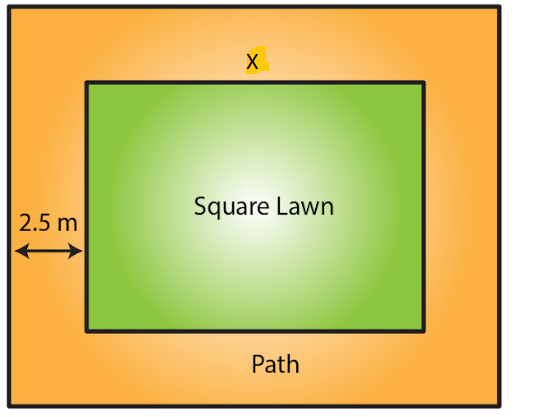Let the side of lawn be x m.

Given that width of the path = 2.5 m

Side of the lawn including the path = (x + 2.5 + 2.5) m

= (x + 5) m

Therefore, area of lawn = (Area of the lawn including the path) – (Area of the path)

We know that the area of a square = (Side)2

Area of lawn (x2) = (x + 5)2 – 165

x2 = (x2 + 10x + 25) – 165

165 = 10x + 25

165 – 25 =10x

140 = 10x

Therefore x = 140/10 = 14

Thus the side of the lawn = 14 m

Therefore,

The area of the lawn = (14 m) 2

= 196 m2

Exercise 20.3 Page No: 20.20

1. Find the area of a parallelogram with base 8 cm and altitude 4.5 cm.

Solution:

Given base = 8 cm and altitude = 4.5 cm

We know that area of the parallelogram = Base x Altitude

= 8 cm x 4.5 cm

Therefore, area of parallelogram = 36 cm2

2. Find the area in square meters of the parallelogram whose base and altitudes are as under

(i) Base =15 dm, altitude = 6.4 dm

(ii) Base =1 m 40 cm, altitude = 60 cm

Solution:

(i) Given base =15 dm, altitude = 6.4 dm

By converting these to standard form we get,

Base = 15 dm = (15 x 10) cm = 150 cm = 1.5 m

Altitude = 6.4 dm = (6.4 x 10) cm = 64 cm = 0.64 m

We know that area of the parallelogram = Base x Altitude

= 1.5 m x 0.64 m

Area of parallelogram = 0.96 m2

(ii) Given base = 1 m 40 cm = 1.4 m [Since 100 cm = 1 m]

Altitude = 60 cm = 0.6 m [Since 100 cm = 1 m]

We know that area of the parallelogram = Base x Altitude

= 1.4 m x 0.6 m

= 0.84 m2

3.  Find the altitude of a parallelogram whose area is 54 d m2 and base is 12 dm.

Solution:

Given area of the given parallelogram = 54 d m2

Base of the given parallelogram = 12 dm

We know that area of the parallelogram = Base x Altitude

Therefore altitude of the given parallelogram = Area/Base

= 54/12 dm

= 4.5 dm

4. The area of a rhombus is 28 m2. If its perimeter be 28 m, find its altitude.

Solution:

Given perimeter of a rhombus = 28 m

But we know that perimeter of a rhombus = 4 (Side)

4(Side) = 28 m

Side = 28/4

Side = 7m

Now, Area of the rhombus = 28 m2

But we know that area of rhombus = Side x Altitude

(Side x Altitude) = 28 m2

(7 x Altitude) = 28 m2

Altitude = 28/7 = 4 m

5. In Fig. 20, ABCD is a parallelogram, DL ⊥ AB and DM ⊥ BC. If AB = 18 cm, BC =12 cm and DM= 9.3 cm, find DL.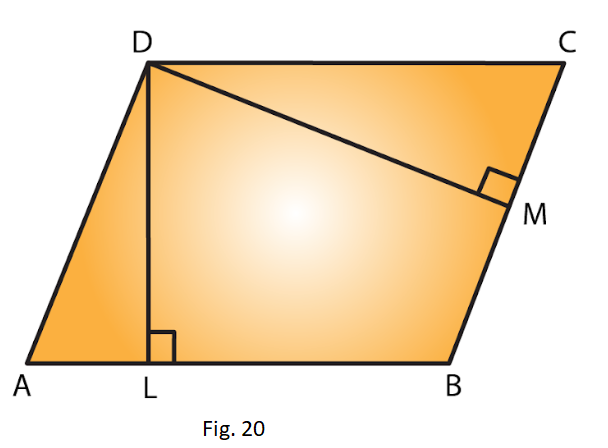Solution:

Given DL ⊥ AB and DM ⊥ BC

Taking BC as the base, BC = 12 cm and altitude DM = 9.3 cm

We know that area of parallelogram ABCD = Base x Altitude

= (12 cm x 9.3 cm)

= 111.6 c m2 ….. Equation (i)

Now, by taking AB as the base,

We have, Area of the parallelogram ABCD = Base x Altitude

= (18 cm x DL) ….. Equation (ii)

From (i) and (ii), we have

18 cm x DL = 111.6 c m2

DL = 111.6/218

= 6.2 cm

6. The longer side of a parallelogram is 54 cm and the corresponding altitude is 16 cm. If the altitude corresponding to the shorter side is 24 cm, find the length of the shorter side.

Solution: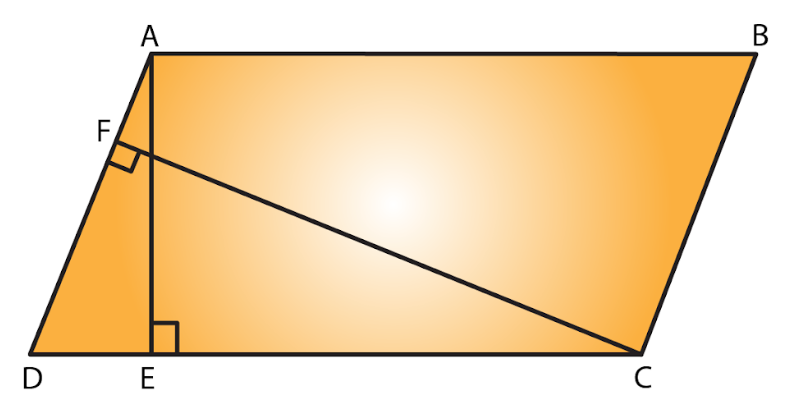Let ABCD is a parallelogram with the longer side AB = 54 cm and corresponding altitude AE = 16 cm.

The shorter side is BC and the corresponding altitude is CF = 24 cm.

We know that area of a parallelogram = base x height.

We have two altitudes and two corresponding bases.

By equating them we get,

½ x BC x CF = ½ x AB x AE

On simplifying, we get

BC x CF = AB x AE

BC x 24 = 54 x 16

BC = (54 × 16)/24

= 36 cm

Hence, the length of the shorter side BC = AD = 36 cm.

7. In Fig. 21, ABCD is a parallelogram, DL ⊥ AB. If AB = 20 cm, AD = 13 cm and area of the parallelogram is 100 c m2, find AL.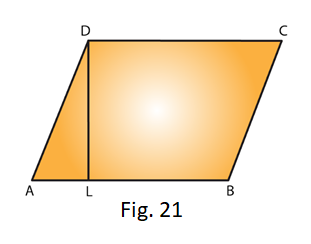Solution:

From the figure we have ABCD is a parallelogram with base AB = 20 cm and corresponding altitude DL.

It is given that the area of the parallelogram ABCD = 100 c m2

We know that the area of a parallelogram = Base x Height

Therefore,

100 = AB x DL

100 = 20 x DL

DL = 100/20 = 5 cm

By observing the picture it is clear that we have to apply the Pythagoras theorem,

Therefore by Pythagoras theorem, we have,

(13)2 = (AL)2+ (5)2

(AL)2 = (13)2 – (5)2

(AL)2 = 169 – 25

= 144

We know that 122 = 44

(AL)2 = (12)2

AL = 12 cm

Hence, length of AL is 12 cm.

8. In Fig. 21, if AB = 35 cm, AD= 20 cm and area of the parallelogram is 560 cm2, find LB.Solution:

From the figure, ABCD is a parallelogram with base AB = 35 cm and corresponding altitude DL.

It is given that the area of the parallelogram ABCD = 560 cm2

Now, Area of the parallelogram = Base x Height

560 cm2 = AB x DL

560 cm2 = 35 cm x DL

DL = 560/235

= 16 cm

Again by Pythagoras theorem, we have, (AD)2 = (AL)2 + (DL)2

(20)2= (AL)2 + (16)2

(AL)= (20)– (16)2

= 400 – 256

= 144

(AL)2 = (12)2

AL = 12 cm

From the figure, AB = AL + LB

35 = 12 + LB

LB = 35 – 12 = 23 cm

Hence, length of LB is 23 cm.

9. The adjacent sides of a parallelogram are 10 m and 8 m. If the distance between the longer sides is 4 m, find the distance between the shorter sides

Solution: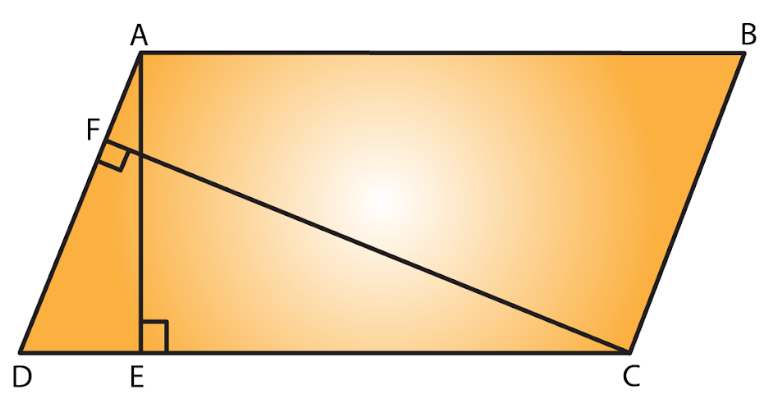Let ABCD is a parallelogram with side AB = 10 m and corresponding altitude AE = 4 m.

The adjacent side AD = 8 m and the corresponding altitude is CF.

We know that area of a parallelogram = Base x Height

We have two altitudes and two corresponding bases.

So, equating them we get

AD x CF = AB x AE

= 8 x CF = 10 x 4

CF = (10 x 4)/8 = 5 m

Hence, the distance between the shorter sides is 5 m.

10. The base of a parallelogram is twice its height. If the area of the parallelogram is 512 cm2, find the base and height.

Solution:

Let the height of the parallelogram be x cm.

Then the base of the parallelogram is 2x cm. [from given data]

Given that the area of the parallelogram = 512 cm2

We know that area of a parallelogram = Base x Height

512 = (2x) (x)

512 = 2x2

x= 512/2

= 256 cm2

x2 = (16)2

x = 16 cm

Hence height of parallelogram = x = 16cm

And base of the parallelogram = 2x = 2 x 16 = 32 cm

11. Find the area of a rhombus having each side equal to 15 cm and one of whose diagonals is 24 cm.

Solution: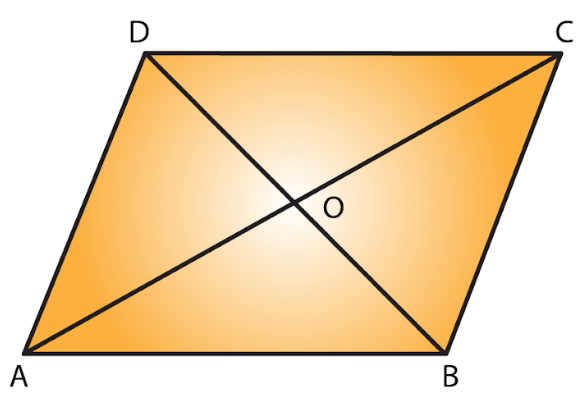Let ABCD be the rhombus where diagonals intersect at 0 as given in the figure.

Then AB = 15 cm and AC = 24 cm.

The diagonals of a rhombus bisect each other at right angles.

Therefore, from the figure triangle A0B is a right-angled triangle, right angled at O such that

Therefore, OA = ½(AC) = ½ (24) = 12 cm and AB = 15 cm.

By applying Pythagoras theorem, we get,

(AB)2 = (OA)2 + (OB)2

(15)2 = (12)2 + (OB)2

(OB)2= (15)2– (12)2

(OB)= 225 – 144

= 81

(OB)2 = (9)2

OB = 9 cm

BD = 2 x OB

= 2 x 9

= 18 cm

Hence, Area of the rhombus ABCD = (½ x AC x BD)

= (1/2 x 24 x 18)

= 216 cm2

12. Find the area of a rhombus, each side of which measures 20 cm and one of whose diagonals is 24 cm.

Solution:Let ABCD be the rhombus whose diagonals intersect at 0.

Then AB = 20 cm and AC = 24 cm by given data

The diagonals of a rhombus bisect each other at right angles.

Therefore Triangle AOB is a right-angled triangle, right angled at O

Such that;

OA = ½ (AC) = ½ (24) = 12 cm and AB =20 cm

By Pythagoras theorem, we have,

(AB)2 = (OA)2+ (OB) 2

(20)2 = (12)2 + (OB) 2

(OB) 2= (20) 2– (12) 2

(OB)= 400 – 144

= 256

(OB) 2 = (16) 2

OB = 16 cm

BD =2 x OB

= 2 x 16 cm

= 32 cm

Hence, Area of the rhombus ABCD = ½ x AC x BD

= ½ x 24 x 32

Area of rhombus ABCD = 384 cm2

13. The length of a side of a square field is 4 m. What will be the altitude of the rhombus, if the area of the rhombus is equal to the square field and one of its diagonals is 2 m?

Solution: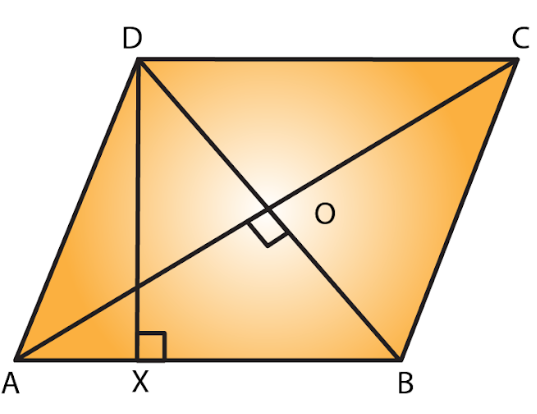Given the length of a side of a square field is 4m

Also given that,

Area of the rhombus = Area of the square of side

½ x AC x BD = 4 m2

½ x AC x 2 = 16 m2

AC = 16 m

We know that the diagonals of a rhombus are perpendicular bisectors of each other.

= AO = ½ (AC) = ½ (16) = 8 m and BO = ½ (BD) = ½ (2) = 1 m

By Pythagoras theorem, we can write as

AO2 + BO2 = AB2

AB2 = (8 m) 2 + (1 m) 2

= 64 m2 + 1 m2 = 65 m2

Side of a rhombus = AB = √65 m.

Let DX be the altitude.

Area of the rhombus = AB x DX

16 m2 = √65m × DX

DX = 16/ (√65) m

Hence, the altitude of the rhombus will be 16/√65 m.

14. Two sides of a parallelogram are 20 cm and 25 cm. If the altitude corresponding to the sides of length 25 cm is 10 cm, find the altitude corresponding to the other pair of sides.

Solution: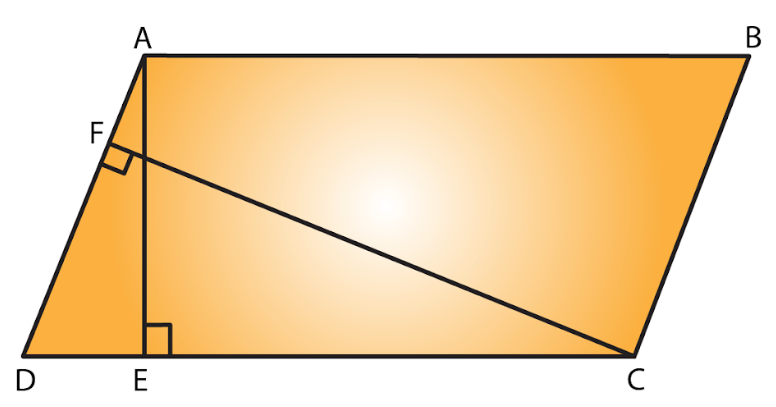Let ABCD is a parallelogram with longer side AB = 25 cm and altitude AE = 10 cm.

Therefore AB = CD (opposite sides of parallelogram are equal).

The shorter side is AD = 20 cm and the corresponding altitude is CF.

We know that area of a parallelogram = Base x Height

We have two altitudes and two corresponding bases.

So, by equating them

AD x CF = CD x AE

20 x CF = 25 x 10

CF = 12.5 cm

Hence, the altitude corresponding to the other pair of the side AD is 12.5 cm.

15. The base and corresponding altitude of a parallelogram are 10 cm and 12 cm respectively. If the other altitude is 8 cm, find the length of the other pair of parallel sides.

Solution: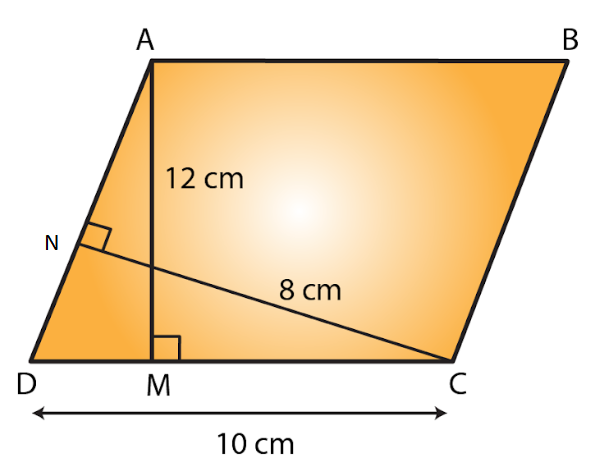Let ABCD is a parallelogram with side AB = CD = 10 cm (since opposite sides of parallelogram are equal) and corresponding altitude AM = 12 cm.

The other side is AD and the corresponding altitude is CN = 8 cm.

We know that area of a parallelogram = Base x Height

We have two altitudes and two corresponding bases.

So, by equating them we get

AD x CN = CD x AM

AD x 8 = 10 x 12

AD = (10×12)/8 = 15 cm

Hence, the length of the other pair of the parallel side = 15 cm.

16. A floral design on the floor of a building consists of 280 tiles. Each tile is in the shape of a parallelogram of altitude 3 cm and base 5 cm. Find the cost of polishing the design at the rate of 50 paise per cm2.

Solution:

Given altitude of a tile = 3 cm

Base of a tile = 5 cm

Area of one tile = Attitude x Base

= 5 cm x 3 cm

= 15 c m2

Area of 280 tiles = 280 x 15 c m2 =

4200 c m2

Rate of polishing the tile = Rs. 0.5 per cm2

Thus, Total cost of polishing the design = 4200 x 0.5

= Rs. 2100

Exercise 20.4 Page No: 20.26

1. Find the area in square centimetres of a triangle whose base and altitude are as under:

(i) Base =18 cm, altitude = 3.5 cm

(ii) Base = 8 dm, altitude =15 cm

Solution:

(i) Given base = 18 cm and height = 3.5 cm

We know that the area of a triangle = ½ (Base x Height)

Therefore area of the triangle = ½ x 18 x 3.5

= 31.5 cm2

(ii) Given base = 8 dm = (8 x 10) cm = 80 cm [Since 1 dm = 10 cm]

And height = 3.5 cm

We know that the area of a triangle = ½ (Base x Height)

Therefore area of the triangle = ½ x 80 x 15

= 600 cm2

2. Find the altitude of a triangle whose area is 42 cm2 and base is 12 cm.

Solution:

Given base = 12 cm and area = 42 cm2

We know that the area of a triangle = ½ (Base x Height)

Therefore altitude of a triangle = (2 x Area)/Base

Altitude = (2 x 42)/12

= 7 cm

3. The area of a triangle is 50 cm2. If the altitude is 8 cm, what is its base?

Solution:

Given, altitude = 8 cm and area = 50 cm2

We know that the area of a triangle = ½ (Base x Height)

Therefore base of a triangle = (2 x Area)/ Altitude

Altitude = (2 x 50)/ 8

= 12.5 cm

4. Find the area of a right angled triangle whose sides containing the right angle are of lengths 20.8 m and 14.7 m.

Solution:

In a right-angled triangle,

The sides containing the right angles are of lengths 20.8 m and 14.7 m.

Let the base be 20.8 m and the height be 141 m.

Then,

Area of a triangle = 1/2 (Base x Height)

= 1/2 (20.8 × 14.7)

= 152.88 m2

5. The area of a triangle, whose base and the corresponding altitude are 15 cm and 7 cm, is equal to area of a right triangle whose one of the sides containing the right angle is 10.5 cm. Find the other side of this triangle.

Solution:

For the first triangle, given that

Base = 15 cm and altitude = 7 cm

We know that area of a triangle = ½ (Base x Altitude)

= ½ (15 x 7)

= 52.5 cm2

It is also given that the area of the first triangle and the second triangle are equal.

Area of the second triangle = 52.5 c m2

One side of the second triangle = 10.5 cm

Therefore, The other side of the second triangle = (2 x Area)/One side of a triangle

= (2x 52.5)/10.5

=10 cm

Hence, the other side of the second triangle will be 10 cm.

6. A rectangular field is 48 m long and 20 m wide. How many right triangular flower beds, whose sides containing the right angle measure 12 m and 5 m can be laid in this field?

Solution:

Given length of the rectangular field = 48 m

Breadth of the rectangular field = 20 m

Area of the rectangular field = Length x Breadth

= 48 m x 20 m

= 960 m2

Area of one right triangular flower bed = ½ (12 x 5) = 30 m2

Therefore, required number of right triangular flower beds = area of the rectangular field/ area of one right triangular flower bed.

= 960/30

Number of right triangular flower bed = 32

7. In Fig. 29, ABCD is a quadrilateral in which diagonal AC = 84 cm; DL ⊥AC, BM ⊥ AC, DL = 16.5 cm and BM = 12 cm. Find the area of quadrilateral ABCD.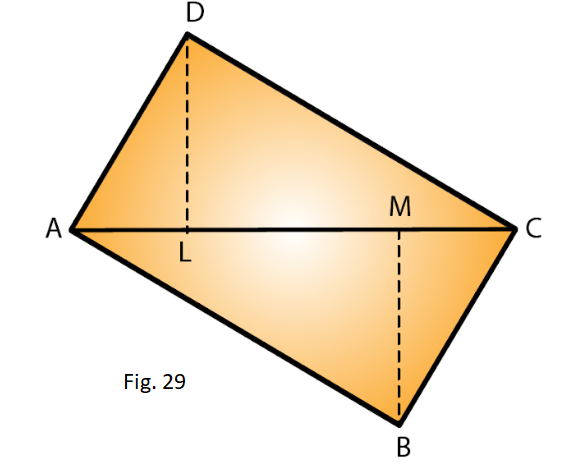Solution:

Given AC = 84 cm, DL = 16.5 cm and BM = 12 cm

We know that area of triangle = ½ x base x height

Area of triangle ADC = ½ (AC x DL)

= ½ (84 x 16.5)

= 693 cm2

Area of triangle ABC = ½ (AC x BM)

= ½ (84 x 12) = 504 cm2

Hence, Area of quadrilateral ABCD = Area of ADC + Area of ABC

= (693 + 504) cm2

= 1197 cm2

8. Find the area of the quadrilateral ABCD given in Fig. 30. The diagonals AC and BD measure 48 m and 32 m respectively and are perpendicular to each other.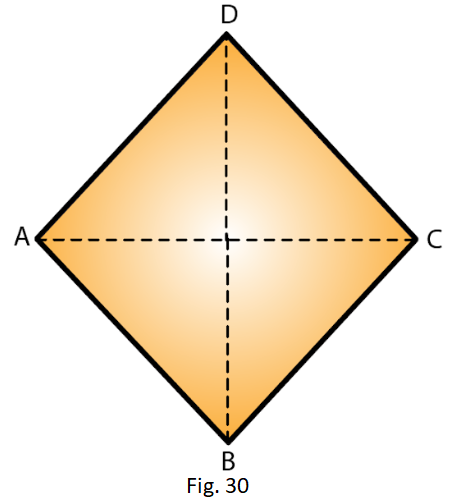Solution:

Given diagonal AC = 48 cm and diagonal BD = 32 m

Area of a quadrilateral = ½ (Product of diagonals)

= ½ (AC x BD)

= ½ (48 x 32) m2

= 768 m2

9.  In Fig 31, ABCD is a rectangle with dimensions 32 m by 18 m. ADE is a triangle such that EF⊥ AD and EF= 14 cm. Calculate the area of the shaded region.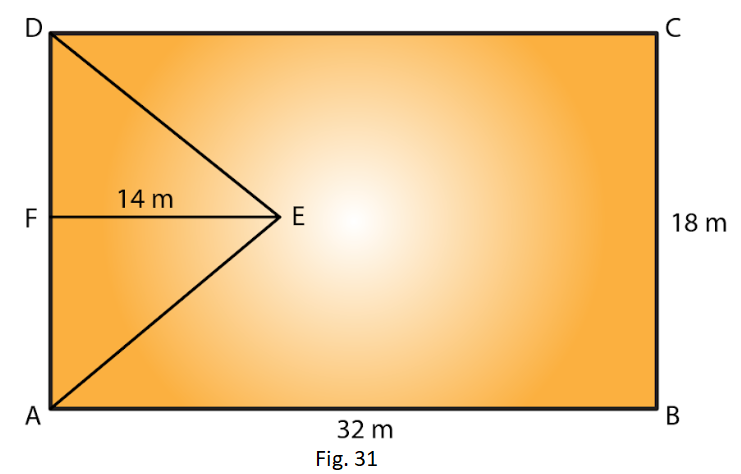Solution:

Given length of rectangle = 32m and breadth = 18m

We know that area of rectangle = length x breadth

Therefore area of the rectangle = AB x BC

= 32 m x 18 m

= 576 m2

Also given that base of triangle = 18m and height = 14m and EF⊥ AD

We know that area of triangle = ½ x base x height

Area of the triangle = ½ (AD x FE)

= ½ (BC x FE) [Since AD = BC]

= ½ (18 m x 14 m)

= 126 m2

Area of the shaded region = Area of the rectangle – Area of the triangle

= (576 – 126) m2

= 450 m2

10. In Fig. 32, ABCD is a rectangle of length AB = 40 cm and breadth BC = 25 cm. If P, Q, R, S be the mid-points of the sides AB, BC, CD and DA respectively, find the area of the shaded region.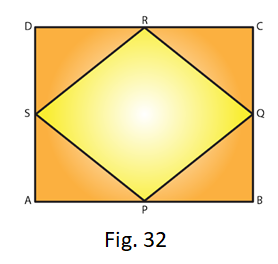Solution: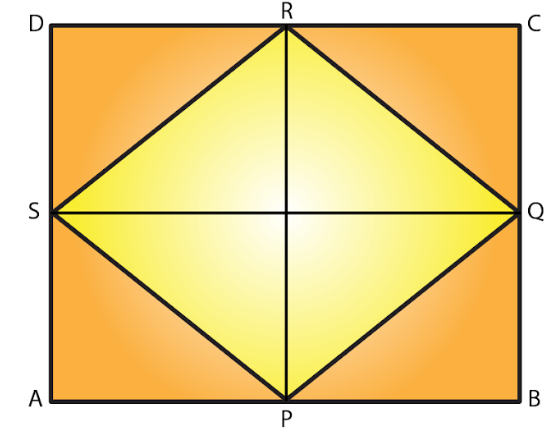Given ABCD is a rectangle of length AB = 40 cm and breadth BC = 25 cm.

Join PR and SQ so that these two lines bisect each other at point O

Also OP = OR = RP/2

= 25/2

= 12.5 cm

From the given figure it is clear that,

Area of Triangle SPQ = Area of Triangle SRQ

Hence, area of the shaded region = 2 x (Area of SPQ)

= 2 x (1/2 (SQ x OP))

= 2 x (1/2 (40 x 12.5))

= 500 cm2

11.  Calculate the area of the quadrilateral ABCD as shown in Fig.33, given that BD = 42 cm, AC = 28 cm, OD = 12 cm and AC ⊥ BO.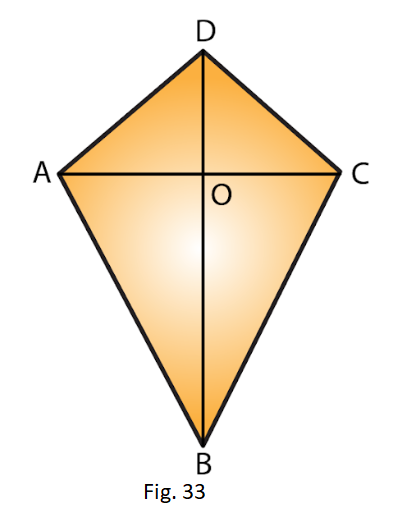Solution:

BD = 42 cm, AC = 28 cm, OD= 12 cm

Area of Triangle ABC = 1/2 (AC x OB)

= 1/2 (AC x (BD – OD))

= 1/2 (28 cm x (42 cm – 12 cm))

= 1/2 (28 cm x 30 cm)

= 14 cm x 30 cm

= 420 cm2

Area of Triangle ADC = 1/2 (AC x OD) = 1/2 (28 cm x 12 cm)

= 14 cm x 12 cm

= 168 cm2

Hence, Area of the quadrilateral ABCD = Area of ABC + Area of ADC

= (420 + 168) cm2

= 588 cm2

12. Find the area of figure formed by a square of side 8 cm and an isosceles triangle with base as one side of the square and perimeter as 18 cm.

Solution: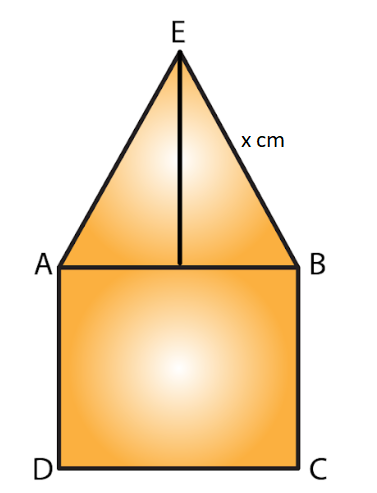Let x cm be one of the equal sides of an isosceles triangle.

Given that the perimeter of the isosceles triangle = 18 cm

Then, x + x + 8 =18

2x = (18 – 8) = 10 cm

2x = 10

x = 5 cm

Area of the figure formed = Area of the square + Area of the isosceles triangle

= (side of square)2 + ½ (base x √[(equal side)2 – ¼ x (base)2]

= 82 + ½ (8) x √ [52 – ¼ x 82]

= 64 + 4 x √ [25 – ¼ x 64]

= 64 + 4 x √ (25 – 16)

= 64 + 4 x √9

= 64 + 4 x 3

= 64 + 12

= 76 cm2

13. Find the area of Fig. 34, in the following ways: (i) Sum of the areas of three triangles (ii) Area of a rectangle — sum of the areas of five triangles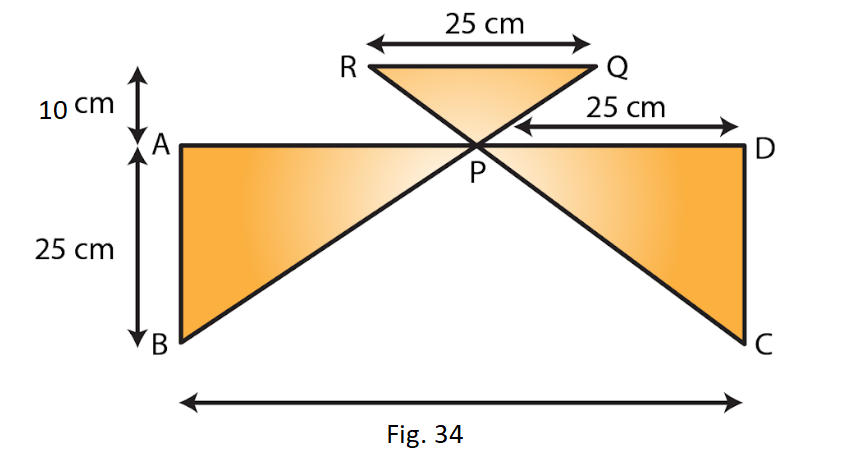Solution:

(i) From the figure, P is the midpoint of AD.

Thus AP = PD = 25 cm and AB = CD = 20 cm

From the figure, we observed that,

Area of Triangle APB = Area of Triangle PDC

Area of Triangle APB = ½ (AB x AP)

= ½ (20 x 25)

= 250 cm2

Area of Triangle PDC = Area of Triangle APB = 250 c m2

Area of Triangle RPQ = ½ (Base x Height)

= ½ (25 cm x 10 cm)

= 125 cm2

Hence, Sum of the three triangles = (250 + 250 + 125) cm2

= 625 cm2

(ii) From the figure, area of the rectangle ABCD = 50 cm x 20 cm

= 1000 cm2

Thus, Area of the rectangle – Sum of the areas of three triangles

= (1000 – 625) cm2

= 375 cm2

14. Calculate the area of quadrilateral field ABCD as shown in Fig.35, by dividing it into a rectangle and a triangle.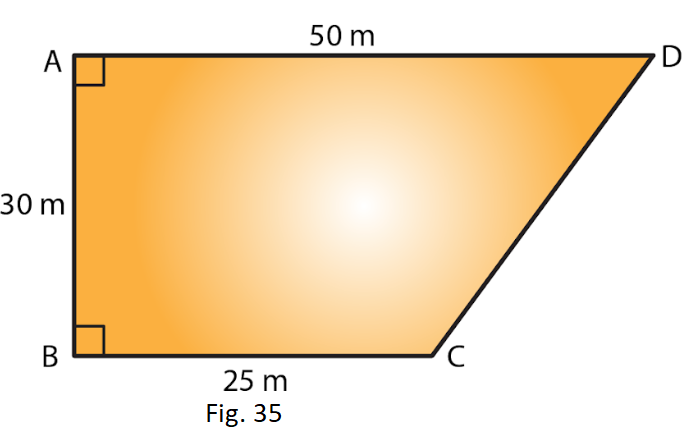Solution: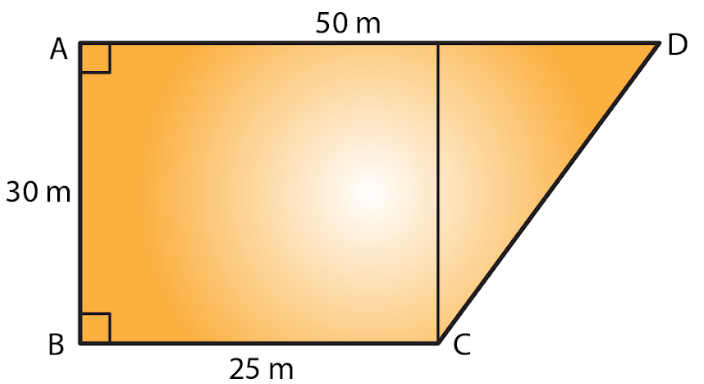Join CE, so that which intersect AD at point E.

Given AE = ED = BC = 25 m and EC = AB = 30 m

Area of rectangle = length x breadth

Area of the rectangle ABCE = AB x BC

= 30 m x 25 m

= 750 m2

Area of triangle = ½ x base x height

Area of Triangle CED = ½ (EC x ED)

= ½ (30 m x 25 m)

= 375 m2

Hence, Area of the quadrilateral ABCD = (750 + 375) m2 = 1125 m2

15. Calculate the area of the pentagon ABCDE, where AB = AE and with dimensions as shown in Fig. 36.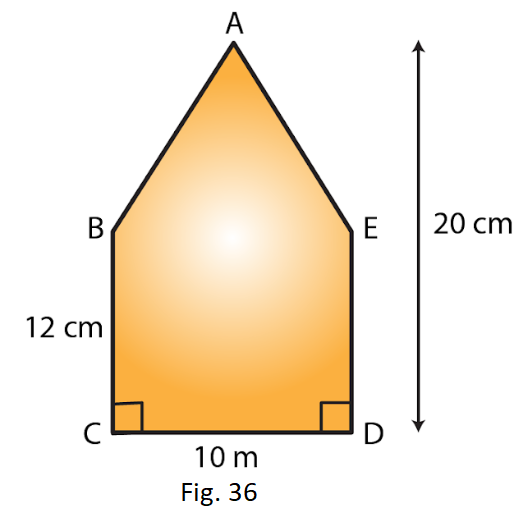Solution: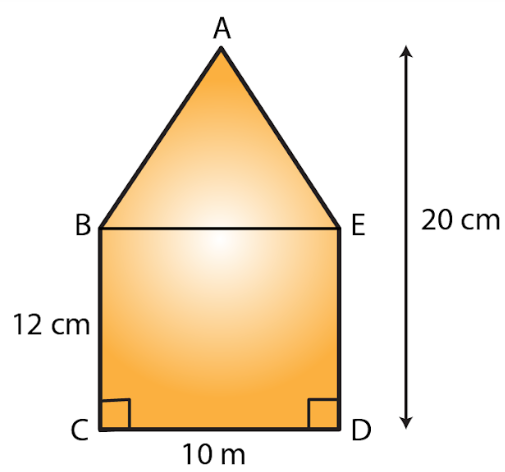Join BE so that we can get rectangle and triangle.

We know that area of rectangle = length x breadth

Area of the rectangle BCDE = CD x DE

= 10 cm x 12 cm

= 120 c m2

Area of triangle = ½ x base x height

Area of Triangle ABE = 1/2 (BE x height of the triangle)

= ½ (10 cm x (20 – 12) cm)

= ½ (10 cm x 8 cm)

= 40 cm2

Hence, Area of the pentagon ABCDE = area of rectangle + area of triangle

= (120 + 40) cm2

= 160 cm2

16. The base of a triangular field is three times its altitude. If the cost of cultivating the field at Rs 24.60 per hectare is Rs 332.10, find its base and height.

Solution:

Let altitude of the triangular field be h m

Then base of the triangular field is 3h m.

We know that area of triangle = ½ x b x h

Area of the triangular field = ½ (h x 3h) = 3h2/2 m2 ……. (i)

The rate of cultivating the field is Rs 24.60 per hectare.

Therefore,

Area of the triangular field = 332.10 /24.60

= 13.5 hectare

= 135000 m2 [Since 1 hectare = 10000 m2] …… (ii)

From equation (i) and (ii) we have,

3h2/2 = 135000 m2

3h2 = 135000 x 2 = 270000 m2

h2 = 270000/3

= 90000 m2

= (300)2

h = 300 m

Hence, Height of the triangular field = 300 m and

Base of the triangular field = 3 x 300 m = 900 m

17. A wall is 4.5 m long and 3 m high. It has two equal windows, each having form and dimensions as shown in Fig. 37. Find the cost of painting the wall (leaving windows) at the rate of Rs 15 per m2.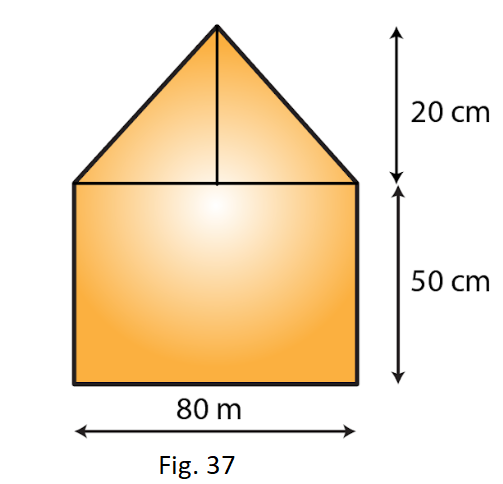Solution:

Given length of a wall = 4.5 m

Breadth of the wall = 3 m

We know that the area of triangle = length x Breadth

Area of the wall = Length x Breadth

= 4.5 m x 3 m = 13.5 m2

From the figure we observed that,

Area of the window = Area of the rectangle + Area of the triangle

= (0.8 m x 0.5 m) + (12 x 0.8 m x 0.2 m) [Since 1 m = 100 cm]

= 0.4 m2 + 0.08 m2

= 0.48 m2

Area of two windows = 2 x 0.48 = 0.96 m2

Area of the remaining wall (leaving windows) = (13.5 – 0.96) m2

= 12.54 m2

Cost of painting the wall per m2 = Rs. 15

Hence, the cost of painting on the wall = Rs. (15 x 12.54)

= Rs. 188.1